# JPWO2004042691A1 - Sample hold circuit and image display apparatus using the same - Google Patents

Sample hold circuit and image display apparatus using the same Download PDF

## Info

Publication number
JPWO2004042691A1
JPWO2004042691A1 JP2005502149A JP2005502149A JPWO2004042691A1 JP WO2004042691 A1 JPWO2004042691 A1 JP WO2004042691A1 JP 2005502149 A JP2005502149 A JP 2005502149A JP 2005502149 A JP2005502149 A JP 2005502149A JP WO2004042691 A1 JPWO2004042691 A1 JP WO2004042691A1
Authority
JP
Japan
Prior art keywords
potential
circuit
node
type transistor
electrode
Prior art date
Legal status (The legal status is an assumption and is not a legal conclusion. Google has not performed a legal analysis and makes no representation as to the accuracy of the status listed.)
Withdrawn
Application number
JP2005502149A
Other languages
Japanese (ja)
Inventor

Original Assignee

Priority date (The priority date is an assumption and is not a legal conclusion. Google has not performed a legal analysis and makes no representation as to the accuracy of the date listed.)
Filing date
Publication date
Priority to JP0211587 priority Critical
Priority to JPPCT/JP02/11587 priority
Priority to JPPCT/JP03/02757 priority
Priority to JP0302757 priority
Application filed by 三菱電機株式会社 filed Critical 三菱電機株式会社
Priority to PCT/JP2003/008249 priority patent/WO2004042691A1/en
Publication of JPWO2004042691A1 publication Critical patent/JPWO2004042691A1/en
Withdrawn legal-status Critical Current

• 239000003990 capacitor Substances 0.000 claims abstract description 201
• 239000004973 liquid crystal related substances Substances 0.000 claims abstract description 107
• 210000002858 crystal cell Anatomy 0.000 claims abstract description 53
• 244000171263 Ribes grossularia Species 0.000 claims description 303
• 230000000875 corresponding Effects 0.000 claims description 70
• 230000003334 potential Effects 0.000 claims description 66
• 230000001105 regulatory Effects 0.000 claims description 3
• 238000010586 diagrams Methods 0.000 description 194
• 230000004048 modification Effects 0.000 description 122
• 238000006011 modification reactions Methods 0.000 description 122
• 238000007599 discharging Methods 0.000 description 26
• 230000000694 effects Effects 0.000 description 25
• 230000001808 coupling Effects 0.000 description 24
• 238000010168 coupling process Methods 0.000 description 24
• 238000005859 coupling reactions Methods 0.000 description 24
• 230000003071 parasitic Effects 0.000 description 20
• 238000005513 bias potential Methods 0.000 description 12
• 230000000295 complement Effects 0.000 description 10
• 229910021417 amorphous silicon Inorganic materials 0.000 description 6
• 239000004065 semiconductors Substances 0.000 description 6
• 239000010409 thin films Substances 0.000 description 6
• 238000002834 transmittance Methods 0.000 description 6
• 101000005841 human Cyclin-dependent kinase 2 Proteins 0.000 description 4
• 239000011159 matrix materials Substances 0.000 description 4
• 239000000203 mixtures Substances 0.000 description 4
• 230000000630 rising Effects 0.000 description 4
• 230000000087 stabilizing Effects 0.000 description 4
• 238000005070 sampling Methods 0.000 description 3
• 238000006243 chemical reactions Methods 0.000 description 2
• 238000009792 diffusion process Methods 0.000 description 2
• 238000005401 electroluminescence Methods 0.000 description 2
• 239000010408 films Substances 0.000 description 2
• 239000011521 glasses Substances 0.000 description 2
• 239000007943 implant Substances 0.000 description 2
• 239000010410 layers Substances 0.000 description 2
• 239000002184 metals Substances 0.000 description 2
• 238000000034 methods Methods 0.000 description 2
• 239000000758 substrates Substances 0.000 description 2
• 230000001629 suppression Effects 0.000 description 2
• 241001157067 Leucoagaricus meleagris Species 0.000 description 1

## Images

•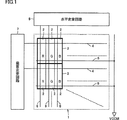•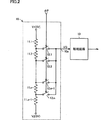•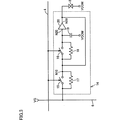•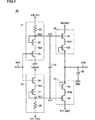•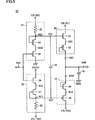•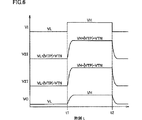••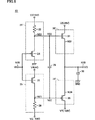•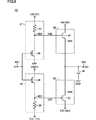•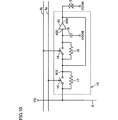•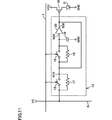•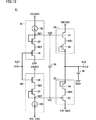•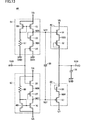•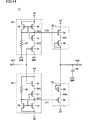••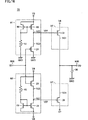•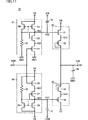••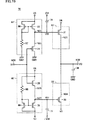••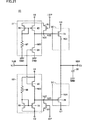•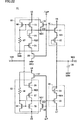•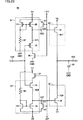•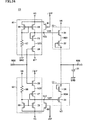•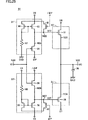•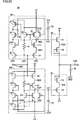••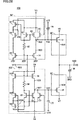•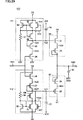•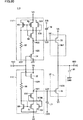•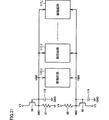•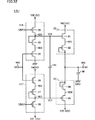•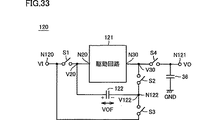••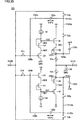•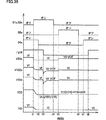•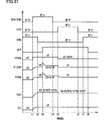•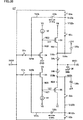••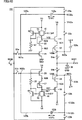•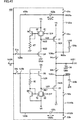••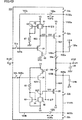••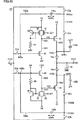•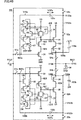•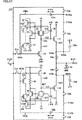•••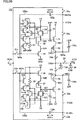•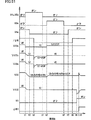•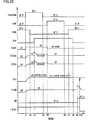•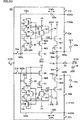•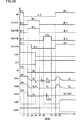••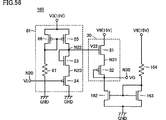•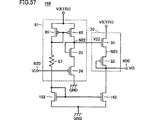•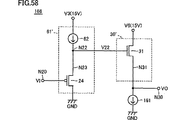•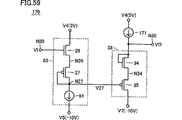•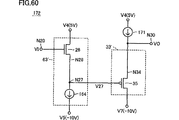•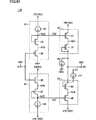••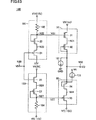•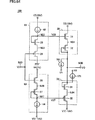•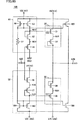•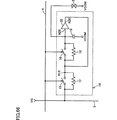•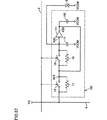•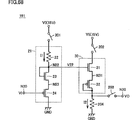•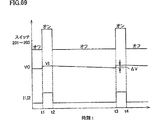•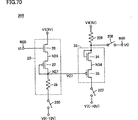•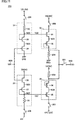•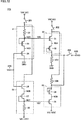•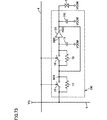•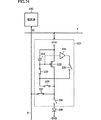•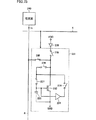•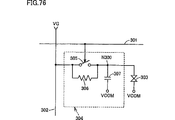## Classifications

• GPHYSICS
• G09EDUCATION; CRYPTOGRAPHY; DISPLAY; ADVERTISING; SEALS
• G09GARRANGEMENTS OR CIRCUITS FOR CONTROL OF INDICATING DEVICES USING STATIC MEANS TO PRESENT VARIABLE INFORMATION
• G09G3/00Control arrangements or circuits, of interest only in connection with visual indicators other than cathode-ray tubes
• G09G3/20Control arrangements or circuits, of interest only in connection with visual indicators other than cathode-ray tubes for presentation of an assembly of a number of characters, e.g. a page, by composing the assembly by combination of individual elements arranged in a matrix no fixed position being assigned to or needed to be assigned to the individual characters or partial characters
• G09G3/34Control arrangements or circuits, of interest only in connection with visual indicators other than cathode-ray tubes for presentation of an assembly of a number of characters, e.g. a page, by composing the assembly by combination of individual elements arranged in a matrix no fixed position being assigned to or needed to be assigned to the individual characters or partial characters by control of light from an independent source
• G09G3/36Control arrangements or circuits, of interest only in connection with visual indicators other than cathode-ray tubes for presentation of an assembly of a number of characters, e.g. a page, by composing the assembly by combination of individual elements arranged in a matrix no fixed position being assigned to or needed to be assigned to the individual characters or partial characters by control of light from an independent source using liquid crystals
• G09G3/3611Control of matrices with row and column drivers
• G09G3/3685Details of drivers for data electrodes
• G09G3/3688Details of drivers for data electrodes suitable for active matrices only

## Abstract

The sample and hold circuit (14) includes a first switch (15) connected between the data line (6) and the first node (N10), a first node (N10), a second node (N20), and The second switch (16) connected between the capacitor, the capacitor (19) connected between the second node (N20) and the common potential (VCOM) line, and the potential equal to the second node (N20). Is provided to the first node (N10) and one electrode of the liquid crystal cell (2). The first switch (15) and the second switch (16) conduct when the scanning line (4) is at the “H” level.

## Description

The present invention relates to a sample hold circuit and an image display device using the sample hold circuit, and more particularly to a sample hold circuit that samples an input potential, holds and outputs the sampled potential, and an image display device using the sample hold circuit.

FIG. 76 is a circuit diagram showing a main part of a conventional liquid crystal display device. In FIG. 76, in this liquid crystal display device, a liquid crystal cell 303 and a sample hold circuit 304 are arranged at the intersection of the scanning line 301 and the data line 302. The sample and hold circuit 304 includes a switch 305 and a capacitor 307. Switch 305 is connected between data line 302 and node N300, and conducts during a period of time when scanning line 301 is at the “H” level of the selection level. The switch 305 has a parasitic resistance. In FIG. 76, the parasitic resistance is indicated by a resistance element 306 connected in parallel to the switch 305. Capacitor 307 is connected between node N300 and the line of common potential VCOM. The liquid crystal cell 303 is connected between the node N300 and the common potential VCOM line.
When scanning line 301 is raised to the “H” level of the selection level, switch 305 is turned on and node N300 is charged to the potential of data line 302. When the scanning line 301 falls to the “L” level, which is a non-selection level, the switch 305 becomes non-conductive, and the potential of the node N300 is held by the capacitor 307. Liquid crystal cell 303 exhibits light transmittance in accordance with the potential of node N300.
However, in the conventional liquid crystal display device, when the potential of the data line 302 changes while the scanning line 301 is set to the “L” level, a leak occurs between the node N300 and the data line 302 via the resistance element 306. A current flows, and the potential of the node N300 changes. For this reason, it is necessary to refresh (rewrite) the potential of the node N300 in a predetermined cycle, and relatively large power is consumed.

Therefore, a main object of the present invention is to provide a sample-and-hold circuit in which a change in holding potential is small and an image display apparatus using the sample-and-hold circuit.
In the sample-and-hold circuit according to the present invention, one electrode receives an input potential, the first switching element that conducts in the first period, the one electrode is connected to the other electrode of the first switching element, and the second A second switching element that conducts during the period, a first capacitor whose one electrode is connected to the other electrode of the first switching element and whose other electrode receives a predetermined potential, and whose input node is the second A driving circuit is provided which is connected to the other electrode of the switching element, whose output node is connected to the other electrode of the first switching element, and which outputs a potential corresponding to the potential of the input node to the output node. Therefore, even when the input potential changes after the first and second switching elements are conducted in the first and second periods and the input potential is sampled, the potential of the other electrode of the first switching element is changed to the drive circuit. Therefore, the change in the sampled potential can be small.
In the image display device according to the present invention, the sample hold circuit and a liquid crystal cell or a light emitting element driven by the output potential are provided. In this case, the grayscale potential or grayscale current needs to be refreshed less frequently and power consumption can be reduced.

FIG. 1 is a block diagram showing the overall configuration of a color liquid crystal display device according to Embodiment 1 of the present invention.
FIG. 2 is a circuit block diagram showing a main part of the horizontal scanning circuit shown in FIG.
FIG. 3 is a circuit diagram showing a configuration of a sample hold circuit provided corresponding to each liquid crystal cell shown in FIG.
FIG. 4 is a circuit diagram showing a configuration of the drive circuit shown in FIG.
FIG. 5 is a circuit diagram for explaining the operation of the drive circuit shown in FIG.
FIG. 6 is a time chart for explaining the operation of the drive circuit shown in FIG.
FIG. 7 is a circuit diagram showing a modification of the first embodiment.
FIG. 8 is a circuit diagram showing another modification of the first embodiment.
FIG. 9 is a circuit diagram showing still another modification of the first embodiment.
FIG. 10 is a circuit diagram showing still another modification of the first embodiment.
FIG. 11 is a circuit diagram showing still another modification of the first embodiment.
FIG. 12 is a circuit diagram showing a configuration of a drive circuit of the sample hold circuit according to the second embodiment of the present invention.
FIG. 13 is a circuit diagram showing in more detail the configuration of the drive circuit shown in FIG.
FIG. 14 is a circuit diagram showing a modification of the second embodiment.
FIG. 15 is a circuit diagram showing another modification of the second embodiment.
FIG. 16 is a circuit diagram showing still another modification of the second embodiment.
FIG. 17 is a circuit diagram showing a configuration of a driving circuit of the sample hold circuit according to the third embodiment of the present invention.
FIG. 18 is a time chart showing the operation of the drive circuit shown in FIG.
FIG. 19 is a circuit diagram showing a modification of the third embodiment.
FIG. 20 is a circuit diagram showing a configuration of a drive circuit of the sample hold circuit according to the fourth embodiment of the present invention.
FIG. 21 is a circuit diagram showing a modification of the fourth embodiment.
FIG. 22 is a circuit diagram showing another modification of the fourth embodiment.
FIG. 23 is a circuit diagram showing still another modification of the fourth embodiment.
FIG. 24 is a circuit diagram showing still another modification of the fourth embodiment.
FIG. 25 is a circuit diagram showing still another modification of the fourth embodiment.
FIG. 26 is a circuit diagram showing a configuration of a drive circuit of the sample hold circuit according to the fifth embodiment of the present invention.
FIG. 27 is a time chart showing the operation of the drive circuit shown in FIG.
FIG. 28 is a circuit diagram showing a modification of the fifth embodiment.
FIG. 29 is a circuit diagram showing a configuration of a drive circuit of the sample hold circuit according to the sixth embodiment of the present invention.
FIG. 30 is a circuit diagram showing a modification of the sixth embodiment.
FIG. 31 is a circuit diagram showing a configuration of a drive circuit of the sample hold circuit according to the seventh embodiment of the present invention.
FIG. 32 is a circuit diagram showing a configuration of the drive circuit shown in FIG.
FIG. 33 is a circuit block diagram showing the configuration of the drive circuit with an offset compensation function of the sample and hold circuit according to the eighth embodiment of the present invention.
FIG. 34 is a time chart showing the operation of the drive circuit with an offset compensation function shown in FIG.
FIG. 35 is a circuit block diagram showing a configuration of a drive circuit with an offset compensation function of a sample and hold circuit according to the ninth embodiment of the present invention.
FIG. 36 is a time chart showing the operation of the drive circuit with the offset compensation function shown in FIG.
FIG. 37 is another time chart showing the operation of the drive circuit with the offset compensation function shown in FIG.
FIG. 38 is a circuit diagram showing a modification of the ninth embodiment.
FIG. 39 is a circuit diagram showing another modification of the ninth embodiment.
FIG. 40 is a circuit diagram showing still another modification of the ninth embodiment.
FIG. 41 is a circuit diagram showing still another modification of the ninth embodiment.
FIG. 42 is a circuit diagram showing still another modification of the ninth embodiment.
FIG. 43 is a circuit diagram showing still another modification of the ninth embodiment.
FIG. 44 is a circuit diagram showing still another modification of the ninth embodiment.
FIG. 45 is a circuit diagram showing still another modification of the ninth embodiment.
FIG. 46 is a circuit diagram showing still another modification of the ninth embodiment.
FIG. 47 is a circuit diagram showing still another modification of the ninth embodiment.
FIG. 48 is a circuit diagram showing still another modification of the ninth embodiment.
FIG. 49 is a circuit diagram showing still another modification of the ninth embodiment.
FIG. 50 is a circuit block diagram showing a configuration of the drive circuit with an offset compensation function of the sample and hold circuit according to the tenth embodiment of the present invention.
FIG. 51 is a time chart showing the operation of the drive circuit with an offset compensation function shown in FIG.
FIG. 52 is another time chart showing the operation of the drive circuit with the offset compensation function shown in FIG.
FIG. 53 is a circuit block diagram showing the configuration of the drive circuit with an offset compensation function of the sample and hold circuit according to the eleventh embodiment of the present invention.
FIG. 54 is a time chart showing the operation of the drive circuit with an offset compensation function shown in FIG.
FIG. 55 is a circuit diagram showing a configuration of a push-type drive circuit of a sample and hold circuit according to the twelfth embodiment of the present invention.
FIG. 56 is a circuit diagram showing in more detail the configuration of the push-type drive circuit shown in FIG.
FIG. 57 is a circuit diagram showing a modification of the twelfth embodiment.
FIG. 58 is a circuit diagram showing another modification of the twelfth embodiment.
FIG. 59 is a circuit diagram showing the structure of the pull-type drive circuit of the sample and hold circuit according to the thirteenth embodiment of the present invention.
FIG. 60 is a circuit diagram showing a modification of the thirteenth embodiment.
61 is a circuit block diagram showing a configuration of a drive circuit of a sample hold circuit according to the fourteenth embodiment of the present invention.
FIG. 62 is a circuit diagram showing a modification of the fourteenth embodiment.
FIG. 63 is a circuit diagram showing another modification of the fourteenth embodiment.
FIG. 64 is a circuit diagram showing still another modification of the fourteenth embodiment.
FIG. 65 is a circuit diagram showing the configuration of the drive circuit shown in FIG. 64 in more detail.
FIG. 66 is a circuit diagram showing a main part of a color liquid crystal display device according to Embodiment 15 of the present invention.
FIG. 67 is a circuit diagram showing a main part of a color liquid crystal display device according to Embodiment 16 of the present invention.
FIG. 68 is a circuit diagram showing a configuration of the drive circuit shown in FIG.
FIG. 69 is a time chart showing the operation of the drive circuit shown in FIG.
FIG. 70 is a circuit diagram showing a modification of the sixteenth embodiment.
FIG. 71 is a circuit diagram showing another modification of the sixteenth embodiment.
FIG. 72 is a circuit diagram showing still another modification of the sixteenth embodiment.
FIG. 73 is a circuit diagram showing still another modification of the sixteenth embodiment.
FIG. 74 is a circuit block diagram showing a main part of an image display device according to Embodiment 17 of the present invention.
FIG. 75 is a circuit block diagram showing an essential part of an image display apparatus according to Embodiment 18 of the present invention.
FIG. 76 is a circuit diagram showing a main part of a conventional liquid crystal display device.

[Embodiment 1]
FIG. 1 is a block diagram showing a configuration of a color liquid crystal display device according to Embodiment 1 of the present invention. In FIG. 1, the color liquid crystal display device includes a liquid crystal panel 1, a vertical scanning circuit 7, and a horizontal scanning circuit 8, and is provided, for example, in a mobile phone.
The liquid crystal panel 1 includes a plurality of liquid crystal cells 2 arranged in a plurality of rows and columns, a scanning line 4 and a common potential line 5 provided corresponding to each row, and a data line 6 provided corresponding to each column. Including.
Three liquid crystal cells 2 are grouped in advance in each row. The three liquid crystal cells 2 in each group are provided with R, G, and B color filters, respectively. The three liquid crystal cells 2 in each group constitute one pixel 3.
The vertical scanning circuit 7 sequentially selects the plurality of scanning lines 4 for each predetermined time according to the image signal, and sets the selected scanning lines 4 to the “H” level of the selection level. When the scanning line 4 is set to the selection level “H” level, each liquid crystal cell 2 corresponding to the scanning line 4 and the data line 6 corresponding to the liquid crystal cell 2 are combined.
In accordance with the image signal, the horizontal scanning circuit 8 sequentially selects, for example, twelve data lines 6 while the single scanning line 4 is selected by the vertical scanning circuit 7. A regulated potential VG is applied. The light transmittance of the liquid crystal cell 2 changes according to the level of the gradation potential VG.
When all the liquid crystal cells 2 of the liquid crystal panel 1 are scanned by the vertical scanning circuit 7 and the horizontal scanning circuit 8, one image is displayed on the liquid crystal panel 1.
FIG. 2 is a circuit block diagram showing a main part of the horizontal scanning circuit 8 shown in FIG. In FIG. 2, the horizontal scanning circuit 8 includes a gradation potential generation circuit 10 and a drive circuit 13. The gradation potential generation circuit 10 and the drive circuit 13 are provided by the number of data lines 6 (12 in this case) simultaneously selected by the horizontal scanning circuit 8.
The gradation potential generation circuit 10 includes n + 1 (where n is a natural number) resistors connected in series between the node of the first power supply potential V1 (5V) and the node of the second power supply potential V2 (0V). Elements 11.1-11. n + 1 and n + 1 resistance elements 11.1 to 11. n switches 12.1 to 12.n respectively connected between n nodes between n + 1 and the output node 10a. n.
n + 1 resistance elements 11.1 to 11. n stages of potentials appear at n nodes between n + 1. Switches 12.1-12. n is controlled by the image density signal φP, and only one of them is made conductive. Any one of the n-stage potentials is output to the output node 10a as the gradation potential VG. The drive circuit 13 supplies a current to the data line 6 so that the selected data line 6 becomes the gradation potential VG.
FIG. 3 is a circuit diagram showing a configuration of the sample and hold circuit 14 provided corresponding to each liquid crystal cell 2. In FIG. 3, the sample hold circuit 14 includes switches 15 and 16, a capacitor 19 and a drive circuit 20. Switches 15 and 16 are connected in series between corresponding data line 6 and input node N20 of drive circuit 20. Both the switches 15 and 16 are turned on when the corresponding scanning line 4 is at the “H” level of the selection level, and are turned off when the corresponding scanning line 4 is at the “L” level of the non-selection level.
Parasitic resistance exists between the terminals of the switches 15 and 16. In FIG. 3, the parasitic resistances of the switches 15 and 16 are indicated by resistance elements 17 and 18, respectively. The resistance elements 17 and 18 are connected in parallel to the switches 15 and 16, respectively. Each of switches 15 and 16 is formed of, for example, an N-type transistor, a P-type transistor, or an N-type transistor and a P-type transistor connected in parallel. The scanning line 4 is directly connected to the gates of N-type transistors included in the switches 15 and 16. The scanning line 4 is connected to the gates of P-type transistors included in the switches 15 and 16 via an inverter.
One electrode of capacitor 19 is connected to node N 20, and the other electrode of capacitor 19 receives common potential VCOM from common potential line 5. Drive circuit 20 outputs a potential equal to the potential of input node N20 to output node N30. The output node N30 of the drive circuit 20 is connected to a node N10 between the switches 15 and 16, and is connected to one electrode of the liquid crystal cell 2. A common potential VCOM is applied to the other electrode of the liquid crystal cell 2.
Next, the operation of the sample and hold circuit 14 will be described. When the scanning line 4 is set to the “H” level of the selection level, the switches 15 and 16 become conductive, and the potentials of the nodes N10, N20, and N30 become the same as the potential of the data line 6. When the scanning line 4 is set to the “L” level of the non-selection level, the potential of the node N20 is held by the capacitor 19. The potential of the node N10 is held at the same potential as that of the node N20 by the drive circuit 20. The potential of the node N20 tends to change by being affected by the potential change of the data line 6 via the resistance elements 17 and 18, but the potential of the node N10 is held by the drive circuit 20, so the potential change of the data line 6 Has a smaller influence on the potential of the node N10 than in the prior art.
FIG. 4 is a circuit diagram showing a configuration of the drive circuit 20. In FIG. 4, drive circuit 20 includes level shift circuits 21 and 25, a capacitor 29, a pull-up circuit 30, and a pull-down circuit 33.
The level shift circuit 21 includes a resistance element 22, an N-type field effect transistor (hereinafter referred to as an N-type transistor) 23 connected in series between a node of the third power supply potential V3 (15V) and a node of the ground potential GND. A P-type field effect transistor (hereinafter referred to as a P-type transistor) 24 is included. The gate of the N-type transistor 23 is connected to its drain (node N22). N-type transistor 23 constitutes a diode element. The gate of P-type transistor 24 is connected to input node N20. The resistance value of the resistance element 22 is set to a value sufficiently larger than the conduction resistance values of the transistors 23 and 24.
When the potential (gradation potential) of the input node N20 is VI, the threshold voltage of the P-type transistor is VTP, and the threshold voltage of the N-type transistor is VTN, the source of the P-type transistor 24 (node N23) The potential V23 and the potential V22 of the drain (node N22) of the N-type transistor 23 are expressed by the following equations (1) and (2), respectively.
V23 = VI + | VTP | (1)
V22 = VI + | VTP | + VTN (2)
Therefore, the level shift circuit 21 outputs a potential V22 obtained by shifting the level of the input potential VI by | VTP | + VTN.
Level shift circuit 25 includes an N-type transistor 26, a P-type transistor 27, and a resistance element 28 connected in series between the node of fourth power supply potential V4 (5V) and fifth power supply potential V5 (-10V). The gate of N-type transistor 26 is connected to input node N20. The gate of P-type transistor 27 is connected to its drain (node N27). The P-type transistor 27 constitutes a diode element. The resistance value of the resistance element 28 is set to a value sufficiently larger than the conduction resistance values of the transistors 26 and 27.
The potential V26 of the source (node N26) of the N-type transistor 26 and the potential V27 of the drain (node N27) of the P-type transistor 27 are represented by the following equations (3) and (4), respectively.
V26 = VI-VTN (3)
V27 = VI−VTN− | VTP | (4)
Therefore, the level shift circuit 25 outputs a potential V27 obtained by shifting the level of the input potential VI by −VTN− | VTP |.
Capacitor 29 is connected between output node N22 of level shift circuit 21 and output node N27 of level shift circuit 25. Capacitor 26 transmits the potential change of node N22 to node N27 and transmits the potential change of node N27 to node N27.
Pull-up circuit 30 includes an N-type transistor 31 and a P-type transistor 32 connected in series between the node of sixth power supply potential V6 (15V) and output node N30. A load capacitance (parasitic capacitance of the liquid crystal cell 2 and the switches 15 and 16) 36 is connected to the output node N30. The gate of N-type transistor 31 receives output potential V22 of level shift circuit 21. The gate of the P-type transistor 32 is connected to its drain. P-type transistor 30 constitutes a diode element. Since the sixth power supply potential V6 is set so that the N-type transistor 31 operates in the saturation region, the N-type transistor 31 performs a so-called source follower operation.
For convenience of explanation, it is assumed that the drain (node N30 ′) of the P-type transistor 32 and the output node N30 are non-conductive as shown in FIG. The potential V31 of the source (node N31) of the N-type transistor 31 and the potential V30 ′ of the drain (node N30 ′) of the P-type transistor 32 are represented by the following equations (5) and (6), respectively.
V31 = V22−VTN = VI + | VTP | (5)
V30 ′ = V31− | VTP | = VI (6)
Returning to FIG. 4, the pull-down circuit 33 includes a P-type transistor 35 and an N-type transistor 34 connected in series between the node of the seventh power supply potential V7 (−10 V) and the output node N30. The gate of P-type transistor 35 receives output potential V27 of level shift circuit 25. The gate of the N-type transistor 34 is connected to its drain. N-type transistor 34 constitutes a diode element. Since the seventh power supply potential V7 is set so that the P-type transistor 35 operates in the saturation region, the P-type transistor 35 performs a so-called source follower operation.
For convenience of explanation, it is assumed that the drain (node N30 ″) of the N-type transistor 34 and the output node N30 are in a non-conductive state as shown in FIG. The potential V34 of N34) and the potential V30 ″ of the drain (node N30 ″) of the N-type transistor 34 are expressed by the following equations (7) and (8), respectively.
V34 = V27 + | VTP | = VI−VTN (7)
V30 ″ = V34 + VTN = VI (8)
Equations (7) and (8) indicate that the node of the sixth power supply potential V6 and the seventh power supply potential even when the drain of the P-type transistor 32 (node N30 ′) and the drain of the N-type transistor 34 (node N30 ″) are connected. This indicates that no current flows between the node V7 and the potential VO of the output node N30 is the same as the potential VI of the input node N20, so that the resistance values of the resistance elements 22 and 28 are made sufficiently large. If so, the through current becomes extremely small in the steady state where VO = VI.
FIG. 6 is a time chart for explaining the AC operation (operation in the transition state) of the drive circuit 20. In FIG. 6, it is assumed that VI = VL in the initial state. As a result, V22, V27, and VO are as follows.
V22 = VL + | VTP | + VTN
V27 = VL- | VTP | -VTN
VO = VL
When VI rises from VL to VH at time t1, V22, V27, and VO are as follows after a predetermined time has elapsed.
V22 = VH + | VTP | + VTN
V27 = VH− | VTP | −VTN
VO = VH
In the process of this level change, the following operations are performed. In the level shift circuit 25, when the input potential VI rises from VL to VH at time t1, the driving capability of the N-type transistor 26 increases and the potential V26 of the node N26 rises rapidly. As a result, the source-gate voltage of the P-type transistor 27 increases, the drive capability of the P-type transistor 27 also increases, and the potential V27 of the node N27 rises rapidly.
When the potential V27 of the node N27 rises rapidly, the potential V22 of the node N22 rises rapidly by VH−VL via the capacitor 29 due to capacitive coupling. In response to this, the potential VO of the output node N30 is also rapidly raised from VL to VH.
Further, when the input potential VI falls from VH to VL at time t2, the driving capability of the P-type transistor 24 increases and the potential V23 of the node N23 rapidly decreases. As a result, the gate-source voltage of the N-type transistor 23 increases, the drive capability of the N-type transistor 23 also increases, and the potential V22 of the node N22 rapidly decreases.
When the potential V22 of the node N22 rapidly decreases, the potential V27 of the node N27 rapidly decreases by VH−VL through the capacitor 26 due to capacitive coupling. In response to this, the potential VO of the output node N30 is also rapidly lowered from VH to VL.
In the drive circuit 20, no through current flows through the pull-up circuit 30 and the pull-down circuit 33 in a steady state, and the resistance values of the resistance elements 22 and 26 are sufficiently higher than the conduction resistance values of the transistors 23, 24, 26, and 27. As a result, the through current of the level shift circuits 21 and 25 can also be reduced, so that the direct current can be reduced. Further, since the capacitor 26 is provided, it is possible to respond quickly to changes in the input potential VI.
In the first embodiment, in the sample and hold circuit 14, two switches 15 and 16 are connected in series between the data line 6 and the input node N20 of the drive circuit 20, and the node between the switches 15 and 16 is connected by the drive circuit 20. Since the potential of N10 is held at the potential of the node N20, even when the potential of the data line 6 changes, the potential change of the nodes N10, N20, and N30 can be suppressed small. Therefore, the frequency of refreshing the potentials of the nodes N10, N20, and N30 can be reduced, and power consumption can be reduced.
Note that the power consumption of the liquid crystal display device can be reduced by switching the polarity of the driving voltage of the liquid crystal cell 2 at a predetermined period. As a method for switching the polarity of the driving voltage of the liquid crystal cell 2 at a predetermined cycle, for example, the first power supply potential V1 in FIG. 2 is alternately switched between 5V and 0V at a predetermined cycle, and the second power supply potential V2 is set at 0V and 5V. There is a method in which the common potential VCOM in FIG. 3 is alternately switched between 0 V and 5 V in a predetermined cycle.
The sample and hold circuit 14 is not only used for sampling and holding a gradation potential in an image display device such as a liquid crystal display device, but also as a circuit that samples and holds an analog potential and supplies it to a load circuit. Needless to say, it can also be used for such applications.
The drive circuit 20 is not only used for transmitting a grayscale potential in an image display device such as a liquid crystal display device, but also controls the potential of the output node so as to be the same as the input analog potential. It goes without saying that it can be used for any purpose as an analog buffer.
The field effect transistor of the drive circuit 20 may be a MOS transistor or a TFT (thin film transistor). The resistance element may be formed of a high dielectric metal, an impurity diffusion layer, or a field effect transistor for reducing the occupied area.
In the case where the field effect transistor is constituted by a TFT, the resistance element may be constituted by an intrinsic a-Si thin film. In other words, the TFT forms a gate electrode on the surface of an intrinsic a-Si thin film formed on a glass substrate, implants impurities into a predetermined region from above the gate electrode, and sources each on one side and the other side of the gate electrode. And a drain. A portion which is masked by the gate electrode and is not implanted with impurities is a channel region. The resistance value of the channel region when the channel cannot be formed, that is, the resistance value of the TFT when not conducting is 10 12 Ω order.
When the resistance element is the same size as the transistor, the resistance value of the resistance element is approximately the same as the resistance value of the transistor when non-conductive, and the power supply voltages V3 and V4-V5 of the level shift circuits 21 and 25 are the resistance element and the transistor. The voltage is divided and the output levels V22 and V27 are lowered, and a desired potential cannot be obtained. In order to prevent this, it is necessary to make the resistance value of the resistance element smaller than the off-resistance value of the transistor. For example, the width of the resistance element may be 10 to 100 times the width of the transistor, and the resistance value of the resistance element may be 1/10 to 1/100 times the resistance value of the transistor. Alternatively, if the resistance element is formed of an a-Si film into which impurities are implanted, the resistance value of the resistance element can be reduced without increasing the area of the resistance element.
Hereinafter, various modified examples will be described. The drive circuit 40 in FIG. 7 is obtained by removing the capacitor 29 from the drive circuit 20 in FIG. When the capacitance value of the load capacitor 36 is relatively small, the dimensions of the transistors 23, 24, 26, 27, 31, 32, 34, and 35 can be reduced. When the dimensions of the transistors 23, 27, 31, and 35 are reduced, the gate capacitances of the transistors 23, 27, 31, and 35 are reduced, and the parasitic capacitances of the nodes N22 and N27 are reduced. Therefore, even if the capacitor 29 is not provided, the potentials V22 and V27 of the nodes N22 and N27 can be raised and lowered by charging and discharging performed through the resistance elements 22 and 28. In this modified example, since the capacitor 29 is removed, the area occupied by the circuit can be reduced.
The drive circuit 41 of FIG. 8 is obtained by removing the diode-connected transistors 23, 27, 32, and 34 from the drive circuit 20 of FIG. The output potential VO is VO = VI + | VTP | −VTN. However, if | VTP | ≈VTN, VO≈VI. Alternatively, if the value of | VTP | −VTN is taken into consideration as an offset value, it can be used similarly to the drive circuit 20 of FIG. In this modification, the transistors 23, 27, 32, and 34 are removed, so that the area occupied by the circuit can be reduced.
The drive circuit 42 of FIG. 9 is obtained by removing the capacitor 29 from the drive circuit 37 of FIG. When the capacitance value of the load capacitor 36 is relatively small, the dimensions of the transistors 24, 26, 31, and 35 can be reduced, and the parasitic capacitances of the nodes N22 and N27 can be reduced. Therefore, even if the capacitor 29 is not provided, the potentials V22 and V27 of the nodes N22 and N27 can be raised and lowered by charging and discharging performed through the resistance elements 22 and 28. In this modified example, since the capacitor 29 is removed, the area occupied by the circuit can be further reduced.
In the color liquid crystal display device of FIG. 10, two scanning lines 4a and 4b are provided corresponding to each row. The switches 15 and 16 are turned on when the scanning lines 4a and 4b are at the “H” level of the selection level, respectively. The switches 15 and 16 are simultaneously turned on, and the switch 15 is turned off after the switch 16 is turned off. In this case, the operation of the drive circuit 20 can be stabilized.
The image display device of FIG. 11 is obtained by replacing the liquid crystal cell 2 with a P-type transistor 50 and an organic EL (electroluminescence) element 51 in the color liquid crystal display device of the first embodiment. P-type transistor 50 and organic EL element 51 are connected in series between a power supply potential VCC line and a ground potential GND line. The gate of the P-type transistor 50 is connected to the output node N30 of the drive circuit 20. The conduction resistance value of the P-type transistor 50 changes according to the output potential of the drive circuit 20, and the value of the current flowing through the organic EL element 51 changes. Thereby, the brightness of the organic EL element 51 changes. The organic EL elements 51 are arranged in a plurality of rows and a plurality of columns to constitute one panel, and one image is displayed on the panel.
[Embodiment 2]
FIG. 12 is a circuit diagram showing the configuration of the drive circuit 60 of the sample and hold circuit according to the second embodiment of the present invention. Referring to FIG. 12, drive circuit 60 is different from drive circuit 20 in FIG. 4 in that level shift circuits 21 and 25 are replaced by level shift circuits 61 and 63, respectively. The level shift circuit 61 replaces the resistance element 22 of the level shift circuit 21 with a constant current source 62, and the level shift circuit 63 replaces the resistance element 28 of the level shift circuit 25 with a constant current source 64.
The constant current source 62 includes P-type transistors 65 and 66 and a resistance element 67 as shown in FIG. P-type transistor 65 is connected between the line of third power supply potential V3 and node N22, and P-type transistor 66 and resistance element 67 are connected in series between the line of third power supply potential V3 and the line of ground potential GND. Is done. The gates of P-type transistors 65 and 66 are both connected to the drain of P-type transistor 66. P-type transistors 65 and 66 constitute a current mirror circuit. A constant current having a value corresponding to the resistance value of the resistance element 67 flows through the P-type transistor 66 and the resistance element 67, and a constant current having a value corresponding to the value of the constant current flowing through the P-type transistor 66 is supplied to the P-type transistor 65. Flowing. One electrode of the resistance element 67 is connected to the ground potential GND line, but is lower than the potential obtained by subtracting the absolute value | VTP | of the threshold voltage of the P-type transistor 66 from the third power supply potential V3. One electrode of the resistance element 67 may be connected to the power supply potential line. Instead of the transistors 65 and 66 and the resistance element 67 as a constant current source, a depletion type transistor having a gate and a source connected to each other may be provided between the line of the third power supply potential V3 and the node N22.
Constant current source 64 includes a resistance element 68 and N-type transistors 69 and 70. Resistance element 68 and N-type transistor 69 are connected in series between a line of fourth power supply potential V4 and a line of fifth power supply potential V5, and N-type transistor 70 is connected between node N27 and a line of fifth power supply potential V5. Connected to. The gates of N-type transistors 69 and 74 are both connected to the drain of N-type transistor 69. N-type transistors 69 and 70 constitute a current mirror circuit. A constant current having a value corresponding to the resistance value of the resistance element 68 flows through the resistance element 68 and the N-type transistor 69, and a constant current having a value corresponding to the value of the constant current flowing through the N-type transistor 69 is supplied to the N-type transistor 70. Flowing. One electrode of the resistance element 68 is connected to the fourth power supply potential V4. However, a line of another power supply potential higher than the potential obtained by adding the threshold voltage VTN of the N-type transistor 69 to the fifth power supply potential V5. One electrode of the resistance element 68 may be connected to the first electrode. Instead of the transistors 69 and 70 and the resistance element 68 as a constant current source, a depletion type transistor having a gate and a source connected to each other may be provided between the line of the fifth power supply potential V5 and the node N27. Since other configurations and operations are the same as those of drive circuit 20 in FIG. 4, description thereof will not be repeated.
In the second embodiment, since the resistance elements 22 and 28 of the drive circuit 20 of FIG. 4 are replaced by the constant current sources 62 and 64, respectively, an output potential VO equal to the input potential VI is obtained regardless of the value of the input potential VI. be able to.
Hereinafter, various modifications of the second embodiment will be described. The drive circuit 71 of FIG. 14 is obtained by removing the capacitor 29 from the drive circuit 60 of FIG. This modified example is effective when the capacity value of the load capacity 36 is relatively small. In this modified example, since the capacitor 29 is removed, the area occupied by the circuit can be reduced.
The drive circuit 72 of FIG. 15 is obtained by removing the N-type transistors 23 and 34 and the P-type transistors 27 and 32 from the drive circuit 60 of FIG. In this modification, the transistors 23, 27, 32, and 34 are removed, so that the area occupied by the circuit can be reduced. However, the output potential VO is VO = VI + | VTP | −VTN.
The drive circuit 73 of FIG. 16 is obtained by removing the capacitor 29 from the drive circuit 72 of FIG. This modified example is effective when the capacity value of the load capacity 36 is relatively small. In this modified example, since the capacitor 29 is removed, the area occupied by the circuit can be reduced.
[Embodiment 3]
For example, in the drive circuit 20 of FIG. 4, when charging and discharging the load capacitor 36, each of the transistors 31, 32, 34, and 35 performs a so-called source follower operation. At this time, as the output potential VO approaches the input potential VI, the gate-source voltage of each of the transistors 31, 32, 34, and 35 decreases, and the current drive capability of the transistors 31, 32, 34, and 35 decreases. For the transistors 32 and 34, it is possible to prevent the driving capability from being lowered by increasing the gate electrode width. However, if the gate electrode width of the transistors 31 and 35 is increased, the gate capacitance increases, The operating speed will decrease. In the seventh embodiment, this problem can be solved.
FIG. 17 is a circuit diagram showing the configuration of the drive circuit 75 of the sample and hold circuit according to the third embodiment of the present invention. Referring to FIG. 17, drive circuit 75 is obtained by adding capacitors 76 and 77 to drive circuit 71 in FIG. One electrode of capacitor 76 receives boost signal φB, and the other electrode is connected to node N22. One electrode of capacitor 77 receives complementary signal / φB of boosted signal φB, and the other electrode is connected to node N27.
FIG. 18 is a time chart showing the operation of the drive circuit 75 shown in FIG. In FIG. 18, for easy understanding, the transition times of the potentials V22 and V27 of the nodes N22 and N27 and the output potential VO are shown to be longer than actual. When input potential VI rises from "L" level VL to "H" level VH at time t1, each of potentials V22, V27, and VO gradually rises. As described above, each of the potentials V22, V27, and VO rises relatively quickly in the period of potential change, but the rising speed becomes slower as it approaches the final level.
At time t2 after elapse of a predetermined time from time t1, boost signal φB is raised to “H” level and signal / φB is lowered to “L” level. When signal φB is raised to “H” level, potential V22 at node N22 rises by a predetermined voltage ΔV1 due to capacitive coupling via capacitor 76. When signal / φB falls to “L” level, potential V27 of node N27 is lowered by a predetermined potential ΔV2 due to capacitive coupling through capacitor 77. At this time, the operation of outputting the “H” level VH to the output node N30 is performed, and the conduction resistance value of the N-type transistor 31 is lower than the conduction resistance value of the P-type transistor 35. The level raising action works more strongly than the level lowering action by V27, and the output potential VO rises more rapidly from time t2 (when V22 is not boosted, it becomes as shown by a broken line).
The boosted potential V22 drops to VI + | VTP | + VTN as a current flows from the node N22 to the ground potential GND line through the transistors 23 and 24. The stepped down potential V27 rises to VI− | VTP | −VTN when a current flows from the line of the fourth power supply potential V4 to the node N27 via the transistors 26 and 27.
At time t3, boost signal φB is lowered to “L” level and signal / φB is raised to “H” level. When signal φB falls to “L” level, potential V22 of node N22 is lowered by a predetermined voltage ΔV1 due to capacitive coupling via capacitor 76. When signal / φB is raised to “H” level, potential V27 of node N27 rises by a predetermined voltage ΔV2 due to capacitive coupling through capacitor 77. Even if V22 decreases by ΔV1, the pull-up circuit 30 does not have the ability to decrease the output potential VO, and even if V27 increases by ΔV2, the pull-down circuit 33 does not have the ability to increase the output potential VO. VO does not change.
The lowered potential V22 rises to VI + | VTP | + VTN when a current flows from the third power supply potential V3 line into the node N22 via the P-type transistor 65. However, since the current driving capability of the P-type transistor 65 is set to be small for reducing power consumption, the time required for the potential V22 of the node N22 to rise to the original level VI + | VTP | + VTN is V22 It is longer than the time required to drop to that level VI + | VTP | + VTN.
Further, the boosted potential V27 is reduced to VI−VTN− | VTP | as a current flows from the node N27 to the line of the fifth power supply potential V5 through the N-type transistor. However, since the current drive capability of the N-type transistor is set to be small in order to reduce power consumption, the time required for the potential V27 of the node N27 to drop to the original level VI-VTN- | VTP | Is longer than the time required to rise to its level VI-VTN- | VTP |.
Next, at time t4, when input potential VI falls from "H" level VH to "L" level VL, each of potentials V22, V27, and V4 gradually decreases. Each of the potentials V22, V27, and V4 falls relatively quickly at the initial stage of the potential change, but the descending speed becomes slower as it approaches the final level.
At time t5 after elapse of a predetermined time from time t4, boost signal φB is raised to “H” level and signal / φB is lowered to “L” level. When signal φB is raised to “H” level, potential V22 at node N22 rises by a predetermined voltage ΔV1 due to capacitive coupling via capacitor 76. When signal / φB falls to “L” level, potential V27 of node N27 is lowered by a predetermined potential ΔV2 due to capacitive coupling through capacitor 77. At this time, the operation of outputting the “L” level VL to the output node N30 is performed, and the conduction resistance value of the P-type transistor 35 is lower than the conduction resistance value of the N-type transistor 31. The level lowering action works more strongly than the level raising action by V22, and the output potential VO decreases more rapidly from time t5 (when V27 is not stepped down, it becomes as shown by a broken line).
The boosted potential V22 drops to VI + | VTP | + VTN as a current flows from the node N22 to the ground potential GND line through the transistors 23 and 24. The stepped down potential V27 rises to VI− | VTP | −VTN when a current flows from the line of the fourth power supply potential V4 to the node N27 via the transistors 26 and 27.
At time t6, boost signal φB is lowered to “L” level and signal / φB is raised to “H” level. When signal φB falls to “L” level, potential V22 of node N22 is lowered by a predetermined voltage ΔV1 due to capacitive coupling via capacitor 76. When signal / φB is raised to “H” level, potential V27 of node N27 rises by a predetermined voltage ΔV2 due to capacitive coupling through capacitor 77. Even if ΔV1 decreases, the pull-up circuit 30 does not have the ability to lower the output potential VO, and even if ΔV2 increases, the pull-down circuit 33 does not have the ability to increase the output potential VO, so the output potential VO does not change. .
The lowered potential V22 rises to VI + | VTP | + VTN when a current flows from the third power supply potential V3 line into the node N22 via the P-type transistor 65. However, since the current driving capability of the P-type transistor 65 is set to be small in order to reduce power consumption, the time required for the potential V22 of the node N22 to rise to the original level VI + | VTP | + VTN is V22 It is longer than the time required to drop to that level VI + | VTP | + VTN.
Further, the boosted potential V27 drops to VI−VTN− | VTP | due to current flowing out from the node N27 to the line of the fifth power supply potential VO through the N-type transistor 70. However, since the current drive capability of the N-type transistor 70 is set to be small in order to reduce power consumption, the time required for the potential V27 of the node N27 to drop to the original level VI-VTN- | VTP | It is longer than the time required for V22 to rise to its level VI-VTN- | VTP |.
In the third embodiment, the potential V22 of the node N22 is higher than the potential VI + | VTP | + VTN that should originally be reached in response to the rise of the input potential VI from the “L” level VL to the “H” level VH. Since the voltage is boosted to the potential, the rising speed of the output potential VO can be increased. Further, in response to the fall of the input potential VI from the “H” level VH to the “L” level VL, the potential V27 of the node N27 is stepped down to a potential lower than the potential VI− | VTP | −VTN that should be originally reached. Therefore, the descending speed of the output potential VO can be increased. Therefore, the response speed of the drive circuit 75 can be increased.
FIG. 19 is a circuit diagram showing a configuration of drive circuit 78 according to a modification of the third embodiment. This drive circuit 78 is obtained by removing the transistors 23, 27, 32, and 34 of the drive circuit 75 of FIG. In this modification, since the transistors 23, 27, 32, and 34 are removed, the output potential VO becomes VO = VI + | VTP | −VTN, but the area occupied by the circuit can be reduced.
[Embodiment 4]
FIG. 20 is a circuit diagram showing a configuration of a drive circuit 80 of the sample hold circuit according to the fourth embodiment of the present invention. Referring to FIG. 20, drive circuit 80 is obtained by adding P-type transistor 81 and N-type transistor 82 to drive circuit 71 in FIG. P-type transistor 81 is connected between the line of third power supply potential V3 and node N22, and has its gate receiving pull-up signal / φP. N-type transistor 82 is connected between node N27 and the line of fifth power supply potential V5, and has its gate receiving complementary signal φP of pull-up signal / φP.
The levels of the signals φP and / φP are changed at the same timing as the signals φB and / φB shown in the third embodiment. That is, after a predetermined time has elapsed since input signal VI was raised from "L" level VL to "H" level VH, signals / φP and φP are pulsed to "L" level and "H" level, respectively. The P-type transistor 81 and the N-type transistor 82 are turned on in a pulse manner. As a result, the potential V22 of the node N22 rises to the potential obtained by dividing the third power supply potential V3 by the transistor 81 and the transistors 23 and 24, and then becomes the predetermined value VI + | VTP | + VTN. The potential V27 of the node N27 is lowered to a potential obtained by dividing the voltage V4-V5 between the fourth power supply potential V4 and the fifth power supply potential V5 by the transistors 26, 27 and the transistor 82, and then the predetermined value VI. −VTN− | VTP | At this time, as described in the third embodiment, the charging action by the N-type transistor 31 works more strongly than the discharging action by the P-type transistor 35, and the output potential VO rapidly becomes equal to the input potential VI. When the input potential VI is lowered from the “H” level VH to the “L” level VL, the discharging action by the P-type transistor 35 works more strongly than the charging action by the N-type transistor 31, and the output potential VO is rapidly increased. Is equal to the input potential VI.
In the fourth embodiment, the same effect as in the third embodiment can be obtained.
Hereinafter, various modifications of the fourth embodiment will be described. The drive circuit 83 in FIG. 21 is obtained by removing the N-type transistors 23 and 34 and the P-type transistors 27 and 32 from the drive circuit 80 in FIG. In this modification, since the transistors 23, 27, 32, and 34 are removed, the output potential VO becomes VO = VI + | VTP | −VTN, but the area occupied by the circuit can be reduced.
The drive circuit 85 of FIG. 22 is obtained by adding an N-type transistor 86 and a P-type transistor 87 to the drive circuit 80 of FIG. N-type transistor 86 is connected between the source of P-type transistor 24 and the line of ground potential GND, and the gate thereof receives pull-up signal / φP. P-type transistor 87 is connected between the line of fourth power supply potential V4 and the drain of N-type transistor 26, and its gate receives complementary signal φP of pull-up signal / φP. In this modified example, since the N-type transistor 86 is turned off when the P-type transistor 81 is turned on, a through current flows from the third power supply potential V3 line to the ground potential GND line via the transistors 81, 23, 24, 86. Can be prevented from flowing. Further, since the P-type transistor 87 becomes non-conductive when the N-type transistor 82 is turned on, a through current flows from the fourth power supply potential V4 line to the fifth power supply potential V5 line through the transistors 87, 26, 27, and 82. It can be prevented from flowing. Therefore, the current consumption of the circuits 61 and 63 can be reduced.
The drive circuit 88 in FIG. 23 is obtained by removing the N-type transistors 23 and 34 and the P-type transistors 27 and 32 from the drive circuit 85 in FIG. In this modification, since the transistors 23, 27, 32, and 34 are removed, the output potential VO becomes VO = VI + | VTP | −VTN, but the area occupied by the circuit can be reduced.
24 provides a signal φP instead of the ground potential GND to the source of the P-type transistor 24 of the drive circuit 80 of FIG. 20 and a signal / φP instead of the fourth power supply potential VO to the drain of the N-type transistor. Is given. In this modified example, since the drain of the P-type transistor 24 is set to the “H” level when the P-type transistor 81 is turned on, it is possible to prevent a through current from flowing through the transistors 81, 23, and 24. Further, since the drain of the N-type transistor 26 is set to the “L” level when the N-type transistor 82 is turned on, it is possible to prevent a through current from flowing through the transistors 26, 27, and 82. Therefore, current consumption of the circuits 61 and 63 can be reduced.
25 is obtained by removing the N-type transistors 23 and 34 and the P-type transistors 27 and 32 from the drive circuit 90 of FIG. In this modification, since the transistors 23, 27, 32, and 34 are removed, the output potential VO becomes VO = VI + | VTP | −VTN, but the area occupied by the circuit can be reduced.
[Embodiment 5]
FIG. 26 is a circuit diagram showing the configuration of the drive circuit 95 of the sample hold circuit according to the fifth embodiment of the present invention. Referring to FIG. 26, drive circuit 95 is different from drive circuit 75 in FIG. 17 in that level shift circuits 61 and 63 are replaced with level shift circuits 96 and 102, respectively.
The level shift circuit 96 is obtained by adding P-type transistors 97 and 98 and N-type transistors 99 to 101 to the level shift circuit 61. P-type transistor 97 has N-type transistors 99 and 100 and P-type transistor 98 connected in series between a third power supply potential V3 line and a ground potential GND line, and N-type transistor 101 has third power supply potential V3. Connected between the line and node N22. The gate of the P-type transistor 97 is connected to the gate of the P-type transistor 66. Therefore, a constant current having a value corresponding to the value of the constant current flowing through the P-type transistor 66 flows through the transistors 97, 99, 100, and 98. The gates of N-type transistors 99 and 100 are connected to their drains, respectively. Each of N-type transistors 99 and 100 constitutes a diode. The gate of P-type transistor 98 receives input potential VI. The potential V99 of the node between the transistors 97 and 99 is V99 = VI + | VTP | + 2VTN. V99 is applied to the gate of the N-type transistor 101. The N-type transistor 101 charges the node N22 to V99−VTN = VI + | VTP | + VTN.
The level shift circuit 102 is obtained by adding N-type transistors 103 and 104 and P-type transistors 105 to 107 to the level shift circuit 63. N-type transistor 103, P-type transistors 105 and 106, and N-type transistor 104 are connected in series between the line of fourth power supply potential V4 and the line of fifth power supply potential V5, and P-type transistor 107 is connected to node N27 and 5 connected to the line of the power supply potential V5. The gate of N-type transistor 103 receives input potential VI. The gates of P-type transistors 105 and 106 are connected to their drains, respectively. Each of P-type transistors 105 and 106 constitutes a diode. The gate of the N-type transistor 104 is connected to the gate of the N-type transistor 69. A constant current having a value corresponding to the value of the constant current flowing through the N-type transistor 69 flows through the N-type transistor 104. The potential V106 at the node between the MOS transistors 106 and 104 is V106 = VI−VTN−2 | VTP |. V106 is applied to the gate of the P-type transistor 107. The P-type transistor 107 discharges the node N27 to V106− | VTP | = VI−VTN− | VTP |. Since other configurations and operations are the same as those of drive circuit 75 in FIG. 17, description thereof will not be repeated.
FIG. 27 is a time chart showing the operation of the drive circuit 95 shown in FIG. 26, and is compared with FIG. Referring to FIG. 27, in drive circuit 95, node N22 is charged to VI + | VTP | + VTN by transistors 97 to 101, so that potential V22 of node N22 falls below a predetermined value VI + | VTP | + VTN ( At times t3 and t6), the potential V22 of the node N22 can be rapidly returned to the predetermined value VI + | VTP | + VTN. Further, since the node N27 is discharged to VI-VTN- | VTP | by the transistors 103 to 107, when the potential V27 of the node N27 rises above a predetermined value VI-VTN- | VTP | (time t3, t6) The potential V27 of N27 can be rapidly returned to the predetermined value VI−VTN− | VTP |. Therefore, the response speed of the circuit can be increased.
FIG. 28 is a circuit diagram showing a modification of the fifth embodiment. This drive circuit 108 is obtained by removing the N-type transistors 23, 34, 100 and the P-type transistors 27, 32, 105 from the drive circuit 95 of FIG. In this modified example, since the transistors 23, 27, 32, 34, 100, and 105 are removed, the output potential VO becomes VO = VI + | VTP | −VTN, but the area occupied by the circuit can be reduced.
[Embodiment 6]
FIG. 29 is a circuit diagram showing the configuration of the drive circuit 110 of the sample and hold circuit according to the sixth embodiment of the present invention. 29, the driving circuit 110 is different from the driving circuit 95 in FIG. 26 in that the level shift circuits 96 and 102 are replaced with level shift circuits 111 and 112.
The level shift circuit 111 is obtained by removing the P-type transistors 97 and 98 and the N-type transistor 100 from the level shift circuit 96 and connecting the N-type transistor 99 between the source of the P-type transistor 65 and the node N22. The gate of N-type transistor 99 is connected to the drain of N-type transistor 99 and the gate of N-type transistor 101. The potential V99 of the gates of the N-type transistors 99 and 101 is V99 = VI + | VTP | + 2VTN. The N-type transistor 101 charges the node N22 to V99−VTN = VO + | VTP | + VTN.
The level shift circuit 112 is obtained by removing the N-type transistors 103 and 104 and the P-type transistor 105 from the level shift circuit 102 and connecting the P-type transistor 106 between the node N27 and the drain of the N-type transistor 70. The gate of P-type transistor 106 is connected to its drain and the gate of P-type transistor 107. The potential V106 of the gates of the P-type transistors 106 and 107 is V106 = VI−VTN−2 | VTP |. P-type transistor 107 discharges node N27 to V106 + | VTP | = VI−VTN− | VTP |. Since other configurations and operations are the same as those of drive circuit 95 in FIG. 26, description thereof will not be repeated.
In the sixth embodiment, the same effect as in the fifth embodiment can be obtained, the current flowing from the third power supply potential V3 line to the ground potential GND line through the transistors 97, 99, 100, and 98, and the fourth Since the current flowing from the power supply potential VO line to the fifth power supply potential V5 line through the transistors 103, 105, 106, 104 can be reduced, current consumption can be reduced. Further, since the transistors 97, 98, 100, and 103 to 105 are removed, the area occupied by the circuit can be reduced.
FIG. 30 is a circuit diagram showing a modification of the sixth embodiment. This drive circuit 113 is obtained by removing the N-type transistors 23 and 34 and the P-type transistors 27 and 32 from the drive circuit 110 of FIG. In this modification, since the transistors 23, 27, 32, and 34 are removed, the output potential VO becomes VO = VI + | VTP | −VTN, but the area occupied by the circuit can be reduced.
[Embodiment 7]
FIG. 31 is a circuit block diagram showing a main part of a semiconductor integrated circuit device according to the seventh embodiment of the present invention. 31, this semiconductor integrated circuit device includes j (where j is an integer of 2 or more) drive circuits 115.1 to 115. j.
As shown in FIG. 32, the drive circuit 115.1 is obtained by replacing the level shift circuits 61 and 63 of the drive circuit 60 of FIG. 13 with level shift circuits 116 and 117, respectively. The level shift circuit 116 is obtained by removing the P-type transistor 66 and the resistance element 67 from the level shift circuit 61, and the level shift circuit 117 is obtained by removing the resistance element 68 and the N-type transistor 69 from the level shift circuit 63. The gates of transistors 65 and 70 receive bias potentials VBP and VBN, respectively. Other drive circuits 115.2 to 115. Each of j has the same configuration as the drive circuit 115.1.
Referring back to FIG. 31, in this semiconductor integrated circuit device, a P-type transistor 66 and a resistance element 67 for generating a bias potential VBP and a resistance element 68 and an N-type transistor 69 for generating a bias potential VBN are drive circuits. 115.1-115. j is provided in common.
P-type transistor 66 and resistance element 67 are connected in series between the third power supply potential V3 line and the ground potential GND line, and the gate of P-type transistor 66 is connected to its drain (node N66). Bias potential VBP appears at node N66. A capacitor 118 for stabilizing the bias potential VBP is connected between the node N66 and the ground potential GND line. Drive circuits 115.1 to 115. A constant current having a value corresponding to the constant current flowing through the P-type transistor 66 flows through each P-type transistor 65 of j.
Resistance element 68 and N-type transistor 69 are connected between the line of fourth power supply potential V4 and the line of fifth power supply potential V5, and the gate of N-type transistor 69 is connected to its drain (node N68). Bias potential VBN appears at node N68. A capacitor 119 for stabilizing the bias potential VBN is connected between the node N68 and the ground potential GND line. Drive potential 115.1-115. A constant current having a value corresponding to the constant current flowing through the N-type transistor 69 flows through each N-type transistor 70 of j.
In the seventh embodiment, the same effect as in the second embodiment can be obtained, and a circuit for generating bias potentials VBP and VBN is provided as drive circuits 115.1 to 115. j are provided in common, the drive circuits 115.1 to 115.j. The occupied area per j can be small.
[Embodiment 8]
FIG. 33 is a circuit block diagram showing the configuration of the drive circuit 120 with the offset compensation function of the sample and hold circuit according to the eighth embodiment of the present invention. In FIG. 33, the drive circuit 120 with an offset compensation function includes a drive circuit 121, a capacitor 122, and switches S1 to S4. The drive circuit 121 is any one of the drive circuits shown in the first to eleventh embodiments. The capacitor 122 and the switches S1 to S4 are connected to the offset voltage VOF when a potential difference, that is, an offset voltage VOF is generated between the input potential and the output potential of the drive circuit 121 due to variations in the threshold voltage of the transistors of the drive circuit 121. An offset compensation circuit for compensating for the above is configured.
That is, the switch S1 is connected between the input node N120 and the input node N20 of the drive circuit 121, and the switch S4 is connected between the output node N121 and the output node N30 of the drive circuit 121. Capacitor 122 and switch S2 are connected in series between input node N20 and output node N30 of drive circuit 121. Switch S3 is connected between input node N120 and node N122 between capacitor 122 and switch S2. Each of the switches S1 to S4 may be a P-type transistor, an N-type transistor, or a P-type transistor and an N-type transistor connected in parallel. Each of the switches S1 to S4 is on / off controlled by a control signal (not shown).
Now, a case where the output potential of the drive circuit 121 is lower than the input potential by the offset voltage VOF will be described. As shown in FIG. 34, in the initial state, all the switches S1 to S4 are turned off. When the switches S1 and S2 are turned on at a certain time t1, the potential V20 of the input node N20 of the drive circuit 121 becomes V20 = VI, and the output potential V30 of the drive circuit 121 and the potential V122 of the node N122 are V30 = V122. = VI-VOF, and the capacitor 122 is charged to the offset voltage VOF.
Next, when the switches S1 and S2 are turned off at time t2, the offset voltage VOF is held in the capacitor 122. Next, when the switch S3 is turned on at time t3, the potential V122 of the node N122 becomes V122 = VI, and the input potential V20 of the drive circuit 121 becomes V20 = VI + VOF. As a result, the output potential V30 of the drive circuit 121 becomes V30 = V20−VOF = VI, and the offset voltage VOF of the drive circuit 121 is cancelled. Next, when the switch S4 is turned on at time t4, the output potential VO becomes VO = VI and is supplied to the load.
In the eighth embodiment, the offset voltage VOF of the drive circuit 121 can be canceled, and the output potential VO and the input potential VI can be matched.
The switch S4 is not always necessary. However, if the switch S4 is not provided, when the capacitance value of the load capacitor 36 is large, the time from when the switches S1 and S2 are turned on at time t1 until the voltage VOF between the terminals of the capacitor 122 becomes stable becomes longer.
[Embodiment 9]
FIG. 35 is a circuit block diagram showing the configuration of the drive circuit 125 with the offset compensation function of the sample and hold circuit according to the ninth embodiment of the present invention. 35, the drive circuit 125 with an offset compensation function is obtained by adding capacitors 122a, 122b, 126a, 126b and switches S1a to S4a, S1b to S4b to the drive circuit 60 of FIG.
Switches S1a and S1b are connected between input node N120 and the gates of transistors 24 and 26 (nodes N20a and N20b), respectively. The switches S4a and S4b are connected between the output node N121 and the drains of the transistors 32 and 34 (nodes N30a and N30b), respectively. Capacitor 122a and switch S2a are connected in series between nodes N20a and N30a. Capacitor 122b and switch S2b are connected in series between nodes N20b and N30b. Switch S3a is connected between input node N120 and node N122a between capacitor 122a and switch S2a. Switch 3b is connected between input node N120 and node N122b between capacitor 122b and switch S2b. Capacitors 126a and 126b have one electrodes connected to nodes N30a and N30b, respectively, and the other electrodes receiving reset signal / φR and its complementary signal φR, respectively.
FIG. 36 is a time chart showing the operation of the drive circuit 125 with the offset compensation function shown in FIG. The charging circuit composed of the constant current source 62 and the transistors 23, 24, 31, and 32 and the discharging circuit composed of the constant current source 64 and the transistors 26, 27, 34, and 35 have the same operation although there is a difference between charging and discharging. 36, only the operation of the charging circuit will be described. Now, since the threshold voltage VTN of the N-type transistor 31 is VOFa larger than the threshold voltage VTN of the N-type transistor, there is an offset voltage VOFa on the charging circuit side and no offset voltage VOFb on the discharging circuit side. To do.
In the initial state, the switches S1a to S3a are turned off and the switch S4a is turned on, and the previous potential VI ′ is held at the nodes N20a, N122a, N30a, and N121. When the switches S1a, S2a are turned on at time t1, the potentials V20a, V122a, V30a, VO of the nodes N20a, N122a, N30a, N121 are all equal to the input potential VI. Further, the potential V22 of the node N22 is V22 = VI + | VTP | + VTN. The reason why V20a, V122a, V30a and VO are all equal to VI even though the threshold voltage VTN 'of the N-type transistor 31 is higher than the threshold voltage VTN of the N-type transistor 23 by VOFa is This is because the node N121 is discharged to the input potential VI by the discharge circuit, but is not discharged below it.
Next, at time t2, the switch S4a is turned off, and the output node N30a of the charging circuit and the output node N30b of the discharging circuit are electrically disconnected. Next, when reset signal / φR falls from "H" level to "L" level at time t3, potentials V30a and V122a of nodes N30a and N122a are stepped down by a predetermined voltage due to capacitive coupling via capacitor 126a. As a result, transistors 31 and 32 become conductive, and potentials V30a and V122a of nodes N30a and N122a rise to VI-VOFa, and capacitor 122a is charged to VOFa.
After the potentials V30a and V122a of the nodes N30a and N122a are stabilized, when the switches S1a and S2a are turned off at time t4 and further the switch S3a is turned on at time t5, the offset voltage VOFa is added to the input potential VI. The applied potential VI + VOFa is applied to node N20a. As a result, the potential V22 of the node N22 becomes V22 = VI + | VTP | + VTN + VOFa, and the potentials V30a and V122a of the nodes N30a and N122a become the same level as the input potential VI.
The output potential V30a of the charging circuit changes from time t1 to V30a = VI, but during the period from time t1 to t2, it is only the potential held by the wiring capacitance or the like, and when there is negative noise, V30a is VI-VOF. Will fall to. On the other hand, after time t5, even if there is a negative noise, the transistors 31 and 32 are charged, so V30a is maintained at VI.
Next, when the switch S3a is turned off at time t6 and further the switch S4a is turned on at time t7, the load capacitor 36 is driven by the drive circuit. When reset signal / φR is raised to “H” level at time t8, the initial state is restored. At time t8, since the output impedance is sufficiently low, the output potential VO hardly changes even when the reset signal / φR is raised to the “H” level. A similar operation is performed on the discharge circuit side, and the output potential VO is maintained at VI.
FIG. 37 is another time chart showing the operation of the drive circuit 125 with the offset compensation function shown in FIG. The charging circuit composed of the constant current source 62 and the transistors 23, 24, 31, and 32 and the discharging circuit composed of the constant current source 64 and the transistors 26, 27, 34, and 35 have the same operation although there is a difference between charging and discharging. Therefore, only the operation of the discharge circuit will be described with reference to FIG. Now, since the absolute value | VTP ′ | of the threshold voltage of the P-type transistor 35 is larger than the absolute value | VTP | of the threshold voltage of the P-type transistor 27 by VOFb, there is an offset voltage VOFb on the discharge circuit side. It is assumed that there is no offset voltage VOFa on the charging circuit side.
In the initial state, the switches S1b to S3b are turned off and the switch S4b is turned on, and the previous potential VI ′ is held at the nodes N20b, N122b, N30b, and N121. When the switches S1b and S2b are turned on at time t1, the potentials V20b, V122b, V30b, and VO of the nodes N20b, N122b, N30b, and N121 are all equal to the input potential VI. Further, the potential V27 of the node N27 is V27 = VI− | VTP | −VTN. Although the absolute value | VTP '| of the threshold voltage of the P-type transistor 35 is higher than the absolute value | VTP | of the threshold voltage of the V-type transistor 27 by VOFb, V20b, V122b, V30b, and VO are all The potential equal to VI is because output node N121 is charged to input potential VI by the charging circuit, but is not charged any more.
Next, at time t2, the switch S4b is turned off, and the output node N30a of the charging circuit and the output node N30b of the discharging circuit are electrically disconnected. Next, when signal φR rises from “L” level to “H” level at time t3, potentials V30b and V122b of nodes N30b and N122b are boosted by a predetermined voltage by capacitive coupling via capacitor 126b. As a result, transistors 34 and 35 become conductive, potentials V30b and V122b of nodes N30b and N122b drop to VI + VOFb, and capacitor 122b is charged to VOFb.
After the potentials V30b and V122b of the nodes N30b and N122b are stabilized, when the switches S1b and S2b are turned off at time t4 and further the switch S3b is turned on at time t5, the offset voltage VOFb is subtracted from the input potential VI. The potential VI-VOF is applied to the node N20b. As a result, the potential V27 of the node N27 becomes V27 = VI−VTN− | VTP | −VOFb, and the potentials V30b and V122b of the nodes N30b and V122b become the same level as the input potential VI.
The output potential V30b of the discharge circuit changes from time t1 to V30b = VI, but during the period from time t1 to time t2, it is only the potential held by the wiring capacitance etc., and when there is positive noise, V30b rises to VI + VOFb Resulting in. On the other hand, after time t5, even if there is a positive noise, it is discharged by the transistors 34 and 35, so V30b is maintained at VI.
Next, when the switch S3b is turned off at time t6 and the switch S4b is turned on at time t7, the load capacitor 36 is driven by the drive circuit. When signal φR falls to “L” level at time t8, the initial state is restored. At this time t8, since the output impedance is low, the output potential V hardly changes even when the signal φR is raised to the “L” level. A similar operation is performed on the discharge circuit side, and the output potential VO is maintained at VI.
Hereinafter, various modifications of the ninth embodiment will be described. The drive circuit 127 with an offset compensation function in FIG. 38 is obtained by removing the N-type transistors 23 and 34 and the P-type transistors 27 and 32 from the drive circuit 125 with an offset compensation function in FIG. In this modified example, the area occupied by the circuit can be small.
The drive circuit 130 with an offset compensation function in FIG. 39 is obtained by replacing the capacitors 126a and 126b of the drive circuit 125 with an offset compensation function in FIG. 35 with an N-type transistor 131a and a P-type transistor 131b, respectively. N-type transistor 131a is connected between the line of eighth power supply potential V8 and node N30a, and has its gate receiving reset signal φR ′. P-type transistor 131b is connected between node N30b and the line of ninth power supply potential V9, and has its gate receiving complementary signal / φR 'of reset signal φR'.
Normally, the signals φR ′ and / φR ′ are set to the “L” level and the “H” level, respectively, and both the N-type transistor 131a and the P-type transistor 131b are made non-conductive. 36 and 37, signal φR ′ is pulsed to “H” level for a predetermined time and signal / φR ′ is pulsed to “L” level for a predetermined time. As a result, the N-type transistor 131a is pulsed and the potential V30a of the node N30a is lowered to the eighth power supply potential V8, and the P-type transistor 131b is pulsed and the potential V30b of the node N30b is the ninth. The power supply potential is raised to V9. Thereafter, in the case described in FIG. 36, the node N30a is charged to VI-VOF, and in the case described in FIG. 37, the node N30b is discharged to VO + VOF. In this modified example, noise does not occur in the output potential VO even at time t8 in FIGS. The pulse widths of the signals φR ′ and / φR ′ are set to the minimum necessary values.
The drive circuit 132 with an offset compensation function in FIG. 40 is obtained by adding an offset compensation circuit including capacitors 122a, 122b, 126a, 126b and switches S1a to S4a, S1b to S4b to the drive circuit 80 in FIG. 36 and FIG. 37, signal / φP is pulsed to “L” level and signal φP is pulsed to “H” level during the period from time t1 to time t2. In this modified example, the potentials V22 and V27 of the nodes N22 and N27 quickly reach a predetermined value, so that the operation speed can be increased.
41 is obtained by removing N-type transistors 23 and 34 and P-type transistors 27 and 32 from drive circuit 132 with an offset compensation function in FIG. In this modified example, the area occupied by the circuit can be small.
42 is obtained by adding an offset compensation circuit comprising capacitors 122a, 122b, 126a, 126b and switches S1a-S4a, S1b-S4b to the drive circuit 85 with offset compensation function of FIG. is there. In this modified example, when the signals / φP and φP become the “L” level and the “H” level, respectively, and the transistors 81 and 82 are turned on, the transistors 86 and 87 are turned off at the same time, so that a through current flows. The current consumption can be reduced.
43 is obtained by removing N-type transistors 23 and 34 and P-type transistors 27 and 32 from drive circuit 135 with an offset compensation function in FIG. In this modification, the area occupied by the circuit can be small.
The drive circuit 140 with an offset compensation function in FIG. 44 is obtained by adding an offset compensation circuit including capacitors 122a, 122b, 126a, 126b and switches S1 to S4a, S1b to S4b to the drive circuit 90 in FIG. In this modification, when the signal / φP is set to “L” level and the P-type transistor 81 is turned on, the drain of the P-type transistor 24 is set to “H” level, and the signal φP is set to “H” level and N Since the drain of the N-type transistor 26 is set to the “L” level when the type transistor 82 is turned on, it is possible to prevent a through current from flowing, and power consumption can be reduced.
The drive circuit 141 with an offset compensation function in FIG. 45 is obtained by removing the N-type transistors 23 and 34 and the P-type transistors 27 and 32 from the drive circuit 140 with an offset compensation function in FIG. In this modified example, the area occupied by the circuit can be small.
46 is obtained by adding an offset compensation circuit including capacitors 122a, 122b, 126a, 126b and switches S1a to S4a, S1b to S4b to the drive circuit 95 with an offset compensation function in FIG. is there. 36 and 37, signal φB is pulsed to “H” level and signal / φB is pulsed to “L” level during the period from time t1 to t2 in FIGS. In this modified example, the potentials V22 and V27 of the nodes N22 and N27 reach the predetermined value quickly, so that the operation speed can be increased.
47 is obtained by removing the N-type transistors 23, 34, 100 and the P-type transistors 27, 32, 105 from the drive circuit 145 with the offset compensation function in FIG. In this modified example, the area occupied by the circuit can be small.
The drive circuit 150 with an offset compensation function in FIG. 48 is obtained by adding an offset compensation circuit including capacitors 122a, 122b, 126a, 126b and switches S1 to S4a, S1b to S4b to the drive circuit 110 in FIG. 36 and 37, signal φB is pulsed to “H” level and signal / φB is pulsed to “L” level during the period from time t1 to t2 in FIGS. In this modified example, the potentials V22 and V27 of the nodes N22 and N27 reach the predetermined value quickly, so that the operation speed can be increased.
The drive circuit 151 with an offset compensation function in FIG. 49 is obtained by removing the N-type transistors 23 and 34 and the P-type transistors 27 and 32 from the drive circuit 150 with an offset compensation function in FIG. In this modified example, the area occupied by the circuit can be small.
[Embodiment 10]
FIG. 50 is a circuit diagram showing a configuration of a drive circuit 155 with an offset compensation function of the sample and hold circuit according to the tenth embodiment of the present invention. 50, the drive circuit 155 with an offset compensation function is different from the drive circuit 145 with an offset compensation function in FIG. 46 in that a switch S5 and a capacitor 156 are added, and the boost signals φB and / φB are boosted. This is the point that the signals φB1, / φB1 are replaced.
Switch S5 is connected between a node between switches S4a and S4b and output node N121. Capacitor 156 is connected between a node between switches S4a and S4b and a line of ground potential GND. The capacitance value of the capacitor 156 is set smaller than the capacitance value of the load capacitor 36.
FIG. 51 is a time chart showing the operation of the drive circuit 155 with the offset compensation function shown in FIG. 50, and is compared with FIG. Here, only the operation on the charging circuit side will be described. Referring to FIG. 51, switch S5 is in an OFF state until time t9, and load capacitance 36 is electrically disconnected. Therefore, for example, potentials V22, V30a, and V122a are quickly applied at times t1 to t2. The input potential VI is reached.
When the switch S5 is turned on at time t9, the potential V156 between the switches S4a and S4b changes according to the potential VO of the data line connected to the output node N121. FIG. 51 shows a case where the potential VO of the data line is lower than V156. After the potential V156 decreases at time t9, current is supplied by the transistors 31 and 32, and the potential V156 gradually increases. Next, at time t10, the signal φB1 rises from the “L” level to the “H” level, the potential V22 of the node N22 rises in a pulse manner, the current flowing through the N-type transistor 31 increases, and the potential V156 = VO rapidly Reaches the input potential VI.
FIG. 52 is another time chart showing the operation of drive circuit 155 with an offset compensation function shown in FIG. 50, and is a diagram compared with FIG. Here, only the operation on the discharge circuit side will be described. Referring to FIG. 52, switch S5 is in an OFF state until time t9, and load capacitor 36 is electrically disconnected. Therefore, for example, potentials V27, V30b, and V122b are rapidly applied at times t1 to t2. The input potential VI is reached.
When the switch S5 is turned on at time t9, the potential V156 between the switches S4a and S4b changes according to the potential VO of the data line connected to the output node N121. FIG. 52 shows a case where the potential VO of the data line is higher than V156. After the potential V156 rises at time t9, current is discharged by the transistors 34 and 35, and the potential V156 gradually decreases.
Next, at time t10, the signal / φB1 falls from the “H” level to the “L” level, the potential V27 of the node N27 falls in a pulse manner, the current flowing through the P-type transistor 35 increases, and the potential V156 = VO becomes The input potential VI is rapidly reached.
In the tenth embodiment, a high operating speed can be obtained even when the capacitance value of the load capacitor 36 is large.
[Embodiment 11]
53 is a circuit diagram showing a configuration of a drive circuit 157 with an offset compensation function according to the eleventh embodiment of the present invention. Referring to FIG. 53, drive circuit 157 with an offset compensation function is different from drive circuit 155 with an offset compensation function in FIG. 50 in that capacitor 156 is removed, on / off timing of switch S5, and This is the timing of the level change of the signals φB1, / φB1.
FIG. 54 is a time chart showing an operation of drive circuit 157 with an offset compensation function shown in FIG. Here, it is assumed that threshold voltage VTN ′ of N-type transistor 31 is higher than threshold voltage VTN of N-type transistor 23 by VOF. In the initial state, the switches S1a to S3a, S1b to S3b are turned off and the switches S4a, S4b, and S5 are turned on. (VH in the figure).
At time t1, the switch S5 is turned off, and the node between the switches S30a and S30b and the load capacitor 36 are electrically disconnected. At time t2, the switches S1a, S1b, S2a, S2b are turned on, and the input potential VI is set to the current potential (VL in the figure). As described above, the potentials V30a, V30b, and V20b of the nodes N30a, N30b, and N20b are all VI = VL. Although the threshold voltage VTN 'of the N-type transistor 31 is VOF higher than the threshold voltage VTN of the other N-type transistors, V30a and V30b become VI = VL because the discharge circuit is connected to the node N30a, This is because N30b is discharged to VI = VL, but not below that.
At time t3, the switches S4a and S4b are turned off, and the charging circuit and the discharging circuit are electrically disconnected. At time t4, reset signal / φR falls from “H” level to “L” level and signal φR rises from “L” level to “H” level. As a result, the potential V30a of the node N30a is stepped down from VL to become VL-VOF, and the potential V30b of the node N30b is stepped up from VL to become VL.
When the switches S1a, S1b, S2a, s2b are turned off at time t5, and then the switches S3a, S3b are turned on at time t6, the potential V20a of the node N20a becomes VL + VOF, and the offset voltage VOF is canceled. The potential V30a of the node N30a is VI = VL.
When the switches S3a and S3b are turned off at time t7 and then the switches S4a, S4b and S5 are turned on at time t8, the load capacitor 36 is charged to VH which is the previous potential, so that the node N30a , N30b potentials V30a and V30b once increase and then gradually decrease. At time t9, signal φB1 is raised from “L” level to “H” level, and signal / φB1 is lowered from “H” level to “L” level.
Thus, the potential V22 of the node N22 is boosted through the capacitor 76, and the potential V27 of the node N27 is stepped down through the capacitor 77. At this time, the operation of outputting the “L” level VL to the output node N121 is performed, and the conduction resistance value of the P-type transistor 35 is lower than the conduction resistance value of the N-type transistor 31, so that the level drop due to V27 The action is stronger than the level raising action by V22, and the potentials V30a, V30b, and VO of the nodes N30a, N30b, and N121 are rapidly lowered to reach VL.
In the eleventh embodiment, the operation speed can be increased.
[Embodiment 12]
FIG. 55 is a circuit diagram showing a configuration of a push-type drive circuit 160 of the sample hold circuit according to the twelfth embodiment of the present invention. In FIG. 55, the push-type drive circuit 160 includes a level shift circuit 61, a pull-up circuit 30, and a constant current source 161. The level shift circuit 61 and the pull-up circuit 30 are the same as those shown in FIG.
That is, level shift circuit 61 includes a constant current source 62, an N-type transistor 23, and a P-type transistor 24 connected in series between a node of third power supply potential V3 (15V) and a node of ground potential GND. The constant current source 62 includes P-type transistors 65 and 66 and a resistance element 67 as shown in FIG. P-type transistor 65 is connected between the node of third power supply potential V3 and the drain (node N22) of N-type transistor 23, and P-type transistor 66 and resistance element 67 are connected to the node of third power supply potential V3 and ground potential GND. Connected in series with other nodes. The gates of P-type transistors 65 and 66 are both connected to the drain of P-type transistor 66. P-type transistors 65 and 66 constitute a current mirror circuit. A constant current having a value corresponding to the resistance value of the resistance element 67 flows through the P-type transistor 66 and the resistance element 67, and a constant current having a value corresponding to the value of the constant current flowing through the P-type transistor 66 is supplied to the P-type transistor 65. Flowing. The gate of the N-type transistor 23 is connected to its drain (node N22). N-type transistor 23 constitutes a diode element. The gate of P-type transistor 24 is connected to input node N20. The current value of the constant current source 62 is set to a minimum value necessary for generating a predetermined threshold voltage in each of the transistors 23 and 24.
When the potential (gradation potential) of the input node N20 is VI, the threshold voltage of the P-type transistor is VTP, and the threshold voltage of the N-type transistor is VTN, the source of the P-type transistor 24 (node N23) The potential V23 and the potential V22 of the drain (node N22) of the N-type transistor 23 are V23 = VI + | VTP | and V22 = VI + | VTP | + VTN, respectively. Therefore, the level shift circuit 61 outputs a potential V22 obtained by shifting the level of the input potential VI by | VTP | + VTN.
Pull-up circuit 30 includes an N-type transistor 31 and a P-type transistor 32 connected in series between the node of sixth power supply potential V6 (15V) and output node N30. The gate of N-type transistor 31 receives output potential V22 of level shift circuit 61. The gate of the P-type transistor 32 is connected to its drain. The P-type transistor 32 constitutes a diode element. Since the sixth power supply potential V6 is set so that the N-type transistor 31 operates in the saturation region, the N-type transistor 31 performs a so-called source follower operation.
Constant current source 161 is connected between output node N30 and the node of ground potential GND. As shown in FIG. 56, constant current source 161 includes N-type transistors 162 and 163 and a resistance element 164. N-type transistor 162 is connected between output node N30 and a node of ground potential GND, and resistance element 164 and N-type transistor 163 are connected in series between a node of sixth power supply potential V6 and a node of ground potential GND. The The gates of N-type transistors 162 and 163 are both connected to the drain of N-type transistor 163. N-type transistors 162 and 163 form a current mirror circuit. A constant current having a value corresponding to the resistance value of the resistance element 164 flows through the resistance element 164 and the N-type transistor 163, and a constant current having a value corresponding to the value of the constant current flowing through the N-type transistor 163 is passed through the N-type transistor 162. Flowing. The current value of the constant current source 161 is set to a minimum value necessary for causing each of the transistors 31 and 32 to generate a predetermined threshold voltage.
The potential V31 of the source (node N31) of the N-type transistor 31 is V31 = V22−VTN = VI + | VTP |, and the potential VO of the output node N30 is VO = V31− | VTP | = VI.
In the twelfth embodiment, it is sufficient to pass a through current having a minimum value necessary for generating a predetermined threshold voltage in each of the transistors 23, 24, 31, and 32. Therefore, current consumption can be reduced. .
FIG. 57 is a circuit diagram showing a configuration of push-type drive circuit 165 according to a modification of the twelfth embodiment. Referring to FIG. 57, drive circuit 165 differs from drive circuit 160 in FIG. 56 in that resistance element 164 is removed and resistance element 67 is shared by two constant current sources 62 and 161. . Resistance element 67 and N-type transistor 163 are connected in series between the source of P-type transistor 66 and the node of ground potential GND. The gate of N-type transistor 163 is connected to its drain. In this modification, it is possible to prevent an offset voltage from being generated due to variations in resistance values of the resistance elements 67 and 164.
58 is obtained by removing the diode-connected transistors 23 and 32 from the push-type drive circuit 160 in FIG. The output potential VO is VO = VI + | VTP | −VTN. However, if | VTP | ≈VTN, VO≈VI. Alternatively, if the value of | VTP | −VTN is considered as an offset value, it can be used in the same manner as the drive circuit 160 of FIG. In this modified example, since the transistors 23 and 32 are removed, the area occupied by the circuit can be reduced.
Further, each of the constant current sources 62 and 161 may be replaced with a resistance element. In this case, the circuit configuration can be simplified.
[Embodiment 13]
FIG. 59 is a circuit diagram showing a configuration of pull-type drive circuit 170 according to the thirteenth embodiment of the present invention. In FIG. 59, drive circuit 170 includes a level shift circuit 63, a constant current source 171 and a pull-down circuit 33. The level shift circuit 63 and the pull-down circuit 33 are the same as those shown in FIG.
That is, the level shift circuit 63 includes the N-type transistor 26, the P-type transistor 27, and the constant current connected in series between the node of the fourth power supply potential V4 (5V) and the node of the fifth power supply potential V5 (-10V). Source 64 is included. N-type transistor 26 has its gate receiving potential VI of input node N20. The gate of P-type transistor 27 is connected to its drain (node N27). The P-type transistor 27 constitutes a diode element. The current value of the constant current source 64 is set to a minimum value necessary for generating a predetermined threshold voltage in each of the transistors 26 and 27.
The potential V26 of the source (node N26) of the N-type transistor 26 is V26 = VI−VTN. The potential V127 of the drain (node N27) of the P-type transistor 27 is V27 = VI−VTN− | VTP |. Therefore, the level shift circuit 63 outputs a potential V27 obtained by shifting the level of the input potential VI by −VTN− | VTP |.
Constant current source 171 is connected between the node of fourth power supply potential V4 and output node N30. Pull-down circuit 33 includes a P-type transistor 35 and an N-type transistor 34 connected in series between a node of seventh power supply potential V7 (−10 V) and output node N30. The gate of P-type transistor 35 receives output potential V27 of level shift circuit 63. The gate of the N-type transistor 34 is connected to its drain. N-type transistor 34 constitutes a diode element. Since the seventh power supply potential V7 is set so that the P-type transistor 35 operates in the saturation region, the P-type transistor 35 performs a so-called source follower operation. The current value of the constant current source 71 is set to a minimum value necessary for generating a predetermined threshold voltage in each of the transistors 34 and 35.
The potential V34 of the source (node N34) of the P-type transistor 35 is V34 = V27 + | VTP | = VI−VTN. The potential VO of the output node N30 is VO = V34 + VTN = VI.
In the thirteenth embodiment, it is sufficient to pass a through current having a minimum value necessary for generating a predetermined threshold voltage in each of the transistors 26, 27, 34, and 35, so that the current consumption can be reduced. .
FIG. 60 is a circuit diagram showing a configuration of pull-type drive circuit 172 according to a modification of the thirteenth embodiment. Referring to FIG. 60, pull-type drive circuit 172 is obtained by removing diode-connected transistors 27 and 34 from pull-type drive circuit 170 of FIG. The output potential VO is VO = VI + | VTP | −VTN. However, if | VTP | ≈VTN, VO≈VI. Alternatively, if the value of | VTP | −VTN is considered as an offset value, it can be used in the same manner as the drive circuit 170 of FIG. In this modified example, since the transistors 27 and 34 are removed, the area occupied by the circuit can be reduced.
Further, each of the constant current sources 164 and 171 may be replaced with a resistance element. In this case, the circuit configuration can be simplified.
[Embodiment 14]
61 is a circuit diagram showing a structure of a drive circuit 175 according to the fourteenth embodiment of the present invention. 61, the drive circuit 175 is a combination of the push type drive circuit 160 of FIG. 55 and the pull type drive circuit 170 of FIG. The gate of P-type transistor 24 of level shift circuit 61 and the gate of N-type transistor 26 of level shift circuit 63 receive potential VI of input node N20. The drain of the P-type transistor 32 of the pull-up circuit 30 and the drain of the N-type transistor 34 of the pull-down circuit 33 are both connected to the output node N30.
When the output potential VO is higher than the input potential VI, the transistors 31 and 32 of the pull-up circuit 30 are turned off, and the transistors 34 and 35 of the pull-down circuit 33 are turned on, so that the output potential VO is lowered. When the output potential VO is lower than the input potential VI, the transistors 34 and 35 of the pull-down circuit 33 are turned off, the transistors 31 and 32 of the pull-up circuit 30 are turned on, and the output potential VO rises. Therefore, VO = VI.
The drive circuit 175 is used as a push-type drive circuit, a pull-type drive circuit, or a push-pull type drive circuit. When the drive circuit 175 is used as a push-type drive circuit, the current drive capability of the transistors 34 and 35 of the pull-down circuit 33 is set to a level sufficiently smaller than the current drive capability of the transistors 31 and 32 of the pull-up circuit 30. The When the drive circuit 175 is used as a pull-type drive circuit, the current drive capability of the transistors 31 and 32 of the pull-up circuit 30 is set to a level sufficiently smaller than the current drive capability of the transistors 34 and 35 of the pull-down circuit 33. The When the drive circuit 175 is used as a push-pull type drive circuit, the current drive capability of the transistors 31 and 32 of the pull-up circuit 30 and the current drive capability of the transistors 34 and 35 of the pull-down circuit 33 are set to the same level.
Also in the fourteenth embodiment, a drive circuit 175 having a small through current can be obtained, and power consumption can be reduced.
FIG. 62 is a circuit diagram showing a configuration of drive circuit 176 according to a modification of the fourteenth embodiment. Referring to FIG. 62, drive circuit 176 is obtained by removing diode-connected transistors 23, 27, 32, and 34 from drive circuit 170 in FIG. The output potential VO is VO = VI + | VTP | −VTN. However, if | VTP | ≈VTN, VO≈VI. Alternatively, if the value of | VTP | −VTN is considered as an offset value, it can be used in the same manner as the drive circuit 175 of FIG. In this modification, the transistors 23, 27, 32, and 34 are removed, so that the area occupied by the circuit can be reduced.
FIG. 63 is a circuit diagram showing a configuration of drive circuit 180 according to another modification of the fourteenth embodiment. 63, the drive circuit 180 is obtained by replacing the level shift circuits 61 and 63 of the drive circuit 175 of FIG. 61 with level shift circuits 181 and 183, respectively. The level shift circuit 181 is obtained by replacing the constant current source 62 of the level shift circuit 61 with a resistance element 182. The level shift circuit 183 is obtained by replacing the constant current source 64 of the level shift circuit 63 with a resistance element 184. The resistance values of the resistance elements 182 and 184 are set to values at which the resistance elements 182 and 184 pass the same current as the constant current sources 62 and 64. Also in this modified example, the same effect as that of the drive circuit 175 of FIG. 61 can be obtained.
FIG. 64 is a circuit diagram showing a configuration of drive circuit 185 according to still another modification of the fourteenth embodiment. Referring to FIG. 64, drive circuit 185 differs from drive circuit 175 of FIG. 61 in that constant current source 161 is connected between output node N30 and the node of fifth power supply potential V5, and constant current source 171 is connected. Is connected between the node of the third power supply potential V3 and the output node N30.
As shown in FIG. 65, the constant current sources 62, 64, 161, and 171 include a resistance element 67, P-type transistors 65, 66, and 189, and N-type transistors 186 to 188. P-type transistor 66, resistance element 67, and N-type transistor 186 are connected in series between the node of third power supply potential V3 and the node of fifth power supply potential V5. The gate of P-type transistor 66 is connected to its drain, and the gate of N-type transistor 186 is connected to its drain. Each of transistors 66 and 186 constitutes a diode element.
P-type transistor 65 is connected between the node of third power supply potential V 3 and node N 22, and its gate is connected to the gate of P-type transistor 66. P-type transistor 189 is connected between the node of third power supply potential V 3 and output node N 30, and its gate is connected to the gate of P-type transistor 66. P-type transistors 66, 65, and 189 constitute a current mirror circuit. A current having a value corresponding to the current flowing through the P-type transistor 66 flows through each of the P-type transistors 65 and 189. P-type transistors 65 and 189 constitute constant current sources 62 and 171, respectively.
N-type transistor 187 is connected between the node of fifth power supply potential V 5 and node N 27, and its gate is connected to the gate of N-type transistor 186. N-type transistor 188 is connected between the node of fifth power supply potential V 5 and output node N 30, and its gate is connected to the gate of N-type transistor 186. N-type transistors 186 to 188 constitute a current mirror circuit. A current having a value corresponding to the current flowing through the N-type transistor 186 flows through each of the N-type transistors 187 and 188. N-type transistors 187 and 188 constitute constant current sources 64 and 161, respectively. Since other configurations and operations are the same as those of drive circuit 175 in FIG. 61, description thereof will not be repeated. Also in this modified example, the same effect as that of the drive circuit 175 of FIG. 61 can be obtained.
[Embodiment 15]
66 is a circuit diagram showing a main part of the color liquid crystal display device according to the fifteenth embodiment of the present invention, which is compared with FIG. 66, this color liquid crystal display device is different from the color liquid crystal display device of the first embodiment in that one electrode of liquid crystal cell 2 is connected to input node N20 instead of output node N30 of drive circuit 20. It is a point.
When the potential difference between the nodes N30 and N20 is large, a leakage current flows between the nodes N30 and N20 via the parasitic resistance (resistance element 18) of the switch 16, and the potential of the node N20 changes. However, if the potential difference between the nodes N30 and N20 is about the normal offset voltage of the drive circuit 20, the leakage current between the nodes N30 and N20 becomes negligibly small and the potential at the node N20 does not change. Therefore, the gradation potential VG of the data line 6 is accurately given to one electrode of the liquid crystal cell 2, and an accurate light transmittance is obtained.
It goes without saying that the same effect can be obtained even if the drive circuit 20 is replaced with another drive circuit shown in the first to fourteenth embodiments. The drive circuit may have a simple configuration that does not have an offset compensation function.
[Embodiment 16]
FIG. 67 is a circuit diagram showing a main part of the color liquid crystal display device according to the sixteenth embodiment of the present invention, which is compared with FIG. Referring to FIG. 67, this color liquid crystal display device is different from the color liquid crystal display device of the fifteenth embodiment in that sample hold circuit 14 is replaced with sample hold circuit 190.
The sample hold circuit 190 is obtained by replacing the drive circuit 20 of the sample hold circuit 14 with a push type drive circuit 191 and adding a capacitor 192. One electrode of capacitor 192 is connected to output node N30 of push-type drive circuit 191, and the other electrode receives common potential VCOM. As shown in FIG. 68, the push-type drive circuit 191 includes a level shift circuit 21, a pull-up circuit 30, switches 201 to 203, and a resistance element 204. The configurations and operations of the level shift circuit 21 and the pull-up circuit 30 are as described with reference to FIGS.
One electrode of switch 201 receives third power supply potential V3, and the other electrode is connected to node N22 via resistance element 22. One electrode of the switch 202 receives the sixth power supply potential V 6, and the other electrode is connected to the drain of the N-type transistor 31. Switch 203 is connected between the drain of P-type transistor 32 and output node N30. Resistance element 204 is connected between the drain of P-type transistor 32 and a line of ground potential GND.
FIG. 69 is a time chart showing the operation of the push-type drive circuit 191. The switches 201 to 203 are turned on for a predetermined time (t2-t1) in a predetermined cycle (t3-t1). When the switches 201 to 203 are turned on, the currents I1 and I2 flow through the resistance elements 22 and 204, respectively, and the capacitor 192 is charged, so that VO = VI. When the switches 201 to 203 are turned off, the charge of the capacitor 192 leaks to the data line, for example, and VO gradually decreases. The ratio of the on-time to the off-time of the switches 201 to 203 is set so that the decrease VO of VO is within the allowable range.
In the sixteenth embodiment, the same effect as in the fifteenth embodiment can be obtained, and the power supply of the drive circuit 191 is intermittently turned on / off, so that the current consumption can be reduced.
The switch 201 may be provided at any position as long as the switch 201 is connected in series with the resistance element 22, the N-type transistor 23, and the P-type transistor 24. For example, the positions of the switch 201 and the resistance element 22 may be reversed. The switch 202 may be provided at any position as long as it is connected in series with the N-type transistor 31, the P-type transistor 32, and the resistance element 204.
Hereinafter, various modifications of the sixteenth embodiment will be described. 70 includes a level shift circuit 25, a pull-down circuit 33, switches 206 to 208, and a resistance element 209. The configurations and operations of the level shift circuit 25 and the pull-down circuit 33 are as described with reference to FIGS. One electrode of switch 206 receives fifth power supply potential V5, and the other electrode is connected to node N27 through resistance element 28. One electrode of the switch 207 receives the seventh power supply potential V 7, and the other electrode is connected to the drain of the P-type transistor 35. Switch 208 is connected between the drain of N-type transistor 34 and output node N30. The resistance element 209 is connected between the drain of the N-type transistor 34 and the fourth power supply potential V4 line. Switches 206 to 208 are turned on / off in the same manner as switches 201 to 203 shown in FIGS. Even in this modified example, the power consumption can be reduced.
71 is a combination of the push-type drive circuit 191 shown in FIG. 68 and the pull-type drive circuit 205 shown in FIG. However, the switch 208 is removed, and the drain of the P-type transistor 32 and the drain of the N-type transistor 34 are both connected to the output node N30 via the switch 203. Switches 201-203, 206, and 207 are turned on / off simultaneously. Even in this modified example, the power consumption can be reduced.
The push-pull type drive circuit 215 in FIG. 72 removes the switches 206 and 207 from the push-pull type drive circuit 210 in FIG. 71 and shares the switches 201 and 202 on the push side and the pull side. The drain of the N-type transistor 26 is connected to a node between the switch 201 and the resistance element 22. The drain of the N-type transistor 34 is connected to the drain of the N-type transistor 31 via the resistance element 209. In this modified example, the number of switches may be small.
In the color liquid crystal display device of FIG. 73, one electrode of the liquid crystal cell 2 is connected to the output node N30 of the push-type drive circuit 191. Even in this modified example, the power consumption can be reduced.
[Embodiment 17]
FIG. 74 is a circuit diagram showing a main part of an image display apparatus according to Embodiment 17 of the present invention. The overall configuration of this image display device is the same as that of the color liquid crystal display device of FIG. 1, and an EL element 220 and a sample hold circuit 221 are provided at each intersection of the scanning line 4 and the data line 6. The gradation potential generation circuit 10 and the drive circuit 13 of the horizontal scanning circuit 8 are replaced with a current source 230 that supplies a gradation current IG having a level corresponding to an image signal to the data line 6.
Sample hold circuit 221 includes a P-type transistor 222, a capacitor 223, a drive circuit 224, and switches 225 to 229. P-type transistor 222, switch 228, and EL element 220 are connected in series between a power supply potential VCC line and a ground potential GND line. The capacitor 223 is connected between the source and gate of the P-type transistor 222. Switches 225 and 226 are connected in series between the gate and drain of P-type transistor 222. The switch 227 is connected between the data line 6 and the drain of the P-type transistor 222. Drive circuit 224 and switch 229 are connected between the gate of P-type transistor 222 and a node between switches 225 and 226. The switches 225 to 229 are ON / OFF controlled by the scanning line 4.
When the scanning line 4 is set to the selection level “H” level, the switches 225 to 227 are turned on and the switches 228 and 229 are turned off. As a result, the P-type transistor 222 is diode-connected by the switches 225 and 226, and the gradation corresponding to the image signal is supplied from the power supply potential VCC line to the current source 230 via the P-type transistor 222, the switch 227, and the data line 6. Current IG flows. At this time, the gate of the P-type transistor 222 has a potential of a level corresponding to the gradation current IG, and the capacitor 223 is charged with the source-gate voltage of the P-type transistor 222.
When the scanning line 4 falls to the “L” level which is a non-selection level, the switches 225 to 227 are turned off and the switches 228 and 229 are turned on. Since the gate potential of the P-type transistor 222 is held by the capacitor 223, the gradation current IG flows from the power supply voltage VCC line to the ground potential GND line via the P-type transistor 222, the switch 228, and the EL element 20, The EL element 220 emits light with a luminance corresponding to the gradation current IG.
At this time, since the potential of the node between the switches 225 and 226 is held at the gate potential of the P-type transistor 222 by the driving circuit 224, the gate potential of the P-type transistor 222 is held constant, and the EL element 220 has a constant luminance. Continue to fire.
If the drive circuit 224 and the switches 226 and 229 are not provided, a leakage current flows between the gate of the P-type transistor 222 and the data line 6 via the parasitic resistance of the switches 225 and 227, and the gate potential of the P-type transistor 222 Changes and the luminance of the EL element 220 changes.
[Embodiment 18]
FIG. 75 is a circuit diagram showing a main part of an image display apparatus according to Embodiment 18 of the present invention. The overall configuration of this image display device is the same as that of the color liquid crystal display device of FIG. 1, and an EL element 220 and a sample hold circuit 231 are provided at each intersection of the scanning line 4 and the data line 6. The gradation potential generation circuit 10 and the drive circuit 13 of the horizontal scanning circuit 8 are replaced with a current source 240 that supplies a gradation current IG having a level corresponding to an image signal to the data line 6.
Sample hold circuit 231 includes an N-type transistor 232, a capacitor 233, a drive circuit 234, and switches 235 to 239. EL element 220, switch 238, and N-type transistor 232 are connected in series between a power supply potential VCC line and a ground potential GND line. The switch 235 is connected between the data line 6 and the drain of the N-type transistor 232. Switches 236 and 237 are connected in series between the drain and gate of N-type transistor 232. Capacitor 233 is connected between the gate and source of N-type transistor 232. Drive circuit 234 and switch 239 are connected in series between the gate of N-type transistor 232 and the node between switches 236 and 237. The switches 235 to 239 are ON / OFF controlled by the scanning line 4.
When the scanning line 4 is set to the selection level “H” level, the switches 235 to 237 are turned on and the switches 238 and 239 are turned off. As a result, the N-type transistor 232 is diode-connected by the switches 236 and 237, and the gradation corresponding to the image signal is transferred from the current source 240 to the ground potential GND line via the data line 6, the switch 235 and the N-type transistor 232. Current IG flows. At this time, the gate of the N-type transistor 232 is at a level corresponding to the gradation current IG, and the capacitor 233 is charged with the gate-source voltage of the N-type transistor 230.
When the scanning line 4 falls to the “L” level of the selection level, the switches 235 to 237 are turned off and the switches 238 and 239 are turned on. Since the gate potential of the N-type transistor 232 is held in the capacitor 233, the gradation current IG flows from the power supply potential VCC line to the ground potential GND line via the EL element 220, the switch 238, and the N-type transistor 232. The EL element 220 emits light with a luminance corresponding to the gradation current IG.
At this time, since the potential of the node between the switches 236 and 237 is held at the gate potential of the N-type transistor 232 by the drive circuit 234, the gate potential of the N-type transistor 232 is held constant, and the EL element 220 has a constant luminance. Continue to fire.
If the drive circuit 234 and the switches 236 and 239 are not provided, a leakage current flows between the gate of the N-type transistor 232 and the data line 6 via the parasitic resistance of the switches 235 and 237, and the gate potential of the N-type transistor 232 Changes and the luminance of the EL element 220 changes.
In Embodiments 1 to 18 described above, the active matrix display device using the liquid crystal cell 2 and the EL elements 51 and 220 has been described. However, the present invention uses any other electro-optical conversion element. Needless to say, the present invention can also be applied to an active matrix display device.
The embodiment disclosed this time should be considered as illustrative in all points and not restrictive. The scope of the present invention is defined by the terms of the claims, rather than the description above, and is intended to include any modifications within the scope and meaning equivalent to the terms of the claims.

[Document Name] Statement
[Claims]
1. A sample-and-hold circuit that samples an input potential, holds and outputs the sampled potential,
A first switching element having one electrode receiving the input potential and conducting in a first period;
A second switching element having one electrode connected to the other electrode of the first switching element and conducting in a second period;
A first capacitor having one electrode connected to the other electrode of the second switching element and the other electrode receiving a predetermined potential; and
The input node is connected to the other electrode of the second switching element, the output node is connected to the other electrode of the first switching element, and the potential corresponding to the potential of the input node is output to the output node With a circuit,
A sample-and-hold circuit in which the power supply voltage of the driving circuit is intermittently supplied.
2. The sample and hold circuit according to claim 1, wherein the first and second periods are the same period.
3. The sample and hold circuit according to claim 1, wherein the second period is a period within the first period.
4. The drive circuit includes:
A first level shift circuit for outputting a potential obtained by level-shifting the potential of the input node in a potential direction by a predetermined first voltage;
A second level shift circuit that outputs a potential obtained by shifting the output potential of the first level shift circuit by a predetermined second voltage in a potential direction opposite to the certain potential direction to the output node; The sample hold circuit according to claim 1.
5. The drive circuit comprises:
A first current limiting element whose one electrode receives a first power supply potential;
A first conductivity type in which the first electrode is connected to the other electrode of the first current limiting element, the second electrode receives a second power supply potential, and the input electrode receives the potential of the input node. A first transistor of
A second conductivity type in which the first electrode receives a third power supply potential, the second electrode is connected to the output node, and the input electrode is connected to the other electrode of the first current limiting element. The sample and hold circuit according to claim 1, comprising: a second transistor.
6. The drive circuit comprises:
Further, a second conductivity type in which the first electrode and the input electrode are connected to the other electrode of the first current limiting element, and the second electrode is connected to the first electrode of the first transistor. A third transistor of
And a fourth transistor of the first conductivity type having a first electrode connected to a second electrode of the second transistor and a second electrode and an input electrode connected to the output node. Item 6. The sample and hold circuit according to Item 5.
7. The sample and hold circuit according to claim 5, wherein the drive circuit further includes a second current limiting element connected between the output node and a fourth power supply potential line.
8. The first and third power supply potentials are the same potential,
8. The sample and hold circuit according to claim 7, wherein the second and fourth power supply potentials are the same potential.
9. The sample and hold circuit according to claim 7, wherein the first and second current limiting elements include first and second resistance elements, respectively.
10. The first current limiting element includes a third transistor of a first conductivity type whose input electrode receives a first constant voltage,
8. The sample and hold circuit according to claim 7, wherein the second current limiting element includes a fourth transistor of a second conductivity type whose input electrode receives a second constant voltage.
11. The drive circuit further includes a predetermined node potential between the first and second level shift circuits in response to a change in potential of the input node in the certain potential direction. 5. The sample and hold circuit according to claim 4, further comprising a pulse generation circuit that changes the potential direction in a pulse manner.
12. The pulse generating circuit according to claim 1, wherein one electrode is connected to the first node and the potential of the other electrode is changed in response to the potential of the input node being changed in the certain potential direction. 12. The sample and hold circuit according to claim 11, further comprising a second capacitor that is changed in a pulse manner in a potential direction.
13. The pulse generating circuit has one electrode receiving a first power supply potential, the other electrode connected to the predetermined node, and the potential of the input node changed in the certain potential direction. The sample-and-hold circuit according to claim 11, further comprising a third switching element that conducts in a pulsed manner according to.
14. The sample and hold circuit according to claim 4, wherein the drive circuit further includes an offset compensation circuit that cancels an offset voltage.
15. The output potential of the second level shift circuit is connected to a second node instead of the output node,
The offset compensation circuit is
A second capacitor;
A first switching circuit that applies the potential of the input node to one electrode of the second capacitor and the first level shift circuit, and connects the other electrode of the second capacitor to the predetermined node;
A second switching circuit that applies the potential of the input node to the other electrode of the second capacitor and supplies the potential of the one electrode of the second capacitor to the first level shift circuit instead of the potential of the input node. ,and
The sample hold circuit according to claim 14, further comprising a third switching circuit that applies a potential of the second node to the output node.
16. The offset compensation circuit is further configured such that the input potential is applied to one electrode of the second capacitor by the first switching circuit and the other electrode of the second capacitor is applied to the predetermined node. 16. The sample hold circuit according to claim 15, further comprising a pulse generation circuit that changes the potential of the predetermined node in a potential direction opposite to the certain potential direction during a connected period.
17. An image display device comprising: the sample hold circuit according to claim 1; and a liquid crystal cell having one electrode connected to an output node of the drive circuit and the other electrode receiving a common potential.
18. An image display device comprising: the sample and hold circuit of claim 1; and a liquid crystal cell having one electrode connected to an input node of the drive circuit and the other electrode receiving a common potential.
19. The sample and hold circuit of claim 1,
The first electrode is connected to one electrode of the first switching element, the input electrode is connected to the other electrode of the second switching element, and the second electrode is the other electrode of the first capacitor. Transistor connected to,
A current source that is connected to the first electrode of the transistor and causes a gray-scale current to flow in the transistor during the first and second periods in which the first and second switching elements are both conductive; and
An image display device comprising: a light-emitting element that is connected between a first electrode of the transistor and a power supply potential line after the first and second periods have elapsed and emits light with luminance according to a current flowing through the transistor. .
20. A sample hold circuit for sampling an input potential, holding and outputting the sampled potential,
A first switching element having one electrode receiving the input potential and conducting in a first period;
A second switching element having one electrode connected to the other electrode of the first switching element and conducting in a second period;
A capacitor having one electrode connected to the other electrode of the second switching element and the other electrode receiving a predetermined potential; and
The input node is connected to the other electrode of the second switching element, the output node is connected to the other electrode of the first switching element, and the potential corresponding to the potential of the input node is output to the output node With a circuit,
The drive circuit is
A first current limiting element whose one electrode receives a first power supply potential;
A first transistor of the first conductivity type, the first electrode and the input electrode of which are connected to the other electrode of the first current limiting element;
A second conductivity type in which the first electrode is connected to the second electrode of the first transistor, the second electrode receives a second power supply potential, and the input electrode receives the potential of the input node; The second transistor of
A third transistor of the first conductivity type, the first electrode receiving a third power supply potential and the input electrode connected to the other electrode of the first current limiting element; and
A sample comprising a fourth transistor of a second conductivity type having a first electrode connected to a second electrode of the third transistor and a second electrode and an input electrode connected to the output node; Hold circuit.
DETAILED DESCRIPTION OF THE INVENTION

BACKGROUND OF THE INVENTION
The present invention relates to a sample hold circuit and an image display device using the sample hold circuit, and more particularly to a sample hold circuit that samples an input potential, holds and outputs the sampled potential, and an image display device using the sample hold circuit.

[Prior art]
FIG. 76 is a circuit diagram showing a main part of a conventional liquid crystal display device. In FIG. 76, in this liquid crystal display device, a liquid crystal cell 303 and a sample hold circuit 304 are arranged at the intersection of the scanning line 301 and the data line 302. The sample and hold circuit 304 includes a switch 305 and a capacitor 307. Switch 305 is connected between data line 302 and node N300, and conducts during a period of time when scanning line 301 is at the “H” level of the selection level. The switch 305 has a parasitic resistance. In FIG. 76, the parasitic resistance is indicated by a resistance element 306 connected in parallel to the switch 305. Capacitor 307 is connected between node N300 and the line of common potential VCOM. The liquid crystal cell 303 is connected between the node N300 and the common potential VCOM line.

When scanning line 301 is raised to the “H” level of the selection level, switch 305 is turned on and node N300 is charged to the potential of data line 302. When the scanning line 301 falls to the “L” level, which is a non-selection level, the switch 305 becomes non-conductive, and the potential of the node N300 is held by the capacitor 307. Liquid crystal cell 303 exhibits light transmittance in accordance with the potential of node N300.

[Problems to be solved by the invention]
However, in the conventional liquid crystal display device, when the potential of the data line 302 changes while the scanning line 301 is set to the “L” level, a leak occurs between the node N300 and the data line 302 via the resistance element 306. A current flows, and the potential of the node N300 changes. For this reason, it is necessary to refresh (rewrite) the potential of the node N300 in a predetermined cycle, and relatively large power is consumed.

Therefore, a main object of the present invention is to provide a sample-and-hold circuit in which a change in holding potential is small and an image display apparatus using the sample-and-hold circuit.

[Means for Solving the Problems and Effects of the Invention]
In the sample-and-hold circuit according to the present invention, one electrode receives an input potential, the first switching element that conducts in the first period, the one electrode is connected to the other electrode of the first switching element, and the second A second switching element that conducts during a period of time, a first capacitor having one electrode connected to the other electrode of the second switching element and the other electrode receiving a predetermined potential, and an input node of the second switching element A drive circuit connected to the other electrode of the switching element, an output node of which is connected to the other electrode of the first switching element, and outputting a potential corresponding to the potential of the input node to the output node. The voltage is supplied intermittently. Therefore, even when the input potential changes after the first and second switching elements are conducted in the first and second periods and the input potential is sampled, the potential of the other electrode of the first switching element is changed to the drive circuit. Therefore, the change in the sampled potential can be small. Further, since the power supply voltage of the driving circuit is intermittently supplied, power consumption can be reduced.

In the image display device according to the present invention, the sample hold circuit and a liquid crystal cell or a light emitting element driven by the output potential are provided. In this case, the grayscale potential or grayscale current needs to be refreshed less frequently and power consumption can be reduced.

DETAILED DESCRIPTION OF THE INVENTION
[Embodiment 1]
FIG. 1 is a block diagram showing a configuration of a color liquid crystal display device according to Embodiment 1 of the present invention. In FIG. 1, the color liquid crystal display device includes a liquid crystal panel 1, a vertical scanning circuit 7, and a horizontal scanning circuit 8, and is provided, for example, in a mobile phone.

The liquid crystal panel 1 includes a plurality of liquid crystal cells 2 arranged in a plurality of rows and columns, a scanning line 4 and a common potential line 5 provided corresponding to each row, and a data line 6 provided corresponding to each column. Including.

Three liquid crystal cells 2 are grouped in advance in each row. The three liquid crystal cells 2 in each group are provided with R, G, and B color filters, respectively. The three liquid crystal cells 2 in each group constitute one pixel 3.

The vertical scanning circuit 7 sequentially selects the plurality of scanning lines 4 for each predetermined time according to the image signal, and sets the selected scanning lines 4 to the “H” level of the selection level. When the scanning line 4 is set to the selection level “H” level, each liquid crystal cell 2 corresponding to the scanning line 4 and the data line 6 corresponding to the liquid crystal cell 2 are combined.

In accordance with the image signal, the horizontal scanning circuit 8 sequentially selects, for example, twelve data lines 6 while the single scanning line 4 is selected by the vertical scanning circuit 7. A regulated potential VG is applied. The light transmittance of the liquid crystal cell 2 changes according to the level of the gradation potential VG.

When all the liquid crystal cells 2 of the liquid crystal panel 1 are scanned by the vertical scanning circuit 7 and the horizontal scanning circuit 8, one image is displayed on the liquid crystal panel 1.

FIG. 2 is a circuit block diagram showing a main part of the horizontal scanning circuit 8 shown in FIG. In FIG. 2, the horizontal scanning circuit 8 includes a gradation potential generation circuit 10 and a drive circuit 13. The gradation potential generation circuit 10 and the drive circuit 13 are provided by the number of data lines 6 (12 in this case) simultaneously selected by the horizontal scanning circuit 8.

The gradation potential generation circuit 10 includes n + 1 (where n is a natural number) resistors connected in series between the node of the first power supply potential V1 (5V) and the node of the second power supply potential V2 (0V). Elements 11.1-11. n + 1 and n + 1 resistance elements 11.1 to 11. n switches 12.1 to 12.n respectively connected between n nodes between n + 1 and the output node 10a. n.

n + 1 resistance elements 11.1 to 11. n stages of potentials appear at n nodes between n + 1. Switches 12.1-12. n is controlled by the image density signal φP, and only one of them is made conductive. Any one of the n-stage potentials is output to the output node 10a as the gradation potential VG. The drive circuit 13 supplies a current to the data line 6 so that the selected data line 6 becomes the gradation potential VG.

FIG. 3 is a circuit diagram showing a configuration of the sample and hold circuit 14 provided corresponding to each liquid crystal cell 2. In FIG. 3, the sample hold circuit 14 includes switches 15 and 16, a capacitor 19 and a drive circuit 20. Switches 15 and 16 are connected in series between corresponding data line 6 and input node N20 of drive circuit 20. Both the switches 15 and 16 are turned on when the corresponding scanning line 4 is at the “H” level of the selection level, and are turned off when the corresponding scanning line 4 is at the “L” level of the non-selection level.

Parasitic resistance exists between the terminals of the switches 15 and 16. In FIG. 3, the parasitic resistances of the switches 15 and 16 are indicated by resistance elements 17 and 18, respectively. The resistance elements 17 and 18 are connected in parallel to the switches 15 and 16, respectively. Each of switches 15 and 16 is formed of, for example, an N-type transistor, a P-type transistor, or an N-type transistor and a P-type transistor connected in parallel. The scanning line 4 is directly connected to the gates of N-type transistors included in the switches 15 and 16. The scanning line 4 is connected to the gates of P-type transistors included in the switches 15 and 16 via an inverter.

One electrode of capacitor 19 is connected to node N 20, and the other electrode of capacitor 19 receives common potential VCOM from common potential line 5. Drive circuit 20 outputs a potential equal to the potential of input node N20 to output node N30. The output node N30 of the drive circuit 20 is connected to a node N10 between the switches 15 and 16, and is connected to one electrode of the liquid crystal cell 2. A common potential VCOM is applied to the other electrode of the liquid crystal cell 2.

Next, the operation of the sample and hold circuit 14 will be described. When the scanning line 4 is set to the “H” level of the selection level, the switches 15 and 16 become conductive, and the potentials of the nodes N10, N20, and N30 become the same as the potential of the data line 6. When the scanning line 4 is set to the “L” level of the non-selection level, the potential of the node N20 is held by the capacitor 19. The potential of the node N10 is held at the same potential as that of the node N20 by the drive circuit 20. The potential of the node N20 tends to change by being affected by the potential change of the data line 6 via the resistance elements 17 and 18, but the potential of the node N10 is held by the drive circuit 20, so the potential change of the data line 6 Has a smaller influence on the potential of the node N10 than in the prior art.

FIG. 4 is a circuit diagram showing a configuration of the drive circuit 20. In FIG. 4, drive circuit 20 includes level shift circuits 21 and 25, a capacitor 29, a pull-up circuit 30, and a pull-down circuit 33.

The level shift circuit 21 includes a resistance element 22, an N-type field effect transistor (hereinafter referred to as an N-type transistor) 23 connected in series between a node of the third power supply potential V3 (15V) and a node of the ground potential GND. A P-type field effect transistor (hereinafter referred to as a P-type transistor) 24 is included. The gate of the N-type transistor 23 is connected to its drain (node N22). N-type transistor 23 constitutes a diode element. The gate of P-type transistor 24 is connected to input node N20. The resistance value of the resistance element 22 is set to a value sufficiently larger than the conduction resistance values of the transistors 23 and 24.

When the potential (gradation potential) of the input node N20 is VI, the threshold voltage of the P-type transistor is VTP, and the threshold voltage of the N-type transistor is VTN, the source of the P-type transistor 24 (node N23) The potential V23 and the potential V22 of the drain (node N22) of the N-type transistor 23 are expressed by the following equations (1) and (2), respectively.
V23 = VI + | VTP | (1)
V22 = VI + | VTP | + VTN (2)
Therefore, the level shift circuit 21 outputs a potential V22 obtained by shifting the level of the input potential VI by | VTP | + VTN.

Level shift circuit 25 includes an N-type transistor 26, a P-type transistor 27, and a resistance element 28 connected in series between the node of fourth power supply potential V4 (5V) and fifth power supply potential V5 (-10V). The gate of N-type transistor 26 is connected to input node N20. The gate of P-type transistor 27 is connected to its drain (node N27). The P-type transistor 27 constitutes a diode element. The resistance value of the resistance element 28 is set to a value sufficiently larger than the conduction resistance values of the transistors 26 and 27.

The potential V26 of the source (node N26) of the N-type transistor 26 and the potential V27 of the drain (node N27) of the P-type transistor 27 are represented by the following equations (3) and (4), respectively.
V26 = VI-VTN (3)
V27 = VI−VTN− | VTP | (4)
Therefore, the level shift circuit 25 outputs a potential V27 obtained by shifting the level of the input potential VI by −VTN− | VTP |.

Capacitor 29 is connected between output node N22 of level shift circuit 21 and output node N27 of level shift circuit 25. Capacitor 29 transmits the potential change of node N22 to node N27 and transmits the potential change of node N27 to node N27.

Pull-up circuit 30 includes an N-type transistor 31 and a P-type transistor 32 connected in series between the node of sixth power supply potential V6 (15V) and output node N30. A load capacitance (parasitic capacitance of the liquid crystal cell 2 and the switches 15 and 16) 36 is connected to the output node N30. The gate of N-type transistor 31 receives output potential V22 of level shift circuit 21. The gate of the P-type transistor 32 is connected to its drain. The P-type transistor 32 constitutes a diode element. Since the sixth power supply potential V6 is set so that the N-type transistor 31 operates in the saturation region, the N-type transistor 31 performs a so-called source follower operation.

For convenience of explanation, it is assumed that the drain (node N30 ′) of the P-type transistor 32 and the output node N30 are non-conductive as shown in FIG. The potential V31 of the source (node N31) of the N-type transistor 31 and the potential V30 ′ of the drain (node N30 ′) of the P-type transistor 32 are represented by the following equations (5) and (6), respectively.
V31 = V22−VTN = VI + | VTP | (5)
V30 ′ = V31− | VTP | = VI (6)
Returning to FIG. 4, the pull-down circuit 33 includes a P-type transistor 35 and an N-type transistor 34 connected in series between the node of the seventh power supply potential V7 (−10 V) and the output node N30. The gate of P-type transistor 35 receives output potential V27 of level shift circuit 25. The gate of the N-type transistor 34 is connected to its drain. N-type transistor 34 constitutes a diode element. Since the seventh power supply potential V7 is set so that the P-type transistor 35 operates in the saturation region, the P-type transistor 35 performs a so-called source follower operation.

For convenience of explanation, it is assumed that the drain (node N30 ″) of the N-type transistor 34 and the output node N30 are in a non-conductive state as shown in FIG. The potential V34 of N34) and the potential V30 ″ of the drain (node N30 ″) of the N-type transistor 34 are expressed by the following equations (7) and (8), respectively.
V34 = V27 + | VTP | = VI−VTN (7)
V30 ″ = V34 + VTN = VI (8)
Equations (7) and (8) indicate that the node of the sixth power supply potential V6 and the seventh power supply potential even when the drain of the P-type transistor 32 (node N30 ′) and the drain of the N-type transistor 34 (node N30 ″) are connected. This indicates that no current flows between the node V7 and the potential VO of the output node N30 is the same as the potential VI of the input node N20, so that the resistance values of the resistance elements 22 and 28 are made sufficiently large. If so, the through current becomes extremely small in the steady state where VO = VI.

FIG. 6 is a time chart for explaining the AC operation (operation in the transition state) of the drive circuit 20. In FIG. 6, it is assumed that VI = VL in the initial state. As a result, V22, V27, and VO are as follows.
V22 = VL + | VTP | + VTN
V27 = VL- | VTP | -VTN
VO = VL
When VI rises from VL to VH at time t1, V22, V27, and VO are as follows after a predetermined time has elapsed.
V22 = VH + | VTP | + VTN
V27 = VH− | VTP | −VTN
VO = VH
In the process of this level change, the following operations are performed. In the level shift circuit 25, when the input potential VI rises from VL to VH at time t1, the driving capability of the N-type transistor 26 increases and the potential V26 of the node N26 rises rapidly. As a result, the source-gate voltage of the P-type transistor 27 increases, the drive capability of the P-type transistor 27 also increases, and the potential V27 of the node N27 rises rapidly.

When the potential V27 of the node N27 rises rapidly, the potential V22 of the node N22 rises rapidly by VH−VL via the capacitor 29 due to capacitive coupling. In response to this, the potential VO of the output node N30 is also rapidly raised from VL to VH.

Further, when the input potential VI falls from VH to VL at time t2, the driving capability of the P-type transistor 24 increases and the potential V23 of the node N23 rapidly decreases. As a result, the gate-source voltage of the N-type transistor 23 increases, the drive capability of the N-type transistor 23 also increases, and the potential V22 of the node N22 rapidly decreases.

When the potential V22 of the node N22 rapidly decreases, the potential V27 of the node N27 rapidly decreases by VH−VL through the capacitor 29 due to capacitive coupling. In response to this, the potential VO of the output node N30 is also rapidly lowered from VH to VL.

In the drive circuit 20, no through current flows through the pull-up circuit 30 and the pull-down circuit 33 in a steady state, and the resistance values of the resistance elements 22 and 26 are sufficiently higher than the conduction resistance values of the transistors 23, 24, 26, and 27. As a result, the through current of the level shift circuits 21 and 25 can also be reduced, so that the direct current can be reduced. Further, since the capacitor 26 is provided, it is possible to respond quickly to changes in the input potential VI.

In the first embodiment, in the sample and hold circuit 14, two switches 15 and 16 are connected in series between the data line 6 and the input node N 20 of the drive circuit 20, and the node between the switches 15 and 16 is connected by the drive circuit 20. Since the potential of N10 is held at the potential of the node N20, even when the potential of the data line 6 changes, the potential change of the nodes N10, N20, and N30 can be suppressed small. Therefore, the frequency of refreshing the potentials of the nodes N10, N20, and N30 can be reduced, and power consumption can be reduced.

Note that the power consumption of the liquid crystal display device can be reduced by switching the polarity of the driving voltage of the liquid crystal cell 2 at a predetermined period. As a method for switching the polarity of the driving voltage of the liquid crystal cell 2 at a predetermined cycle, for example, the first power supply potential V1 in FIG. 2 is alternately switched between 5V and 0V at a predetermined cycle, and the second power supply potential V2 is set at 0V and 5V. There is a method in which the common potential VCOM in FIG. 3 is alternately switched between 0 V and 5 V in a predetermined cycle.

The sample and hold circuit 14 is not only used for sampling and holding a gradation potential in an image display device such as a liquid crystal display device, but also as a circuit that samples and holds an analog potential and supplies it to a load circuit. Needless to say, it can also be used for such applications.

The drive circuit 20 is not only used for transmitting a grayscale potential in an image display device such as a liquid crystal display device, but also controls the potential of the output node so as to be the same as the input analog potential. It goes without saying that it can be used for any purpose as an analog buffer.

The field effect transistor of the drive circuit 20 may be a MOS transistor or a TFT (thin film transistor). The resistance element may be formed of a high dielectric metal, an impurity diffusion layer, or a field effect transistor for reducing the occupied area.

In the case where the field effect transistor is constituted by a TFT, the resistance element may be constituted by an intrinsic a-Si thin film. In other words, the TFT forms a gate electrode on the surface of an intrinsic a-Si thin film formed on a glass substrate, implants impurities into a predetermined region from above the gate electrode, and sources each on one side and the other side of the gate electrode. And a drain. A portion which is masked by the gate electrode and is not implanted with impurities is a channel region. The resistance value of the channel region when the channel cannot be formed, that is, the resistance value of the TFT when not conducting is 10 12 Ω order.

When the resistance element is the same size as the transistor, the resistance value of the resistance element is approximately the same as the resistance value of the transistor when non-conductive, and the power supply voltages V3 and V4-V5 of the level shift circuits 21 and 25 are the resistance element and the transistor. The voltage is divided and the output levels V22 and V27 are lowered, and a desired potential cannot be obtained. In order to prevent this, it is necessary to make the resistance value of the resistance element smaller than the off-resistance value of the transistor. For example, the width of the resistance element may be 10 to 100 times the width of the transistor, and the resistance value of the resistance element may be 1/10 to 1/100 times the resistance value of the transistor. Alternatively, if the resistance element is formed of an a-Si film into which impurities are implanted, the resistance value of the resistance element can be reduced without increasing the area of the resistance element.

Hereinafter, various modified examples will be described. The drive circuit 40 in FIG. 7 is obtained by removing the capacitor 29 from the drive circuit 20 in FIG. When the capacitance value of the load capacitor 36 is relatively small, the dimensions of the transistors 23, 24, 26, 27, 31, 32, 34, and 35 can be reduced. When the dimensions of the transistors 23, 27, 31, and 35 are reduced, the gate capacitances of the transistors 23, 27, 31, and 35 are reduced, and the parasitic capacitances of the nodes N22 and N27 are reduced. Therefore, even if the capacitor 29 is not provided, the potentials V22 and V27 of the nodes N22 and N27 can be raised and lowered by charging and discharging performed through the resistance elements 22 and 28. In this modified example, since the capacitor 29 is removed, the area occupied by the circuit can be reduced.

The drive circuit 41 of FIG. 8 is obtained by removing the diode-connected transistors 23, 27, 32, and 34 from the drive circuit 20 of FIG. The output potential VO is VO = VI + | VTP | −VTN. However, if | VTP | ≈VTN, VO≈VI. Alternatively, if the value of | VTP | −VTN is taken into consideration as an offset value, it can be used similarly to the drive circuit 20 of FIG. In this modification, the transistors 23, 27, 32, and 34 are removed, so that the area occupied by the circuit can be reduced.

The drive circuit 42 in FIG. 9 is obtained by removing the capacitor 29 from the drive circuit 41 in FIG. When the capacitance value of the load capacitor 36 is relatively small, the dimensions of the transistors 24, 26, 31, and 35 can be reduced, and the parasitic capacitances of the nodes N22 and N27 can be reduced. Therefore, even if the capacitor 29 is not provided, the potentials V22 and V27 of the nodes N22 and N27 can be raised and lowered by charging and discharging performed through the resistance elements 22 and 28. In this modified example, since the capacitor 29 is removed, the area occupied by the circuit can be further reduced.

In the color liquid crystal display device of FIG. 10, two scanning lines 4a and 4b are provided corresponding to each row. The switches 15 and 16 are turned on when the scanning lines 4a and 4b are at the “H” level of the selection level, respectively. The switches 15 and 16 are simultaneously turned on, and the switch 15 is turned off after the switch 16 is turned off. In this case, the operation of the drive circuit 20 can be stabilized.

The image display device of FIG. 11 is obtained by replacing the liquid crystal cell 2 with a P-type transistor 50 and an organic EL (electroluminescence) element 51 in the color liquid crystal display device of the first embodiment. P-type transistor 50 and organic EL element 51 are connected in series between a power supply potential VCC line and a ground potential GND line. The gate of the P-type transistor 50 is connected to the output node N30 of the drive circuit 20. The conduction resistance value of the P-type transistor 50 changes according to the output potential of the drive circuit 20, and the value of the current flowing through the organic EL element 51 changes. Thereby, the brightness of the organic EL element 51 changes. The organic EL elements 51 are arranged in a plurality of rows and a plurality of columns to constitute one panel, and one image is displayed on the panel.

[Embodiment 2]
FIG. 12 is a circuit diagram showing the configuration of the drive circuit 60 of the sample and hold circuit according to the second embodiment of the present invention. Referring to FIG. 12, drive circuit 60 is different from drive circuit 20 in FIG. 4 in that level shift circuits 21 and 25 are replaced by level shift circuits 61 and 63, respectively. The level shift circuit 61 replaces the resistance element 22 of the level shift circuit 21 with a constant current source 62, and the level shift circuit 63 replaces the resistance element 28 of the level shift circuit 25 with a constant current source 64.

The constant current source 62 includes P-type transistors 65 and 66 and a resistance element 67 as shown in FIG. P-type transistor 65 is connected between the line of third power supply potential V3 and node N22, and P-type transistor 66 and resistance element 67 are connected in series between the line of third power supply potential V3 and the line of ground potential GND. Is done. The gates of P-type transistors 65 and 66 are both connected to the drain of P-type transistor 66. P-type transistors 65 and 66 constitute a current mirror circuit. A constant current having a value corresponding to the resistance value of the resistance element 67 flows through the P-type transistor 66 and the resistance element 67, and a constant current having a value corresponding to the value of the constant current flowing through the P-type transistor 66 is supplied to the P-type transistor 65. Flowing. One electrode of the resistance element 67 is connected to the ground potential GND line, but is lower than the potential obtained by subtracting the absolute value | VTP | of the threshold voltage of the P-type transistor 66 from the third power supply potential V3. One electrode of the resistance element 67 may be connected to the power supply potential line. Instead of the transistors 65 and 66 and the resistance element 67 as a constant current source, a depletion type transistor having a gate and a source connected to each other may be provided between the line of the third power supply potential V3 and the node N22.

Constant current source 64 includes a resistance element 68 and N-type transistors 69 and 70. Resistance element 68 and N-type transistor 69 are connected in series between a line of fourth power supply potential V4 and a line of fifth power supply potential V5, and N-type transistor 70 is connected between node N27 and a line of fifth power supply potential V5. Connected to. The gates of N-type transistors 69 and 74 are both connected to the drain of N-type transistor 69. N-type transistors 69 and 70 constitute a current mirror circuit. A constant current having a value corresponding to the resistance value of the resistance element 68 flows through the resistance element 68 and the N-type transistor 69, and a constant current having a value corresponding to the value of the constant current flowing through the N-type transistor 69 is supplied to the N-type transistor 70. Flowing. One electrode of the resistance element 68 is connected to the fourth power supply potential V4. However, a line of another power supply potential higher than the potential obtained by adding the threshold voltage VTN of the N-type transistor 69 to the fifth power supply potential V5. One electrode of the resistance element 68 may be connected to the first electrode. Instead of the transistors 69 and 70 and the resistance element 68 as a constant current source, a depletion type transistor having a gate and a source connected to each other may be provided between the line of the fifth power supply potential V5 and the node N27. Since other configurations and operations are the same as those of drive circuit 20 in FIG. 4, description thereof will not be repeated.

In the second embodiment, since the resistance elements 22 and 28 of the drive circuit 20 of FIG. 4 are replaced by the constant current sources 62 and 64, respectively, an output potential VO equal to the input potential VI is obtained regardless of the value of the input potential VI. be able to.

Hereinafter, various modifications of the second embodiment will be described. The drive circuit 71 of FIG. 14 is obtained by removing the capacitor 29 from the drive circuit 60 of FIG. This modified example is effective when the capacity value of the load capacity 36 is relatively small. In this modified example, since the capacitor 29 is removed, the area occupied by the circuit can be reduced.

The drive circuit 72 of FIG. 15 is obtained by removing the N-type transistors 23 and 34 and the P-type transistors 27 and 32 from the drive circuit 60 of FIG. In this modification, the transistors 23, 27, 32, and 34 are removed, so that the area occupied by the circuit can be reduced. However, the output potential VO is VO = VI + | VTP | −VTN.

The drive circuit 73 of FIG. 16 is obtained by removing the capacitor 29 from the drive circuit 72 of FIG. This modified example is effective when the capacity value of the load capacity 36 is relatively small. In this modified example, since the capacitor 29 is removed, the area occupied by the circuit can be reduced.

[Embodiment 3]
For example, in the drive circuit 20 of FIG. 4, when charging and discharging the load capacitor 36, each of the transistors 31, 32, 34, and 35 performs a so-called source follower operation. At this time, as the output potential VO approaches the input potential VI, the gate-source voltage of each of the transistors 31, 32, 34, and 35 decreases, and the current drive capability of the transistors 31, 32, 34, and 35 decreases. For the transistors 32 and 34, it is possible to prevent the driving capability from being lowered by increasing the gate electrode width. However, if the gate electrode width of the transistors 31 and 35 is increased, the gate capacitance increases, The operating speed will decrease. In the third embodiment, this problem can be solved.

FIG. 17 is a circuit diagram showing the configuration of the drive circuit 75 of the sample and hold circuit according to the third embodiment of the present invention. Referring to FIG. 17, drive circuit 75 is obtained by adding capacitors 76 and 77 to drive circuit 71 in FIG. One electrode of capacitor 76 receives boost signal φB, and the other electrode is connected to node N22. One electrode of capacitor 77 receives complementary signal / φB of boosted signal φB, and the other electrode is connected to node N27.

FIG. 18 is a time chart showing the operation of the drive circuit 75 shown in FIG. In FIG. 18, for easy understanding, the transition times of the potentials V22 and V27 of the nodes N22 and N27 and the output potential VO are shown to be longer than actual. When input potential VI rises from "L" level VL to "H" level VH at time t1, each of potentials V22, V27, and VO gradually rises. As described above, each of the potentials V22, V27, and VO rises relatively quickly in the period of potential change, but the rising speed becomes slower as it approaches the final level.

At time t2 after elapse of a predetermined time from time t1, boost signal φB is raised to “H” level and signal / φB is lowered to “L” level. When signal φB is raised to “H” level, potential V22 at node N22 rises by a predetermined voltage ΔV1 due to capacitive coupling via capacitor 76. When signal / φB falls to “L” level, potential V27 of node N27 is lowered by a predetermined potential ΔV2 due to capacitive coupling through capacitor 77. At this time, the operation of outputting the “H” level VH to the output node N30 is performed, and the conduction resistance value of the N-type transistor 31 is lower than the conduction resistance value of the P-type transistor 35. The level raising action works more strongly than the level lowering action by V27, and the output potential VO rises more rapidly from time t2 (when V22 is not boosted, it becomes as shown by a broken line).

The boosted potential V22 drops to VI + | VTP | + VTN as a current flows from the node N22 to the ground potential GND line through the transistors 23 and 24. The stepped down potential V27 rises to VI− | VTP | −VTN when a current flows from the line of the fourth power supply potential V4 to the node N27 via the transistors 26 and 27.

At time t3, boost signal φB is lowered to “L” level and signal / φB is raised to “H” level. When signal φB falls to “L” level, potential V22 of node N22 is lowered by a predetermined voltage ΔV1 due to capacitive coupling via capacitor 76. When signal / φB is raised to “H” level, potential V27 of node N27 rises by a predetermined voltage ΔV2 due to capacitive coupling through capacitor 77. Even if V22 decreases by ΔV1, the pull-up circuit 30 does not have the ability to decrease the output potential VO, and even if V27 increases by ΔV2, the pull-down circuit 33 does not have the ability to increase the output potential VO. VO does not change.

The lowered potential V22 rises to VI + | VTP | + VTN when a current flows from the third power supply potential V3 line into the node N22 via the P-type transistor 65. However, since the current driving capability of the P-type transistor 65 is set to be small for reducing power consumption, the time required for the potential V22 of the node N22 to rise to the original level VI + | VTP | + VTN is V22 It is longer than the time required to drop to that level VI + | VTP | + VTN.

Further, the boosted potential V27 is reduced to VI−VTN− | VTP | as a current flows from the node N27 to the line of the fifth power supply potential V5 through the N-type transistor. However, since the current driving capability of the N-type transistor is set to be small in order to reduce power consumption, the time required for the potential V27 of the node N27 to fall to the original level VI-VTN- | VTP | Is longer than the time required to rise to its level VI-VTN- | VTP |.

Next, at time t4, when input potential VI falls from "H" level VH to "L" level VL, each of potentials V22, V27, and V4 gradually decreases. Each of the potentials V22, V27, and V4 falls relatively quickly at the initial stage of the potential change, but the descending speed becomes slower as it approaches the final level.

At time t5 after elapse of a predetermined time from time t4, boost signal φB is raised to “H” level and signal / φB is lowered to “L” level. When signal φB is raised to “H” level, potential V22 at node N22 rises by a predetermined voltage ΔV1 due to capacitive coupling via capacitor 76. When signal / φB falls to “L” level, potential V27 of node N27 is lowered by a predetermined potential ΔV2 due to capacitive coupling through capacitor 77. At this time, the operation of outputting the “L” level VL to the output node N30 is performed, and the conduction resistance value of the P-type transistor 35 is lower than the conduction resistance value of the N-type transistor 31. The level lowering action works more strongly than the level raising action by V22, and the output potential VO decreases more rapidly from time t5 (when V27 is not stepped down, it becomes as shown by a broken line).

The boosted potential V22 drops to VI + | VTP | + VTN as a current flows from the node N22 to the ground potential GND line through the transistors 23 and 24. The stepped down potential V27 rises to VI− | VTP | −VTN when a current flows from the line of the fourth power supply potential V4 to the node N27 via the transistors 26 and 27.

At time t6, boost signal φB is lowered to “L” level and signal / φB is raised to “H” level. When signal φB falls to “L” level, potential V22 of node N22 is lowered by a predetermined voltage ΔV1 due to capacitive coupling via capacitor 76. When signal / φB is raised to “H” level, potential V27 of node N27 rises by a predetermined voltage ΔV2 due to capacitive coupling through capacitor 77. Even if ΔV1 decreases, the pull-up circuit 30 does not have the ability to lower the output potential VO, and even if ΔV2 increases, the pull-down circuit 33 does not have the ability to increase the output potential VO, so the output potential VO does not change. .

The lowered potential V22 rises to VI + | VTP | + VTN when a current flows from the third power supply potential V3 line into the node N22 via the P-type transistor 65. However, since the current driving capability of the P-type transistor 65 is set to be small in order to reduce power consumption, the time required for the potential V22 of the node N22 to rise to the original level VI + | VTP | + VTN is V22 It is longer than the time required to drop to that level VI + | VTP | + VTN.

Further, the boosted potential V27 is reduced to VI−VTN− | VTP | as a current flows from the node N27 to the line of the fifth power supply potential V5 through the N-type transistor. However, since the current drive capability of the N-type transistor 70 is set to be small in order to reduce power consumption, the time required for the potential V27 of the node N27 to drop to the original level VI-VTN- | VTP | It is longer than the time required for V27 to rise to its level VI-VTN- | VTP |.

In the third embodiment, the potential V22 of the node N22 is higher than the potential VI + | VTP | + VTN that should originally be reached in response to the rise of the input potential VI from the “L” level VL to the “H” level VH. Since the voltage is boosted to the potential, the rising speed of the output potential VO can be increased. Further, in response to the fall of the input potential VI from the “H” level VH to the “L” level VL, the potential V27 of the node N27 is stepped down to a potential lower than the potential VI− | VTP | −VTN that should be originally reached. Therefore, the descending speed of the output potential VO can be increased. Therefore, the response speed of the drive circuit 75 can be increased.

FIG. 19 is a circuit diagram showing a configuration of drive circuit 78 according to a modification of the third embodiment. This drive circuit 78 is obtained by removing the transistors 23, 27, 32, and 34 of the drive circuit 75 of FIG. In this modification, since the transistors 23, 27, 32, and 34 are removed, the output potential VO becomes VO = VI + | VTP | −VTN, but the area occupied by the circuit can be reduced.

[Embodiment 4]
FIG. 20 is a circuit diagram showing a configuration of a drive circuit 80 of the sample hold circuit according to the fourth embodiment of the present invention. Referring to FIG. 20, drive circuit 80 is obtained by adding P-type transistor 81 and N-type transistor 82 to drive circuit 71 in FIG. P-type transistor 81 is connected between the line of third power supply potential V3 and node N22, and has its gate receiving pull-up signal / φP. N-type transistor 82 is connected between node N27 and the line of fifth power supply potential V5, and has its gate receiving complementary signal φP of pull-up signal / φP.

The levels of the signals φP and / φP are changed at the same timing as the signals φB and / φB shown in the third embodiment. That is, after a predetermined time has elapsed since input signal VI was raised from "L" level VL to "H" level VH, signals / φP and φP are pulsed to "L" level and "H" level, respectively. The P-type transistor 81 and the N-type transistor 82 are turned on in a pulse manner. As a result, the potential V22 of the node N22 rises to the potential obtained by dividing the third power supply potential V3 by the transistor 81 and the transistors 23 and 24, and then becomes the predetermined value VI + | VTP | + VTN. The potential V27 of the node N27 is lowered to a potential obtained by dividing the voltage V4-V5 between the fourth power supply potential V4 and the fifth power supply potential V5 by the transistors 26, 27 and the transistor 82, and then the predetermined value VI. −VTN− | VTP | At this time, as described in the third embodiment, the charging action by the N-type transistor 31 works more strongly than the discharging action by the P-type transistor 35, and the output potential VO rapidly becomes equal to the input potential VI. When the input potential VI is lowered from the “H” level VH to the “L” level VL, the discharging action by the P-type transistor 35 works more strongly than the charging action by the N-type transistor 31, and the output potential VO is rapidly increased. Is equal to the input potential VI.

In the fourth embodiment, the same effect as in the third embodiment can be obtained.

Hereinafter, various modifications of the fourth embodiment will be described. The drive circuit 83 in FIG. 21 is obtained by removing the N-type transistors 23 and 34 and the P-type transistors 27 and 32 from the drive circuit 80 in FIG. In this modification, since the transistors 23, 27, 32, and 34 are removed, the output potential VO becomes VO = VI + | VTP | −VTN, but the area occupied by the circuit can be reduced.

The drive circuit 85 of FIG. 22 is obtained by adding an N-type transistor 86 and a P-type transistor 87 to the drive circuit 80 of FIG. N-type transistor 86 is connected between the source of P-type transistor 24 and the line of ground potential GND, and the gate thereof receives pull-up signal / φP. P-type transistor 87 is connected between the line of fourth power supply potential V4 and the drain of N-type transistor 26, and its gate receives complementary signal φP of pull-up signal / φP. In this modified example, since the N-type transistor 86 is turned off when the P-type transistor 81 is turned on, a through current flows from the third power supply potential V3 line to the ground potential GND line via the transistors 81, 23, 24, 86. Can be prevented from flowing. Further, since the P-type transistor 87 becomes non-conductive when the N-type transistor 82 is turned on, a through current flows from the fourth power supply potential V4 line to the fifth power supply potential V5 line through the transistors 87, 26, 27, and 82. It can be prevented from flowing. Therefore, the current consumption of the circuits 61 and 63 can be reduced.

The drive circuit 88 in FIG. 23 is obtained by removing the N-type transistors 23 and 34 and the P-type transistors 27 and 32 from the drive circuit 85 in FIG. In this modification, since the transistors 23, 27, 32, and 34 are removed, the output potential VO becomes VO = VI + | VTP | −VTN, but the area occupied by the circuit can be reduced.

The drive circuit 90 of FIG. 24 applies a signal φP instead of the ground potential GND to the source of the P-type transistor 24 of the drive circuit 80 of FIG. φP is given. In this modified example, since the drain of the P-type transistor 24 is set to the “H” level when the P-type transistor 81 is turned on, it is possible to prevent a through current from flowing through the transistors 81, 23, and 24. Further, since the drain of the N-type transistor 26 is set to the “L” level when the N-type transistor 82 is turned on, it is possible to prevent a through current from flowing through the transistors 26, 27, and 82. Therefore, current consumption of the circuits 61 and 63 can be reduced.

25 is obtained by removing the N-type transistors 23 and 34 and the P-type transistors 27 and 32 from the drive circuit 90 of FIG. In this modification, since the transistors 23, 27, 32, and 34 are removed, the output potential VO becomes VO = VI + | VTP | −VTN, but the area occupied by the circuit can be reduced.

[Embodiment 5]
FIG. 26 is a circuit diagram showing the configuration of the drive circuit 95 of the sample hold circuit according to the fifth embodiment of the present invention. Referring to FIG. 26, drive circuit 95 is different from drive circuit 75 in FIG. 17 in that level shift circuits 61 and 63 are replaced with level shift circuits 96 and 102, respectively.

The level shift circuit 96 is obtained by adding P-type transistors 97 and 98 and N-type transistors 99 to 101 to the level shift circuit 61. P-type transistor 97 has N-type transistors 99 and 100 and P-type transistor 98 connected in series between a third power supply potential V3 line and a ground potential GND line, and N-type transistor 101 has third power supply potential V3. Connected between the line and node N22. The gate of the P-type transistor 97 is connected to the gate of the P-type transistor 66. Therefore, a constant current having a value corresponding to the value of the constant current flowing through the P-type transistor 66 flows through the transistors 97, 99, 100, and 98. The gates of N-type transistors 99 and 100 are connected to their drains, respectively. Each of N-type transistors 99 and 100 constitutes a diode. The gate of P-type transistor 98 receives input potential VI. The potential V99 of the node between the transistors 97 and 99 is V99 = VI + | VTP | + 2VTN. V99 is applied to the gate of the N-type transistor 101. The N-type transistor 101 charges the node N22 to V99−VTN = VI + | VTP | + VTN.

The level shift circuit 102 is obtained by adding N-type transistors 103 and 104 and P-type transistors 105 to 107 to the level shift circuit 63. N-type transistor 103, P-type transistors 105 and 106, and N-type transistor 104 are connected in series between the line of fourth power supply potential V4 and the line of fifth power supply potential V5, and P-type transistor 107 is connected to node N27 and 5 connected to the line of the power supply potential V5. The gate of N-type transistor 103 receives input potential VI. The gates of P-type transistors 105 and 106 are connected to their drains, respectively. Each of P-type transistors 105 and 106 constitutes a diode. The gate of the N-type transistor 104 is connected to the gate of the N-type transistor 69. A constant current having a value corresponding to the value of the constant current flowing through the N-type transistor 69 flows through the N-type transistor 104. The potential V106 at the node between the MOS transistors 106 and 104 is V106 = VI−VTN−2 | VTP |. V106 is applied to the gate of the P-type transistor 107. The P-type transistor 107 discharges the node N27 to V106− | VTP | = VI−VTN− | VTP |. Since other configurations and operations are the same as those of drive circuit 75 in FIG. 17, description thereof will not be repeated.

FIG. 27 is a time chart showing the operation of the drive circuit 95 shown in FIG. 26, and is compared with FIG. Referring to FIG. 27, in drive circuit 95, node N22 is charged to VI + | VTP | + VTN by transistors 97 to 101, so that potential V22 of node N22 falls below a predetermined value VI + | VTP | + VTN ( At times t3 and t6), the potential V22 of the node N22 can be rapidly returned to the predetermined value VI + | VTP | + VTN. Further, since the node N27 is discharged to VI-VTN- | VTP | by the transistors 103 to 107, when the potential V27 of the node N27 rises above a predetermined value VI-VTN- | VTP | (time t3, t6) The potential V27 of N27 can be rapidly returned to the predetermined value VI−VTN− | VTP |. Therefore, the response speed of the circuit can be increased.

FIG. 28 is a circuit diagram showing a modification of the fifth embodiment. This drive circuit 108 is obtained by removing the N-type transistors 23, 34, 100 and the P-type transistors 27, 32, 105 from the drive circuit 95 of FIG. In this modified example, since the transistors 23, 27, 32, 34, 100, and 105 are removed, the output potential VO becomes VO = VI + | VTP | −VTN, but the area occupied by the circuit can be reduced.

[Embodiment 6]
FIG. 29 is a circuit diagram showing the configuration of the drive circuit 110 of the sample and hold circuit according to the sixth embodiment of the present invention. 29, the driving circuit 110 is different from the driving circuit 95 in FIG. 26 in that the level shift circuits 96 and 102 are replaced with level shift circuits 111 and 112.

The level shift circuit 111 is obtained by removing the P-type transistors 97 and 98 and the N-type transistor 100 from the level shift circuit 96 and connecting the N-type transistor 99 between the source of the P-type transistor 65 and the node N22. The gate of N-type transistor 99 is connected to the drain of N-type transistor 99 and the gate of N-type transistor 101. The potential V99 of the gates of the N-type transistors 99 and 101 is V99 = VI + | VTP | + 2VTN. The N-type transistor 101 charges the node N22 to V99−VTN = VO + | VTP | + VTN.

The level shift circuit 112 is obtained by removing the N-type transistors 103 and 104 and the P-type transistor 105 from the level shift circuit 102 and connecting the P-type transistor 106 between the node N27 and the drain of the N-type transistor 70. The gate of P-type transistor 106 is connected to its drain and the gate of P-type transistor 107. The potential V106 of the gates of the P-type transistors 106 and 107 is V106 = VI−VTN−2 | VTP |. P-type transistor 107 discharges node N27 to V106 + | VTP | = VI−VTN− | VTP |. Since other configurations and operations are the same as those of drive circuit 95 in FIG. 26, description thereof will not be repeated.

In the sixth embodiment, the same effect as in the fifth embodiment can be obtained, the current flowing from the third power supply potential V3 line to the ground potential GND line through the transistors 97, 99, 100, and 98, and the fourth Since the current flowing from the power supply potential V4 line to the fifth power supply potential V5 line via the transistors 103, 105, 106, 104 can be reduced, current consumption can be reduced. Further, since the transistors 97, 98, 100, and 103 to 105 are removed, the area occupied by the circuit can be reduced.

FIG. 30 is a circuit diagram showing a modification of the sixth embodiment. This drive circuit 113 is obtained by removing the N-type transistors 23 and 34 and the P-type transistors 27 and 32 from the drive circuit 110 of FIG. In this modification, since the transistors 23, 27, 32, and 34 are removed, the output potential VO becomes VO = VI + | VTP | −VTN, but the area occupied by the circuit can be reduced.

[Embodiment 7]
FIG. 31 is a circuit block diagram showing a main part of a semiconductor integrated circuit device according to the seventh embodiment of the present invention. 31, this semiconductor integrated circuit device includes j (where j is an integer of 2 or more) drive circuits 115.1 to 115. j.

As shown in FIG. 32, the drive circuit 115.1 is obtained by replacing the level shift circuits 61 and 63 of the drive circuit 60 of FIG. 13 with level shift circuits 116 and 117, respectively. The level shift circuit 116 is obtained by removing the P-type transistor 66 and the resistance element 67 from the level shift circuit 61, and the level shift circuit 117 is obtained by removing the resistance element 68 and the N-type transistor 69 from the level shift circuit 63. The gates of transistors 65 and 70 receive bias potentials VBP and VBN, respectively. Other drive circuits 115.2 to 115. Each of j has the same configuration as the drive circuit 115.1.

Referring back to FIG. 31, in this semiconductor integrated circuit device, a P-type transistor 66 and a resistance element 67 for generating a bias potential VBP and a resistance element 68 and an N-type transistor 69 for generating a bias potential VBN are drive circuits. 115.1-115. j is provided in common.

P-type transistor 66 and resistance element 67 are connected in series between the third power supply potential V3 line and the ground potential GND line, and the gate of P-type transistor 66 is connected to its drain (node N66). Bias potential VBP appears at node N66. A capacitor 118 for stabilizing the bias potential VBP is connected between the node N66 and the ground potential GND line. Drive circuits 115.1 to 115. A constant current having a value corresponding to the constant current flowing through the P-type transistor 66 flows through each P-type transistor 65 of j.

Resistance element 68 and N-type transistor 69 are connected between the line of fourth power supply potential V4 and the line of fifth power supply potential V5, and the gate of N-type transistor 69 is connected to its drain (node N68). Bias potential VBN appears at node N68. A capacitor 119 for stabilizing the bias potential VBN is connected between the node N68 and the ground potential GND line. Drive potential 115.1-115. A constant current having a value corresponding to the constant current flowing through the N-type transistor 69 flows through each N-type transistor 70 of j.

In the seventh embodiment, the same effect as in the second embodiment can be obtained, and a circuit for generating bias potentials VBP and VBN is provided as drive circuits 115.1 to 115. j are provided in common, the drive circuits 115.1 to 115.j. The occupied area per j can be small.

[Embodiment 8]
FIG. 33 is a circuit block diagram showing the configuration of the drive circuit 120 with the offset compensation function of the sample and hold circuit according to the eighth embodiment of the present invention. In FIG. 33, the drive circuit 120 with an offset compensation function includes a drive circuit 121, a capacitor 122, and switches S1 to S4. The drive circuit 121 is any one of the drive circuits shown in the first to eleventh embodiments. The capacitor 122 and the switches S1 to S4 are connected to the offset voltage VOF when a potential difference, that is, an offset voltage VOF is generated between the input potential and the output potential of the drive circuit 121 due to variations in the threshold voltage of the transistors of the drive circuit 121. An offset compensation circuit for compensating for the above is configured.

That is, the switch S1 is connected between the input node N120 and the input node N20 of the drive circuit 121, and the switch S4 is connected between the output node N121 and the output node N30 of the drive circuit 121. Capacitor 122 and switch S2 are connected in series between input node N20 and output node N30 of drive circuit 121. Switch S3 is connected between input node N120 and node N122 between capacitor 122 and switch S2. Each of the switches S1 to S4 may be a P-type transistor, an N-type transistor, or a P-type transistor and an N-type transistor connected in parallel. Each of the switches S1 to S4 is on / off controlled by a control signal (not shown).

Now, a case where the output potential of the drive circuit 121 is lower than the input potential by the offset voltage VOF will be described. As shown in FIG. 34, in the initial state, all the switches S1 to S4 are turned off. When the switches S1 and S2 are turned on at a certain time t1, the potential V20 of the input node N20 of the drive circuit 121 becomes V20 = VI, and the output potential V30 of the drive circuit 121 and the potential V122 of the node N122 are V30 = V122. = VI-VOF, and the capacitor 122 is charged to the offset voltage VOF.

Next, when the switches S1 and S2 are turned off at time t2, the offset voltage VOF is held in the capacitor 122. Next, when the switch S3 is turned on at time t3, the potential V122 of the node N122 becomes V122 = VI, and the input potential V20 of the drive circuit 121 becomes V20 = VI + VOF. As a result, the output potential V30 of the drive circuit 121 becomes V30 = V20−VOF = VI, and the offset voltage VOF of the drive circuit 121 is cancelled. Next, when the switch S4 is turned on at time t4, the output potential VO becomes VO = VI and is supplied to the load.

In the eighth embodiment, the offset voltage VOF of the drive circuit 121 can be canceled, and the output potential VO and the input potential VI can be matched.

The switch S4 is not always necessary. However, if the switch S4 is not provided, when the capacitance value of the load capacitor 36 is large, the time from when the switches S1 and S2 are turned on at time t1 until the voltage VOF between the terminals of the capacitor 122 becomes stable becomes longer.

[Embodiment 9]
FIG. 35 is a circuit block diagram showing the configuration of the drive circuit 125 with the offset compensation function of the sample and hold circuit according to the ninth embodiment of the present invention. 35, the drive circuit 125 with an offset compensation function is obtained by adding capacitors 122a, 122b, 126a, 126b and switches S1a to S4a, S1b to S4b to the drive circuit 60 of FIG.

Switches S1a and S1b are connected between input node N120 and the gates of transistors 24 and 26 (nodes N20a and N20b), respectively. The switches S4a and S4b are connected between the output node N121 and the drains of the transistors 32 and 34 (nodes N30a and N30b), respectively. Capacitor 122a and switch S2a are connected in series between nodes N20a and N30a. Capacitor 122b and switch S2b are connected in series between nodes N20b and N30b. Switch S3a is connected between input node N120 and node N122a between capacitor 122a and switch S2a. Switch 3b is connected between input node N120 and node N122b between capacitor 122b and switch S2b. Capacitors 126a and 126b have one electrodes connected to nodes N30a and N30b, respectively, and the other electrodes receiving reset signal / φR and its complementary signal φR, respectively.

FIG. 36 is a time chart showing the operation of the drive circuit 125 with the offset compensation function shown in FIG. The charging circuit composed of the constant current source 62 and the transistors 23, 24, 31, and 32 and the discharging circuit composed of the constant current source 64 and the transistors 26, 27, 34, and 35 have the same operation although there is a difference between charging and discharging. 36, only the operation of the charging circuit will be described. Now, since the threshold voltage VTN of the N-type transistor 31 is VOFa larger than the threshold voltage VTN of the N-type transistor, there is an offset voltage VOFa on the charging circuit side and no offset voltage VOFb on the discharging circuit side. To do.

In the initial state, the switches S1a to S3a are turned off and the switch S4a is turned on, and the previous potential VI ′ is held at the nodes N20a, N122a, N30a, and N121. When the switches S1a, S2a are turned on at time t1, the potentials V20a, V122a, V30a, VO of the nodes N20a, N122a, N30a, N121 are all equal to the input potential VI. Further, the potential V22 of the node N22 is V22 = VI + | VTP | + VTN. The reason why V20a, V122a, V30a and VO are all equal to VI even though the threshold voltage VTN 'of the N-type transistor 31 is higher than the threshold voltage VTN of the N-type transistor 23 by VOFa is This is because the node N121 is discharged to the input potential VI by the discharge circuit, but is not discharged below it.

Next, at time t2, the switch S4a is turned off, and the output node N30a of the charging circuit and the output node N30b of the discharging circuit are electrically disconnected. Next, when reset signal / φR falls from "H" level to "L" level at time t3, potentials V30a and V122a of nodes N30a and N122a are stepped down by a predetermined voltage due to capacitive coupling via capacitor 126a. As a result, transistors 31 and 32 become conductive, and potentials V30a and V122a of nodes N30a and N122a rise to VI-VOFa, and capacitor 122a is charged to VOFa.

After the potentials V30a and V122a of the nodes N30a and N122a are stabilized, when the switches S1a and S2a are turned off at time t4 and further the switch S3a is turned on at time t5, the offset voltage VOFa is added to the input potential VI. The applied potential VI + VOFa is applied to node N20a. As a result, the potential V22 of the node N22 becomes V22 = VI + | VTP | + VTN + VOFa, and the potentials V30a and V122a of the nodes N30a and N122a become the same level as the input potential VI.

The output potential V30a of the charging circuit changes from time t1 to V30a = VI, but during the period from time t1 to t2, it is only the potential held by the wiring capacitance or the like, and when there is negative noise, V30a is VI-VOF. Will fall to. On the other hand, after time t5, even if there is a negative noise, the transistors 31 and 32 are charged, so V30a is maintained at VI.

Next, when the switch S3a is turned off at time t6 and further the switch S4a is turned on at time t7, the load capacitor 36 is driven by the drive circuit. When reset signal / φR is raised to “H” level at time t8, the initial state is restored. At time t8, since the output impedance is sufficiently low, the output potential VO hardly changes even when the reset signal / φR is raised to the “H” level. A similar operation is performed on the discharge circuit side, and the output potential VO is maintained at VI.

FIG. 37 is another time chart showing the operation of the drive circuit 125 with the offset compensation function shown in FIG. The charging circuit composed of the constant current source 62 and the transistors 23, 24, 31, and 32 and the discharging circuit composed of the constant current source 64 and the transistors 26, 27, 34, and 35 have the same operation although there is a difference between charging and discharging. Therefore, only the operation of the discharge circuit will be described with reference to FIG. Now, since the absolute value | VTP ′ | of the threshold voltage of the P-type transistor 35 is larger than the absolute value | VTP | of the threshold voltage of the P-type transistor 27 by VOFb, there is an offset voltage VOFb on the discharge circuit side. It is assumed that there is no offset voltage VOFa on the charging circuit side.

In the initial state, the switches S1b to S3b are turned off and the switch S4b is turned on, and the previous potential VI ′ is held at the nodes N20b, N122b, N30b, and N121. When the switches S1b and S2b are turned on at time t1, the potentials V20b, V122b, V30b, and VO of the nodes N20b, N122b, N30b, and N121 are all equal to the input potential VI. Further, the potential V27 of the node N27 is V27 = VI− | VTP | −VTN. Although the absolute value | VTP '| of the threshold voltage of the P-type transistor 35 is higher than the absolute value | VTP | of the threshold voltage of the P-type transistor 27 by VOFb, V20b, V122b, V30b, and VO are all The potential equal to VI is because output node N121 is charged to input potential VI by the charging circuit, but is not charged any more.

Next, at time t2, the switch S4b is turned off, and the output node N30a of the charging circuit and the output node N30b of the discharging circuit are electrically disconnected. Next, when signal φR rises from “L” level to “H” level at time t3, potentials V30b and V122b of nodes N30b and N122b are boosted by a predetermined voltage by capacitive coupling via capacitor 126b. As a result, transistors 34 and 35 become conductive, potentials V30b and V122b of nodes N30b and N122b drop to VI + VOFb, and capacitor 122b is charged to VOFb.

After the potentials V30b and V122b of the nodes N30b and N122b are stabilized, when the switches S1b and S2b are turned off at time t4 and further the switch S3b is turned on at time t5, the offset voltage VOFb is subtracted from the input potential VI. The potential VI-VOF is applied to the node N20b. As a result, the potential V27 of the node N27 becomes V27 = VI−VTN− | VTP | −VOFb, and the potentials V30b and V122b of the nodes N30b and V122b become the same level as the input potential VI.

The output potential V30b of the discharge circuit changes from time t1 to V30b = VI, but during the period from time t1 to time t2, it is only the potential held by the wiring capacitance etc., and when there is positive noise, V30b rises to VI + VOFb Resulting in. On the other hand, after time t5, even if there is a positive noise, it is discharged by the transistors 34 and 35, so V30b is maintained at VI.

Next, when the switch S3b is turned off at time t6 and the switch S4b is turned on at time t7, the load capacitor 36 is driven by the drive circuit. When signal φR falls to “L” level at time t8, the initial state is restored. At this time t8, since the output impedance is low, the output potential V hardly changes even when the signal φR is raised to the “L” level. A similar operation is performed on the discharge circuit side, and the output potential VO is maintained at VI.

Hereinafter, various modifications of the ninth embodiment will be described. The drive circuit 127 with an offset compensation function in FIG. 38 is obtained by removing the N-type transistors 23 and 34 and the P-type transistors 27 and 32 from the drive circuit 125 with an offset compensation function in FIG. In this modified example, the area occupied by the circuit can be small.

The drive circuit 130 with an offset compensation function in FIG. 39 is obtained by replacing the capacitors 126a and 126b of the drive circuit 125 with an offset compensation function in FIG. 35 with an N-type transistor 131a and a P-type transistor 131b, respectively. N-type transistor 131a is connected between the line of eighth power supply potential V8 and node N30a, and has its gate receiving reset signal φR ′. P-type transistor 131b is connected between node N30b and the line of ninth power supply potential V9, and has its gate receiving complementary signal / φR 'of reset signal φR'.

Normally, the signals φR ′ and / φR ′ are set to the “L” level and the “H” level, respectively, and both the N-type transistor 131a and the P-type transistor 131b are made non-conductive. 36 and 37, signal φR ′ is pulsed to “H” level for a predetermined time and signal / φR ′ is pulsed to “L” level for a predetermined time. As a result, the N-type transistor 131a is pulsed and the potential V30a of the node N30a is lowered to the eighth power supply potential V8, and the P-type transistor 131b is pulsed and the potential V30b of the node N30b is the ninth. The power supply potential is raised to V9. Thereafter, in the case described in FIG. 36, the node N30a is charged to VI-VOF, and in the case described in FIG. 37, the node N30b is discharged to VO + VOF. In this modified example, noise does not occur in the output potential VO even at time t8 in FIGS. The pulse widths of the signals φR ′ and / φR ′ are set to the minimum necessary values.

The drive circuit 132 with an offset compensation function in FIG. 40 is obtained by adding an offset compensation circuit including capacitors 122a, 122b, 126a, 126b and switches S1a to S4a, S1b to S4b to the drive circuit 80 in FIG. 36 and FIG. 37, signal / φP is pulsed to “L” level and signal φP is pulsed to “H” level during the period from time t1 to time t2. In this modified example, the potentials V22 and V27 of the nodes N22 and N27 reach the predetermined value quickly, so that the operation speed can be increased.

41 is obtained by removing N-type transistors 23 and 34 and P-type transistors 27 and 32 from drive circuit 132 with an offset compensation function in FIG. In this modified example, the area occupied by the circuit can be small.

42 is obtained by adding an offset compensation circuit including capacitors 122a, 122b, 126a, and 126b and switches S1a to S4a and S1b to S4b to the drive circuit 85 of FIG. In this modified example, when the signals / φP and φP become the “L” level and the “H” level, respectively, and the transistors 81 and 82 are turned on, the transistors 86 and 87 are turned off at the same time, so that a through current flows. The current consumption can be reduced.

43 is obtained by removing N-type transistors 23 and 34 and P-type transistors 27 and 32 from drive circuit 135 with an offset compensation function in FIG. In this modification, the area occupied by the circuit can be small.

The drive circuit 140 with an offset compensation function in FIG. 44 is obtained by adding an offset compensation circuit including capacitors 122a, 122b, 126a, 126b and switches S1 to S4a, S1b to S4b to the drive circuit 90 in FIG. In this modification, when the signal / φP is set to “L” level and the P-type transistor 81 is turned on, the drain of the P-type transistor 24 is set to “H” level, and the signal φP is set to “H” level and N Since the drain of the N-type transistor 26 is set to the “L” level when the type transistor 82 is turned on, it is possible to prevent a through current from flowing, and power consumption can be reduced.

The drive circuit 141 with an offset compensation function in FIG. 45 is obtained by removing the N-type transistors 23 and 34 and the P-type transistors 27 and 32 from the drive circuit 140 with an offset compensation function in FIG. In this modified example, the area occupied by the circuit can be small.

46 is obtained by adding an offset compensation circuit including capacitors 122a, 122b, 126a, 126b and switches S1a to S4a, S1b to S4b to the drive circuit 95 with an offset compensation function in FIG. is there. 36 and 37, signal φB is pulsed to “H” level and signal / φB is pulsed to “L” level during the period from time t1 to t2 in FIGS. In this modified example, the potentials V22 and V27 of the nodes N22 and N27 reach the predetermined value quickly, so that the operation speed can be increased.

47 is obtained by removing the N-type transistors 23, 34, 100 and the P-type transistors 27, 32, 105 from the drive circuit 145 with the offset compensation function in FIG. In this modified example, the area occupied by the circuit can be small.

The drive circuit 150 with an offset compensation function in FIG. 48 is obtained by adding an offset compensation circuit including capacitors 122a, 122b, 126a, 126b and switches S1 to S4a, S1b to S4b to the drive circuit 110 in FIG. 36 and 37, signal φB is pulsed to “H” level and signal / φB is pulsed to “L” level during the period from time t1 to t2 in FIGS. In this modified example, the potentials V22 and V27 of the nodes N22 and N27 reach the predetermined value quickly, so that the operation speed can be increased.

The drive circuit 151 with an offset compensation function in FIG. 49 is obtained by removing the N-type transistors 23 and 34 and the P-type transistors 27 and 32 from the drive circuit 150 with an offset compensation function in FIG. In this modified example, the area occupied by the circuit can be small.

[Embodiment 10]
FIG. 50 is a circuit diagram showing a configuration of a drive circuit 155 with an offset compensation function of the sample and hold circuit according to the tenth embodiment of the present invention. 50, the drive circuit 155 with an offset compensation function is different from the drive circuit 145 with an offset compensation function in FIG. 46 in that a switch S5 and a capacitor 156 are added, and the boost signals φB and / φB are boosted. This is the point that the signals φB1, / φB1 are replaced.

Switch S5 is connected between a node between switches S4a and S4b and output node N121. Capacitor 156 is connected between a node between switches S4a and S4b and a line of ground potential GND. The capacitance value of the capacitor 156 is set smaller than the capacitance value of the load capacitor 36.

FIG. 51 is a time chart showing the operation of the drive circuit 155 with the offset compensation function shown in FIG. 50, and is compared with FIG. Here, only the operation on the charging circuit side will be described. Referring to FIG. 51, switch S5 is in an OFF state until time t9, and load capacitance 36 is electrically disconnected. Therefore, for example, potentials V22, V30a, and V122a are quickly applied at times t1 to t2. The input potential VI is reached.

When the switch S5 is turned on at time t9, the potential V156 between the switches S4a and S4b changes according to the potential VO of the data line connected to the output node N121. FIG. 51 shows a case where the potential VO of the data line is lower than V156. After the potential V156 decreases at time t9, current is supplied by the transistors 31 and 32, and the potential V156 gradually increases. Next, at time t10, the signal φB1 rises from the “L” level to the “H” level, the potential V22 of the node N22 rises in a pulse manner, the current flowing through the N-type transistor 31 increases, and the potential V156 = VO rapidly Reaches the input potential VI.

FIG. 52 is another time chart showing the operation of drive circuit 155 with an offset compensation function shown in FIG. 50, and is a diagram compared with FIG. Here, only the operation on the discharge circuit side will be described. Referring to FIG. 52, switch S5 is in an OFF state until time t9, and load capacitor 36 is electrically disconnected. Therefore, for example, potentials V27, V30b, and V122b are rapidly applied at times t1 to t2. The input potential VI is reached.

When the switch S5 is turned on at time t9, the potential V156 between the switches S4a and S4b changes according to the potential VO of the data line connected to the output node N121. FIG. 52 shows a case where the potential VO of the data line is higher than V156. After the potential V156 rises at time t9, current is discharged by the transistors 34 and 35, and the potential V156 gradually decreases.

Next, at time t10, the signal / φB1 falls from the “H” level to the “L” level, the potential V27 of the node N27 falls in a pulse manner, the current flowing through the P-type transistor 35 increases, and the potential V156 = VO becomes The input potential VI is rapidly reached.

In the tenth embodiment, a high operating speed can be obtained even when the capacitance value of the load capacitor 36 is large.

[Embodiment 11]
53 is a circuit diagram showing a configuration of a drive circuit 157 with an offset compensation function according to the eleventh embodiment of the present invention. Referring to FIG. 53, drive circuit 157 with an offset compensation function is different from drive circuit 155 with an offset compensation function in FIG. 50 in that capacitor 156 is removed, on / off timing of switch S5, and This is the timing of the level change of the signals φB1, / φB1.

FIG. 54 is a time chart showing an operation of drive circuit 157 with an offset compensation function shown in FIG. Here, it is assumed that threshold voltage VTN ′ of N-type transistor 31 is higher than threshold voltage VTN of N-type transistor 23 by VOF. In the initial state, the switches S1a to S3a, S1b to S3b are turned off and the switches S4a, S4b, and S5 are turned on. (VH in the figure).

At time t1, the switch S5 is turned off, and the node between the switches S30a and S30b and the load capacitor 36 are electrically disconnected. At time t2, the switches S1a, S1b, S2a, S2b are turned on, and the input potential VI is set to the current potential (VL in the figure). As described above, the potentials V30a, V30b, and V20b of the nodes N30a, N30b, and N20b are all VI = VL. Although the threshold voltage VTN 'of the N-type transistor 31 is VOF higher than the threshold voltage VTN of the other N-type transistors, V30a and V30b become VI = VL because the discharge circuit is connected to the node N30a, This is because N30b is discharged to VI = VL, but not below that.

At time t3, the switches S4a and S4b are turned off, and the charging circuit and the discharging circuit are electrically disconnected. At time t4, reset signal / φR falls from “H” level to “L” level and signal φR rises from “L” level to “H” level. As a result, the potential V30a of the node N30a is stepped down from VL to become VL-VOF, and the potential V30b of the node N30b is stepped up from VL to become VL.

When the switches S1a, S1b, S2a, s2b are turned off at time t5, and then the switches S3a, S3b are turned on at time t6, the potential V20a of the node N20a becomes VL + VOF, and the offset voltage VOF is canceled. The potential V30a of the node N30a is VI = VL.

When the switches S3a and S3b are turned off at time t7 and then the switches S4a, S4b and S5 are turned on at time t8, the load capacitor 36 is charged to VH which is the previous potential, so that the node N30a , N30b potentials V30a and V30b once increase and then gradually decrease. At time t9, signal φB1 is raised from “L” level to “H” level, and signal / φB1 is lowered from “H” level to “L” level.

Thus, the potential V22 of the node N22 is boosted through the capacitor 76, and the potential V27 of the node N27 is stepped down through the capacitor 77. At this time, the operation of outputting the “L” level VL to the output node N121 is performed, and the conduction resistance value of the P-type transistor 35 is lower than the conduction resistance value of the N-type transistor 31, so that the level drop due to V27 The action is stronger than the level raising action by V22, and the potentials V30a, V30b, and VO of the nodes N30a, N30b, and N121 are rapidly lowered to reach VL.

In the eleventh embodiment, the operation speed can be increased.

[Embodiment 12]
FIG. 55 is a circuit diagram showing a configuration of a push-type drive circuit 160 of the sample hold circuit according to the twelfth embodiment of the present invention. In FIG. 55, the push-type drive circuit 160 includes a level shift circuit 61, a pull-up circuit 30, and a constant current source 161. The level shift circuit 61 and the pull-up circuit 30 are the same as those shown in FIG.

That is, level shift circuit 61 includes a constant current source 62, an N-type transistor 23, and a P-type transistor 24 connected in series between a node of third power supply potential V3 (15V) and a node of ground potential GND. The constant current source 62 includes P-type transistors 65 and 66 and a resistance element 67 as shown in FIG. P-type transistor 65 is connected between the node of third power supply potential V3 and the drain (node N22) of N-type transistor 23, and P-type transistor 66 and resistance element 67 are connected to the node of third power supply potential V3 and ground potential GND. Connected in series with other nodes. The gates of P-type transistors 65 and 66 are both connected to the drain of P-type transistor 66. P-type transistors 65 and 66 constitute a current mirror circuit. A constant current having a value corresponding to the resistance value of the resistance element 67 flows through the P-type transistor 66 and the resistance element 67, and a constant current having a value corresponding to the value of the constant current flowing through the P-type transistor 66 is supplied to the P-type transistor 65. Flowing. The gate of the N-type transistor 23 is connected to its drain (node N22). N-type transistor 23 constitutes a diode element. The gate of P-type transistor 24 is connected to input node N20. The current value of the constant current source 62 is set to a minimum value necessary for generating a predetermined threshold voltage in each of the transistors 23 and 24.

When the potential (gradation potential) of the input node N20 is VI, the threshold voltage of the P-type transistor is VTP, and the threshold voltage of the N-type transistor is VTN, the source of the P-type transistor 24 (node N23) The potential V23 and the potential V22 of the drain (node N22) of the N-type transistor 23 are V23 = VI + | VTP | and V22 = VI + | VTP | + VTN, respectively. Therefore, the level shift circuit 61 outputs a potential V22 obtained by shifting the level of the input potential VI by | VTP | + VTN.

Pull-up circuit 30 includes an N-type transistor 31 and a P-type transistor 32 connected in series between the node of sixth power supply potential V6 (15V) and output node N30. The gate of N-type transistor 31 receives output potential V22 of level shift circuit 61. The gate of the P-type transistor 32 is connected to its drain. The P-type transistor 32 constitutes a diode element. Since the sixth power supply potential V6 is set so that the N-type transistor 31 operates in the saturation region, the N-type transistor 31 performs a so-called source follower operation.

Constant current source 161 is connected between output node N30 and the node of ground potential GND. As shown in FIG. 56, constant current source 161 includes N-type transistors 162 and 163 and a resistance element 164. N-type transistor 162 is connected between output node N30 and a node of ground potential GND, and resistance element 164 and N-type transistor 163 are connected in series between a node of sixth power supply potential V6 and a node of ground potential GND. The The gates of N-type transistors 162 and 163 are both connected to the drain of N-type transistor 163. N-type transistors 162 and 163 form a current mirror circuit. A constant current having a value corresponding to the resistance value of the resistance element 164 flows through the resistance element 164 and the N-type transistor 163, and a constant current having a value corresponding to the value of the constant current flowing through the N-type transistor 163 is passed through the N-type transistor 162. Flowing. The current value of the constant current source 161 is set to a minimum value necessary for causing each of the transistors 31 and 32 to generate a predetermined threshold voltage.

The potential V31 of the source (node N31) of the N-type transistor 31 is V31 = V22−VTN = VI + | VTP |, and the potential VO of the output node N30 is VO = V31− | VTP | = VI.

In the twelfth embodiment, it is sufficient to pass a through current having a minimum value necessary for generating a predetermined threshold voltage in each of the transistors 23, 24, 31, and 32. Therefore, current consumption can be reduced. .

FIG. 57 is a circuit diagram showing a configuration of push-type drive circuit 165 according to a modification of the twelfth embodiment. Referring to FIG. 57, drive circuit 165 differs from drive circuit 160 in FIG. 56 in that resistance element 164 is removed and resistance element 67 is shared by two constant current sources 62 and 161. . Resistance element 67 and N-type transistor 163 are connected in series between the source of P-type transistor 66 and the node of ground potential GND. The gate of N-type transistor 163 is connected to its drain. In this modification, it is possible to prevent an offset voltage from being generated due to variations in resistance values of the resistance elements 67 and 164.

58 is obtained by removing the diode-connected transistors 23 and 32 from the push-type drive circuit 160 in FIG. The output potential VO is VO = VI + | VTP | −VTN. However, if | VTP | ≈VTN, VO≈VI. Alternatively, if the value of | VTP | −VTN is considered as an offset value, it can be used in the same manner as the drive circuit 160 of FIG. In this modified example, since the transistors 23 and 32 are removed, the area occupied by the circuit can be reduced.

Further, each of the constant current sources 62 and 161 may be replaced with a resistance element. In this case, the circuit configuration can be simplified.

[Embodiment 13]
FIG. 59 is a circuit diagram showing a configuration of pull-type drive circuit 170 according to the thirteenth embodiment of the present invention. In FIG. 59, drive circuit 170 includes a level shift circuit 63, a constant current source 171 and a pull-down circuit 33. The level shift circuit 63 and the pull-down circuit 33 are the same as those shown in FIG.

That is, the level shift circuit 63 includes the N-type transistor 26, the P-type transistor 27, and the constant current connected in series between the node of the fourth power supply potential V4 (5V) and the node of the fifth power supply potential V5 (-10V). Source 64 is included. N-type transistor 26 has its gate receiving potential VI of input node N20. The gate of P-type transistor 27 is connected to its drain (node N27). The P-type transistor 27 constitutes a diode element. The current value of the constant current source 64 is set to a minimum value necessary for generating a predetermined threshold voltage in each of the transistors 26 and 27.

The potential V26 of the source (node N26) of the N-type transistor 26 is V26 = VI−VTN. The potential V127 of the drain (node N27) of the P-type transistor 27 is V27 = VI−VTN− | VTP |. Therefore, the level shift circuit 63 outputs a potential V27 obtained by shifting the level of the input potential VI by −VTN− | VTP |.

Constant current source 171 is connected between the node of fourth power supply potential V4 and output node N30. Pull-down circuit 33 includes a P-type transistor 35 and an N-type transistor 34 connected in series between a node of seventh power supply potential V7 (−10 V) and output node N30. The gate of P-type transistor 35 receives output potential V27 of level shift circuit 63. The gate of the N-type transistor 34 is connected to its drain. N-type transistor 34 constitutes a diode element. Since the seventh power supply potential V7 is set so that the P-type transistor 35 operates in the saturation region, the P-type transistor 35 performs a so-called source follower operation. The current value of the constant current source 171 is set to a minimum value necessary for causing each of the transistors 34 and 35 to generate a predetermined threshold voltage.

The potential V34 of the source (node N34) of the P-type transistor 35 is V34 = V27 + | VTP | = VI−VTN. The potential VO of the output node N30 is VO = V34 + VTN = VI.

In the thirteenth embodiment, it is sufficient to pass a through current having a minimum value necessary for generating a predetermined threshold voltage in each of the transistors 26, 27, 34, and 35, so that the current consumption can be reduced. .

FIG. 60 is a circuit diagram showing a configuration of pull-type drive circuit 172 according to a modification of the thirteenth embodiment. Referring to FIG. 60, pull-type drive circuit 172 is obtained by removing diode-connected transistors 27 and 34 from pull-type drive circuit 170 of FIG. The output potential VO is VO = VI + | VTP | −VTN. However, if | VTP | ≈VTN, VO≈VI. Alternatively, if the value of | VTP | −VTN is taken into consideration as an offset value, it can be used in the same manner as the drive circuit 170 of FIG. In this modified example, since the transistors 27 and 34 are removed, the area occupied by the circuit can be reduced.

Further, each of the constant current sources 164 and 171 may be replaced with a resistance element. In this case, the circuit configuration can be simplified.

[Embodiment 14]
61 is a circuit diagram showing a structure of a drive circuit 175 according to the fourteenth embodiment of the present invention. 61, the drive circuit 175 is a combination of the push type drive circuit 160 of FIG. 55 and the pull type drive circuit 170 of FIG. The gate of P-type transistor 24 of level shift circuit 61 and the gate of N-type transistor 26 of level shift circuit 63 receive potential VI of input node N20. The drain of the P-type transistor 32 of the pull-up circuit 30 and the drain of the N-type transistor 34 of the pull-down circuit 33 are both connected to the output node N30.

When the output potential VO is higher than the input potential VI, the transistors 31 and 32 of the pull-up circuit 30 are turned off, and the transistors 34 and 35 of the pull-down circuit 33 are turned on, so that the output potential VO is lowered. When the output potential VO is lower than the input potential VI, the transistors 34 and 35 of the pull-down circuit 33 are turned off, the transistors 31 and 32 of the pull-up circuit 30 are turned on, and the output potential VO rises. Therefore, VO = VI.

The drive circuit 175 is used as a push-type drive circuit, a pull-type drive circuit, or a push-pull type drive circuit. When the drive circuit 175 is used as a push-type drive circuit, the current drive capability of the transistors 34 and 35 of the pull-down circuit 33 is set to a level sufficiently smaller than the current drive capability of the transistors 31 and 32 of the pull-up circuit 30. The When the drive circuit 175 is used as a pull-type drive circuit, the current drive capability of the transistors 31 and 32 of the pull-up circuit 30 is set to a level sufficiently smaller than the current drive capability of the transistors 34 and 35 of the pull-down circuit 33. The When the drive circuit 175 is used as a push-pull type drive circuit, the current drive capability of the transistors 31 and 32 of the pull-up circuit 30 and the current drive capability of the transistors 34 and 35 of the pull-down circuit 33 are set to the same level.

Also in the fourteenth embodiment, a drive circuit 175 having a small through current can be obtained, and power consumption can be reduced.

FIG. 62 is a circuit diagram showing a configuration of drive circuit 176 according to a modification of the fourteenth embodiment. Referring to FIG. 62, drive circuit 176 is obtained by removing diode-connected transistors 23, 27, 32, and 34 from drive circuit 170 in FIG. The output potential VO is VO = VI + | VTP | −VTN. However, if | VTP | ≈VTN, VO≈VI. Alternatively, if the value of | VTP | −VTN is considered as an offset value, it can be used in the same manner as the drive circuit 175 of FIG. In this modification, the transistors 23, 27, 32, and 34 are removed, so that the area occupied by the circuit can be reduced.

FIG. 63 is a circuit diagram showing a configuration of drive circuit 180 according to another modification of the fourteenth embodiment. 63, the drive circuit 180 is obtained by replacing the level shift circuits 61 and 63 of the drive circuit 175 of FIG. 61 with level shift circuits 181 and 183, respectively. The level shift circuit 181 is obtained by replacing the constant current source 62 of the level shift circuit 61 with a resistance element 182. The level shift circuit 183 is obtained by replacing the constant current source 64 of the level shift circuit 63 with a resistance element 184. The resistance values of the resistance elements 182 and 184 are set to values at which the resistance elements 182 and 184 pass the same current as the constant current sources 62 and 64. Also in this modified example, the same effect as that of the drive circuit 175 of FIG. 61 can be obtained.

FIG. 64 is a circuit diagram showing a configuration of drive circuit 185 according to still another modification of the fourteenth embodiment. Referring to FIG. 64, drive circuit 185 differs from drive circuit 175 of FIG. 61 in that constant current source 161 is connected between output node N30 and the node of fifth power supply potential V5, and constant current source 171 is connected. Is connected between the node of the third power supply potential V3 and the output node N30.

As shown in FIG. 65, the constant current sources 62, 64, 161, and 171 include a resistance element 67, P-type transistors 65, 66, and 189, and N-type transistors 186 to 188. P-type transistor 66, resistance element 67, and N-type transistor 186 are connected in series between the node of third power supply potential V3 and the node of fifth power supply potential V5. The gate of P-type transistor 66 is connected to its drain, and the gate of N-type transistor 186 is connected to its drain. Each of transistors 66 and 186 constitutes a diode element.

P-type transistor 65 is connected between the node of third power supply potential V 3 and node N 22, and its gate is connected to the gate of P-type transistor 66. P-type transistor 189 is connected between the node of third power supply potential V 3 and output node N 30, and its gate is connected to the gate of P-type transistor 66. P-type transistors 66, 65, and 189 constitute a current mirror circuit. A current having a value corresponding to the current flowing through the P-type transistor 66 flows through each of the P-type transistors 65 and 189. P-type transistors 65 and 189 constitute constant current sources 62 and 171, respectively.

N-type transistor 187 is connected between the node of fifth power supply potential V 5 and node N 27, and its gate is connected to the gate of N-type transistor 186. N-type transistor 188 is connected between the node of fifth power supply potential V 5 and output node N 30, and its gate is connected to the gate of N-type transistor 186. N-type transistors 186 to 188 constitute a current mirror circuit. A current having a value corresponding to the current flowing through the N-type transistor 186 flows through each of the N-type transistors 187 and 188. N-type transistors 187 and 188 constitute constant current sources 64 and 161, respectively. Since other configurations and operations are the same as those of drive circuit 175 in FIG. 61, description thereof will not be repeated. Also in this modified example, the same effect as that of the drive circuit 175 of FIG. 61 can be obtained.

[Embodiment 15]
66 is a circuit diagram showing a main part of the color liquid crystal display device according to the fifteenth embodiment of the present invention, which is compared with FIG. 66, this color liquid crystal display device is different from the color liquid crystal display device of the first embodiment in that one electrode of liquid crystal cell 2 is connected to input node N20 instead of output node N30 of drive circuit 20. It is a point.

When the potential difference between the nodes N30 and N20 is large, a leakage current flows between the nodes N30 and N20 via the parasitic resistance (resistance element 18) of the switch 16, and the potential of the node N20 changes. However, if the potential difference between the nodes N30 and N20 is about the normal offset voltage of the drive circuit 20, the leakage current between the nodes N30 and N20 becomes negligibly small and the potential at the node N20 does not change. Therefore, the gradation potential VG of the data line 6 is accurately given to one electrode of the liquid crystal cell 2, and an accurate light transmittance is obtained.

It goes without saying that the same effect can be obtained even if the drive circuit 20 is replaced with another drive circuit shown in the first to fourteenth embodiments. The drive circuit may have a simple configuration that does not have an offset compensation function.

[Embodiment 16]
FIG. 67 is a circuit diagram showing a main part of the color liquid crystal display device according to the sixteenth embodiment of the present invention, which is compared with FIG. Referring to FIG. 67, this color liquid crystal display device is different from the color liquid crystal display device of the fifteenth embodiment in that sample hold circuit 14 is replaced with sample hold circuit 190.

The sample hold circuit 190 is obtained by replacing the drive circuit 20 of the sample hold circuit 14 with a push type drive circuit 191 and adding a capacitor 192. One electrode of capacitor 192 is connected to output node N30 of push-type drive circuit 191, and the other electrode receives common potential VCOM. As shown in FIG. 68, the push-type drive circuit 191 includes a level shift circuit 21, a pull-up circuit 30, switches 201 to 203, and a resistance element 204. The configurations and operations of the level shift circuit 21 and the pull-up circuit 30 are as described with reference to FIGS.

One electrode of switch 201 receives third power supply potential V3, and the other electrode is connected to node N22 via resistance element 22. One electrode of the switch 202 receives the sixth power supply potential V 6, and the other electrode is connected to the drain of the N-type transistor 31. Switch 203 is connected between the drain of P-type transistor 32 and output node N30. Resistance element 204 is connected between the drain of P-type transistor 32 and a line of ground potential GND.

FIG. 69 is a time chart showing the operation of the push-type drive circuit 191. The switches 201 to 203 are turned on for a predetermined time (t2-t1) in a predetermined cycle (t3-t1). When the switches 201 to 203 are turned on, the currents I1 and I2 flow through the resistance elements 22 and 204, respectively, and the capacitor 192 is charged, so that VO = VI. When the switches 201 to 203 are turned off, the charge of the capacitor 192 leaks to the data line, for example, and VO gradually decreases. The ratio of the on-time to the off-time of the switches 201 to 203 is set so that the decrease VO of VO is within the allowable range.

In the sixteenth embodiment, the same effect as in the fifteenth embodiment can be obtained, and the power supply of the drive circuit 191 is intermittently turned on / off, so that the current consumption can be reduced.

The switch 201 may be provided at any position as long as the switch 201 is connected in series with the resistance element 22, the N-type transistor 23, and the P-type transistor 24. For example, the positions of the switch 201 and the resistance element 22 may be reversed. The switch 202 may be provided at any position as long as it is connected in series with the N-type transistor 31, the P-type transistor 32, and the resistance element 204.

Hereinafter, various modifications of the sixteenth embodiment will be described. 70 includes a level shift circuit 25, a pull-down circuit 33, switches 206 to 208, and a resistance element 209. The configurations and operations of the level shift circuit 25 and the pull-down circuit 33 are as described with reference to FIGS. One electrode of switch 206 receives fifth power supply potential V5, and the other electrode is connected to node N27 through resistance element 28. One electrode of the switch 207 receives the seventh power supply potential V 7, and the other electrode is connected to the drain of the P-type transistor 35. Switch 208 is connected between the drain of N-type transistor 34 and output node N30. The resistance element 209 is connected between the drain of the N-type transistor 34 and the fourth power supply potential V4 line. Switches 206 to 208 are turned on / off in the same manner as switches 201 to 203 shown in FIGS. Even in this modified example, the power consumption can be reduced.

71 is a combination of the push-type drive circuit 191 shown in FIG. 68 and the pull-type drive circuit 205 shown in FIG. However, the switch 208 is removed, and the drain of the P-type transistor 32 and the drain of the N-type transistor 34 are both connected to the output node N30 via the switch 203. Switches 201-203, 206, and 207 are turned on / off simultaneously. Even in this modified example, the power consumption can be reduced.

The push-pull type drive circuit 215 in FIG. 72 removes the switches 206 and 207 from the push-pull type drive circuit 210 in FIG. 71 and shares the switches 201 and 202 on the push side and the pull side. The drain of the N-type transistor 26 is connected to a node between the switch 201 and the resistance element 22. The drain of the N-type transistor 34 is connected to the drain of the N-type transistor 31 via the resistance element 209. In this modified example, the number of switches may be small.

In the color liquid crystal display device of FIG. 73, one electrode of the liquid crystal cell 2 is connected to the output node N30 of the push-type drive circuit 191. Even in this modified example, the power consumption can be reduced.

[Embodiment 17]
FIG. 74 is a circuit diagram showing a main part of an image display apparatus according to Embodiment 17 of the present invention. The overall configuration of this image display device is the same as that of the color liquid crystal display device of FIG. 1, and an EL element 220 and a sample hold circuit 221 are provided at each intersection of the scanning line 4 and the data line 6. The gradation potential generation circuit 10 and the drive circuit 13 of the horizontal scanning circuit 8 are replaced with a current source 230 that supplies a gradation current IG having a level corresponding to an image signal to the data line 6.

Sample hold circuit 221 includes a P-type transistor 222, a capacitor 223, a drive circuit 224, and switches 225 to 229. P-type transistor 222, switch 228, and EL element 220 are connected in series between a power supply potential VCC line and a ground potential GND line. The capacitor 223 is connected between the source and gate of the P-type transistor 222. Switches 225 and 226 are connected in series between the gate and drain of P-type transistor 222. The switch 227 is connected between the data line 6 and the drain of the P-type transistor 222. Drive circuit 224 and switch 229 are connected between the gate of P-type transistor 222 and a node between switches 225 and 226. The switches 225 to 229 are ON / OFF controlled by the scanning line 4.

When the scanning line 4 is set to the selection level “H” level, the switches 225 to 227 are turned on and the switches 228 and 229 are turned off. As a result, the P-type transistor 222 is diode-connected by the switches 225 and 226, and the gradation corresponding to the image signal is supplied from the power supply potential VCC line to the current source 230 via the P-type transistor 222, the switch 227, and the data line 6. Current IG flows. At this time, the gate of the P-type transistor 222 has a potential of a level corresponding to the gradation current IG, and the capacitor 223 is charged with the source-gate voltage of the P-type transistor 222.

When the scanning line 4 falls to the “L” level which is a non-selection level, the switches 225 to 227 are turned off and the switches 228 and 229 are turned on. Since the gate potential of the P-type transistor 222 is held by the capacitor 223, the gradation current IG flows from the power supply voltage VCC line to the ground potential GND line via the P-type transistor 222, the switch 228, and the EL element 20, The EL element 220 emits light with a luminance corresponding to the gradation current IG.

At this time, since the potential of the node between the switches 225 and 226 is held at the gate potential of the P-type transistor 222 by the driving circuit 224, the gate potential of the P-type transistor 222 is held constant, and the EL element 220 has a constant luminance. Continue to fire.

If the drive circuit 224 and the switches 226 and 229 are not provided, a leakage current flows between the gate of the P-type transistor 222 and the data line 6 via the parasitic resistance of the switches 225 and 227, and the gate potential of the P-type transistor 222 Changes and the luminance of the EL element 220 changes.

[Embodiment 18]
FIG. 75 is a circuit diagram showing a main part of an image display apparatus according to Embodiment 18 of the present invention. The overall configuration of this image display device is the same as that of the color liquid crystal display device of FIG. 1, and an EL element 220 and a sample hold circuit 231 are provided at each intersection of the scanning line 4 and the data line 6. The gradation potential generation circuit 10 and the drive circuit 13 of the horizontal scanning circuit 8 are replaced with a current source 240 that supplies a gradation current IG having a level corresponding to an image signal to the data line 6.

Sample hold circuit 231 includes an N-type transistor 232, a capacitor 233, a drive circuit 234, and switches 235 to 239. EL element 220, switch 238, and N-type transistor 232 are connected in series between a power supply potential VCC line and a ground potential GND line. The switch 235 is connected between the data line 6 and the drain of the N-type transistor 232. Switches 236 and 237 are connected in series between the drain and gate of N-type transistor 232. Capacitor 233 is connected between the gate and source of N-type transistor 232. Drive circuit 234 and switch 239 are connected in series between the gate of N-type transistor 232 and the node between switches 236 and 237. The switches 235 to 239 are ON / OFF controlled by the scanning line 4.

When the scanning line 4 is set to the selection level “H” level, the switches 235 to 237 are turned on and the switches 238 and 239 are turned off. As a result, the N-type transistor 232 is diode-connected by the switches 236 and 237, and the gradation corresponding to the image signal is transferred from the current source 240 to the ground potential GND line via the data line 6, the switch 235 and the N-type transistor 232. Current IG flows. At this time, the gate of the N-type transistor 232 has a potential of a level corresponding to the gradation current IG, and the capacitor 233 is charged with the gate-source voltage of the N-type transistor 232.

When the scanning line 4 falls to the “L” level of the selection level, the switches 235 to 237 are turned off and the switches 238 and 239 are turned on. Since the gate potential of the N-type transistor 232 is held in the capacitor 233, the gradation current IG flows from the power supply potential VCC line to the ground potential GND line via the EL element 220, the switch 238, and the N-type transistor 232. The EL element 220 emits light with a luminance corresponding to the gradation current IG.

At this time, since the potential of the node between the switches 236 and 237 is held at the gate potential of the N-type transistor 232 by the drive circuit 234, the gate potential of the N-type transistor 232 is held constant, and the EL element 220 has a constant luminance. Continue to fire.

If the drive circuit 234 and the switches 236 and 239 are not provided, a leakage current flows between the gate of the N-type transistor 232 and the data line 6 via the parasitic resistance of the switches 235 and 237, and the gate potential of the N-type transistor 232 Changes and the luminance of the EL element 220 changes.

In Embodiments 1 to 18 described above, the active matrix display device using the liquid crystal cell 2 and the EL elements 51 and 220 has been described. However, the present invention uses any other electro-optical conversion element. Needless to say, the present invention can also be applied to an active matrix display device.

The embodiment disclosed this time should be considered as illustrative in all points and not restrictive. The scope of the present invention is defined by the terms of the claims, rather than the description above, and is intended to include any modifications within the scope and meaning equivalent to the terms of the claims.
[Brief description of the drawings]
FIG. 1 is a block diagram showing an overall configuration of a color liquid crystal display device according to Embodiment 1 of the present invention.
FIG. 2 is a circuit block diagram showing a main part of the horizontal scanning circuit shown in FIG. 1;
FIG. 3 is a circuit diagram showing a configuration of a sample and hold circuit provided corresponding to each liquid crystal cell shown in FIG. 1;
4 is a circuit diagram showing a configuration of a drive circuit shown in FIG. 3. FIG.
5 is a circuit diagram for explaining the operation of the drive circuit shown in FIG. 4; FIG.
6 is a time chart for explaining the operation of the drive circuit shown in FIG. 4; FIG.
FIG. 7 is a circuit diagram showing a modification of the first embodiment.
FIG. 8 is a circuit diagram showing another modification of the first embodiment.
FIG. 9 is a circuit diagram showing still another modified example of the first embodiment.
FIG. 10 is a circuit diagram showing still another modification of the first embodiment.
FIG. 11 is a circuit diagram showing still another modified example of the first embodiment.
FIG. 12 is a circuit diagram showing a configuration of a drive circuit of a sample and hold circuit according to a second embodiment of the present invention.
13 is a circuit diagram showing in more detail the configuration of the drive circuit shown in FIG. 12. FIG.
FIG. 14 is a circuit diagram showing a modification of the second embodiment.
FIG. 15 is a circuit diagram showing another modification of the second embodiment.
FIG. 16 is a circuit diagram showing still another modification of the second embodiment.
FIG. 17 is a circuit diagram showing a configuration of a driving circuit of a sample and hold circuit according to a third embodiment of the present invention.
FIG. 18 is a time chart showing an operation of the drive circuit shown in FIG. 17;
FIG. 19 is a circuit diagram showing a modification of the third embodiment.
FIG. 20 is a circuit diagram showing a configuration of a sample hold circuit drive circuit according to a fourth embodiment of the present invention;
FIG. 21 is a circuit diagram showing a modification of the fourth embodiment.
FIG. 22 is a circuit diagram showing another modification of the fourth embodiment.
FIG. 23 is a circuit diagram showing still another modification of the fourth embodiment.
FIG. 24 is a circuit diagram showing still another modification of the fourth embodiment.
FIG. 25 is a circuit diagram showing still another modification of the fourth embodiment.
FIG. 26 is a circuit diagram showing a configuration of a sample hold circuit drive circuit according to a fifth embodiment of the present invention;
FIG. 27 is a time chart showing an operation of the drive circuit shown in FIG. 26;
FIG. 28 is a circuit diagram showing a modification of the fifth embodiment.
FIG. 29 is a circuit diagram showing a configuration of a drive circuit of a sample and hold circuit according to a sixth embodiment of the present invention.
30 is a circuit diagram showing a modification of the sixth embodiment. FIG.
FIG. 31 is a circuit diagram showing a configuration of a drive circuit of a sample and hold circuit according to a seventh embodiment of the present invention.
32 is a circuit diagram showing a configuration of the drive circuit shown in FIG. 31;
FIG. 33 is a circuit block diagram showing a configuration of a drive circuit with an offset compensation function of a sample and hold circuit according to an eighth embodiment of the present invention.
34 is a time chart showing an operation of the drive circuit with an offset compensation function shown in FIG. 33;
FIG. 35 is a circuit block diagram showing a configuration of a drive circuit with an offset compensation function of a sample and hold circuit according to a ninth embodiment of the present invention.
36 is a time chart showing an operation of the drive circuit with an offset compensation function shown in FIG. 35. FIG.
37 is another time chart showing the operation of the drive circuit with an offset compensation function shown in FIG. 35. FIG.
FIG. 38 is a circuit diagram showing a modification of the ninth embodiment.
FIG. 39 is a circuit diagram showing another modification of the ninth embodiment.
FIG. 40 is a circuit diagram showing still another modification of the ninth embodiment.
FIG. 41 is a circuit diagram showing still another modification of the ninth embodiment.
FIG. 42 is a circuit diagram showing still another modification of the ninth embodiment.
FIG. 43 is a circuit diagram showing still another modification of the ninth embodiment.
FIG. 44 is a circuit diagram showing still another modification of the ninth embodiment.
FIG. 45 is a circuit diagram showing still another modification of the ninth embodiment.
FIG. 46 is a circuit diagram showing still another modification of the ninth embodiment.
FIG. 47 is a circuit diagram showing still another modification of the ninth embodiment.
FIG. 48 is a circuit diagram showing still another modification of the ninth embodiment.
FIG. 49 is a circuit diagram showing still another modification of the ninth embodiment.
FIG. 50 is a circuit block diagram showing a configuration of a drive circuit with an offset compensation function of a sample and hold circuit according to a tenth embodiment of the present invention.
51 is a time chart showing an operation of the drive circuit with an offset compensation function shown in FIG. 50. FIG.
52 is another time chart showing the operation of the drive circuit with an offset compensation function shown in FIG. 50. FIG.
FIG. 53 is a circuit block diagram showing a configuration of a drive circuit with an offset compensation function of a sample and hold circuit according to an eleventh embodiment of the present invention.
54 is a time chart showing an operation of the drive circuit with an offset compensation function shown in FIG. 53. FIG.
FIG. 55 is a circuit diagram showing a configuration of a push-type drive circuit of a sample and hold circuit according to a twelfth embodiment of the present invention.
56 is a circuit diagram showing in more detail the configuration of the push-type drive circuit shown in FIG. 55. FIG.
FIG. 57 is a circuit diagram showing a modification of the twelfth embodiment.
FIG. 58 is a circuit diagram showing another modification of the twelfth embodiment.
FIG. 59 is a circuit diagram showing a configuration of a pull-type drive circuit of a sample and hold circuit according to a thirteenth embodiment of the present invention.
60 is a circuit diagram showing a modification of the thirteenth embodiment. FIG.
61 is a circuit block diagram showing a configuration of a drive circuit of a sample hold circuit according to a fourteenth embodiment of the present invention. FIG.
62 is a circuit diagram showing a modification of the fourteenth embodiment. FIG.
63 is a circuit diagram showing another modification of the fourteenth embodiment. FIG.
FIG. 64 is a circuit diagram showing still another modification of the fourteenth embodiment.
65 is a circuit diagram showing in more detail the configuration of the drive circuit shown in FIG. 64. FIG.
FIG. 66 is a circuit diagram showing a main part of a color liquid crystal display device according to a fifteenth embodiment of the present invention.
FIG. 67 is a circuit diagram showing a main part of a color liquid crystal display device according to a sixteenth embodiment of the present invention.
68 is a circuit diagram showing a configuration of the drive circuit shown in FIG. 67;
69 is a time chart showing an operation of the drive circuit shown in FIG. 68. FIG.
FIG. 70 is a circuit diagram showing a modification of the sixteenth embodiment.
71 is a circuit diagram showing another modification of the sixteenth embodiment. FIG.
FIG. 72 is a circuit diagram showing still another modification of the sixteenth embodiment.
FIG. 73 is a circuit diagram showing still another modification of the sixteenth embodiment.
74 is a circuit block diagram showing a main part of an image display device according to a seventeenth embodiment of the present invention. FIG.
FIG. 75 is a circuit block diagram showing a main part of an image display device according to an eighteenth embodiment of the present invention.
FIG. 76 is a circuit diagram showing a main part of a conventional liquid crystal display device.

## Claims (20)

1. A sample-and-hold circuit (14) that samples an input potential (VG) and holds and outputs the sampled potential;
A first switching element (15) having one electrode receiving the input potential (VG) and conducting in a first period;
A second switching element (16) having one electrode connected to the other electrode of the first switching element (15) and conducting in a second period;
A first capacitor (19) whose one electrode is connected to the other electrode of the second switching element (16) and the other electrode receives a predetermined potential (VCOM), and an input node (N20) thereof 2 is connected to the other electrode of the switching element (16), its output node (N30) is connected to the other electrode of the first switching element (15), and a potential corresponding to the potential of the input node (N20) is set. A sample and hold circuit comprising a drive circuit (160) for outputting to the output node (N30).
2. 2. The sample and hold circuit according to claim 1, wherein the first period and the second period are the same period.
3. The sample hold circuit according to claim 1, wherein the second period is a period within the first period.
4. The drive circuit (160)
A first level shift circuit (61) for outputting a potential (V22) obtained by level-shifting the potential (VI) of the input node (N20) in a potential direction by a predetermined first voltage;
A potential obtained by level-shifting the output potential (V22) of the first level shift circuit (61) by a predetermined second voltage in a potential direction opposite to the certain potential direction is output to the output node (N30). The sample and hold circuit according to claim 1, further comprising a second level shift circuit (30, 161).
5. The first level shift circuit (61)
A first current limiting element (62) having one electrode receiving a first power supply potential (V3), and the first electrode connected to the other electrode of the first current limiting element (62); Including a first transistor (24) of a first conductivity type, the second electrode receiving a second power supply potential (GND) and the input electrode receiving the potential (VI) of the input node (N20);
In the second level shift circuit (30, 161), the first electrode receives the third power supply potential (V6), the second electrode is connected to the output node (N30), and the input electrode 5. The sample and hold circuit according to claim 4, comprising: a second transistor of a second conductivity type connected to the other electrode of the first current limiting element.
6. The first level shift circuit (61) further includes a first electrode and an input electrode connected to the other electrode of the first current limiting element (62), and a second electrode of the first level shift circuit (61). A third transistor (23) of the second conductivity type connected to the first electrode of the transistor (24);
The second level shift circuit (30, 161) further has a first electrode connected to a second electrode of the second transistor (31), and a second electrode and an input electrode connected to the output. The sample and hold circuit according to claim 5, comprising a fourth transistor (32) of the first conductivity type connected to the node (N30).
7. The second level shift circuit (30, 161) further includes a second current limiting element (161) connected between the output node (N30) and a fourth power supply potential (GND) line. 6. The sample and hold circuit according to claim 5, further comprising:
8. The first and third power supply potentials (V3, V6) are the same potential,
The sample hold circuit according to claim 7, wherein the second and fourth power supply potentials (GND, GND) are the same potential.
9. The sample and hold circuit according to claim 7, wherein the first and second current limiting elements (62, 161) include first and second resistance elements, respectively.
10. The first current limiting element (62) includes a third transistor (65) of the second conductivity type whose input electrode receives a first constant voltage,
The sample and hold circuit according to claim 7, wherein the second current limiting element (162) includes a fourth transistor (161) of a first conductivity type whose input electrode receives a second constant voltage.
11. The drive circuit (75, 80) further includes the first and second level shift circuits (61, 30) in response to the potential (VI) of the input node (N20) being changed in the certain potential direction. The sample and hold circuit according to claim 4, further comprising: a pulse generation circuit (76, 81) that changes a potential (V 22) of a predetermined node (N 22) between the first and second nodes in a pulse direction.
12. The pulse generating circuit (76) has one electrode connected to the first node (N22) and the other electrode potential changed to the potential (VI) of the input node (N20) in the certain potential direction. The sample-and-hold circuit according to claim 11, comprising a second capacitor (76) that is changed in a pulsed manner in the certain potential direction in response to this.
13. The pulse generating circuit (81) has one electrode receiving the first power supply potential (V3), the other electrode connected to the predetermined node (N22), and the potential (VI) of the input node (N20). The sample-and-hold circuit according to claim 11, further comprising a third switching element (81) that conducts in a pulse manner in response to a change in the potential direction.
14. The sample hold circuit according to claim 4, wherein the drive circuit (125) further includes an offset compensation circuit (122a, S1a to S3a) for canceling an offset voltage.
15. The output potential of the second level shift circuit (30) is connected to the second node (N30a) instead of the output node (N121),
The offset compensation circuit (122a, S1a to S3a)
A second capacitor (122a),
A potential (VI) of the input node is applied to one electrode of the second capacitor (122a) and the first level shift circuit (61), and the other electrode of the second capacitor (122a) is connected to the predetermined node. The first switching circuit (S1a, S2a) connected to (N30a) and the other electrode of the second capacitor (122a) are supplied with the potential (VI) of the input node and the second capacitor (122a) On the other hand, the potential of the second switching circuit (S3a) that applies the potential of the electrode to the first level shift circuit (61) instead of the potential (VI) of the input node, and the potential of the second node (N30a) The sample and hold circuit according to claim 14, further comprising a third switching circuit (S4a) applied to the output node (N121).
16. In the offset compensation circuit (122a, 126a, 131a, S1a to S3a), the input potential is applied to one electrode of the second capacitor (122a) by the first switching circuit (S1a, S2a). In a period in which the other electrode of the second capacitor (122a) is connected to the predetermined node (N30a), the potential of the predetermined node (N30a) is pulsed in a potential direction opposite to the certain potential direction. 16. The drive circuit according to claim 15, comprising a pulse generation circuit (126a, 131a) to be changed.
17. The sample according to claim 4, wherein the drive circuit (191) further includes a switching circuit (201, 202) for intermittently supplying a power supply voltage to the first and second level shift circuits (21, 30). Hold circuit.
18. A sample and hold circuit (14) according to claim 1, and a liquid crystal cell (2) having one electrode connected to the output node (N30) of the drive circuit (20) and the other electrode receiving a common potential (VCOM). An image display device.
19. A sample and hold circuit (14) according to claim 1, and a liquid crystal cell (2) having one electrode connected to an input node (N20) of the drive circuit (20) and the other electrode receiving a common potential (VCOM). An image display device.
20. The sample and hold circuit (226, 225, 223, 224) of claim 1,
The first electrode is connected to one electrode of the first switching element (226), the input electrode is connected to the other electrode of the second switching element (225), and the second electrode is connected to the first electrode. A transistor (222) connected to the other electrode of one capacitor (223);
The first and second switching elements (226, 225) are connected to the first electrode of the transistor (222) and connected to the transistor (222) during the first and second periods in which the first and second switching elements (226, 225) are both conducting. A current source (230) for supplying a regulated current (IG), and connected between a first electrode of the transistor (222) and a power supply potential (GND) line after the first and second periods, An image display device comprising a light emitting element (220) that emits light with a luminance corresponding to a current flowing through the transistor (222).
JP2005502149A 2002-11-06 2003-06-27 Sample hold circuit and image display apparatus using the same Withdrawn JPWO2004042691A1 (en)

## Priority Applications (5)

Application Number Priority Date Filing Date Title
JP0211587 2002-11-06
JPPCT/JP02/11587 2002-11-06
JPPCT/JP03/02757 2003-03-07
JP0302757 2003-03-07
PCT/JP2003/008249 WO2004042691A1 (en) 2002-11-06 2003-06-27 Sample hold circuit and image display device using the same

## Publications (1)

Publication Number Publication Date
JPWO2004042691A1 true JPWO2004042691A1 (en) 2006-03-09

# Family

## Family Applications (1)

Application Number Title Priority Date Filing Date
JP2005502149A Withdrawn JPWO2004042691A1 (en) 2002-11-06 2003-06-27 Sample hold circuit and image display apparatus using the same

## Country Status (7)

US (1) US7573451B2 (en)
JP (1) JPWO2004042691A1 (en)
KR (1) KR100698952B1 (en)
CN (1) CN100375144C (en)
DE (1) DE10392192T5 (en)
TW (1) TWI304141B (en)
WO (1) WO2004042691A1 (en)

## Families Citing this family (11)

* Cited by examiner, † Cited by third party
Publication number Priority date Publication date Assignee Title
JP4009214B2 (en) * 2003-03-14 2007-11-14 松下電器産業株式会社 Current drive
KR100557501B1 (en) * 2003-06-30 2006-03-07 엘지.필립스 엘시디 주식회사 Analog buffer and method for driving the same
JP4596243B2 (en) * 2004-09-02 2010-12-08 ソニー株式会社 Signal output device and video display device
JP4647294B2 (en) * 2004-11-26 2011-03-09 ルネサスエレクトロニクス株式会社 Semiconductor device
CN101197921B (en) * 2006-12-07 2010-11-03 比亚迪股份有限公司 Image signal sampling circuit and its method
KR101674690B1 (en) * 2010-03-30 2016-11-09 가부시키가이샤 제이올레드 Inverter circuit and display
GB2481008A (en) 2010-06-07 2011-12-14 Sharp Kk Active storage pixel memory
WO2012132630A1 (en) * 2011-03-29 2012-10-04 シャープ株式会社 Liquid crystal display device
US8836680B2 (en) * 2011-08-04 2014-09-16 Sharp Kabushiki Kaisha Display device for active storage pixel inversion and method of driving the same
US8896512B2 (en) 2011-08-04 2014-11-25 Sharp Kabushiki Kaisha Display device for active storage pixel inversion and method of driving the same
CN108877655A (en) * 2018-07-03 2018-11-23 深圳吉迪思电子科技有限公司 A kind of pixel circuit, display screen and electronic equipment

## Family Cites Families (25)

* Cited by examiner, † Cited by third party
Publication number Priority date Publication date Assignee Title
JPH01296815A (en) 1988-05-25 1989-11-30 Canon Inc Semiconductor integrated circuit
US4945259A (en) 1988-11-10 1990-07-31 Burr-Brown Corporation Bias voltage generator and method
JP2758911B2 (en) * 1988-12-09 1998-05-28 株式会社リコー Thin-film two-terminal element
JPH03293813A (en) * 1990-04-12 1991-12-25 Fujitsu Ltd Semiconductor integrated circuit
JPH0456888A (en) * 1990-06-25 1992-02-24 Nippon Telegr & Teleph Corp <Ntt> Dot matrix driving circuit
US5206544A (en) 1991-04-08 1993-04-27 International Business Machines Corporation CMOS off-chip driver with reduced signal swing and reduced power supply disturbance
JPH05142572A (en) * 1991-11-22 1993-06-11 Toshiba Corp Liquid crystal display device
JP3053276B2 (en) * 1991-11-22 2000-06-19 株式会社東芝 Liquid crystal display
JPH05291917A (en) * 1992-04-16 1993-11-05 Olympus Optical Co Ltd High speed buffer circuit
GB2312773A (en) 1996-05-01 1997-11-05 Sharp Kk Active matrix display
DE19804379A1 (en) * 1997-02-05 1998-08-06 Denso Corp Scanning and holding circuit with storage capacitor
JPH10254412A (en) 1997-03-14 1998-09-25 Fujitsu Ltd Sample-hold circuit
JPH11242207A (en) * 1997-12-26 1999-09-07 Sony Corp Voltage generation circuit, optical space modulation element, image display device, and picture element driving method
GB9812742D0 (en) 1998-06-12 1998-08-12 Philips Electronics Nv Active matrix electroluminescent display devices
JP2000194323A (en) 1998-12-25 2000-07-14 Fujitsu Ltd Analog buffer circuit and liquid crystal display device
JP2000214800A (en) * 1999-01-20 2000-08-04 Sanyo Electric Co Ltd Electroluminescence display device
JP2001147659A (en) * 1999-11-18 2001-05-29 Sony Corp Display device
KR100603804B1 (en) * 2000-07-07 2006-07-24 세이코 엡슨 가부시키가이샤 Current sampling circuit for organic electroluminescent display
JP4428813B2 (en) 2000-05-17 2010-03-10 三菱電機株式会社 Analog output circuit
FR2811460B1 (en) 2000-07-06 2002-11-29 Mp Serigraphie Device for fixing posters
WO2002044797A1 (en) 2000-11-30 2002-06-06 Thomson Licensing S.A. Method and apparatus for uniform brightness in displays
JP3846293B2 (en) 2000-12-28 2006-11-15 日本電気株式会社 Feedback type amplifier circuit and drive circuit
JP2004096702A (en) * 2002-02-20 2004-03-25 Mitsubishi Electric Corp Drive circuit
US6980194B2 (en) * 2002-03-11 2005-12-27 Mitsubishi Denki Kabushiki Kaisha Amplitude conversion circuit for converting signal amplitude
KR20040078646A (en) * 2002-10-11 2004-09-10 미쓰비시덴키 가부시키가이샤 Display apparatus

## Also Published As

Publication number Publication date
KR100698952B1 (en) 2007-03-23
KR20040081109A (en) 2004-09-20
TWI304141B (en) 2008-12-11
US7573451B2 (en) 2009-08-11
DE10392192T5 (en) 2005-01-05
CN1615506A (en) 2005-05-11
CN100375144C (en) 2008-03-12
WO2004042691A1 (en) 2004-05-21
TW200407591A (en) 2004-05-16
US20050088396A1 (en) 2005-04-28

## Similar Documents

Publication Publication Date Title
US8816949B2 (en) Shift register circuit and image display comprising the same
US9892703B2 (en) Output circuit, data driver, and display device
US8493312B2 (en) Shift register
US8194817B2 (en) Shift register circuit
KR101499236B1 (en) Display device and driving method thereof
JP4993544B2 (en) Shift register circuit
DE10307320B4 (en) Driver circuit
JP3863215B2 (en) Shift register
US8159486B2 (en) Level converter circuit and a liquid crystal display device employing the same
JP4887657B2 (en) Active matrix display device and driving method thereof
US7405720B2 (en) Analog buffer circuit, display device and portable terminal
US9269318B2 (en) Display device
KR100345260B1 (en) Shift register using MIS transistors having the same polarity
US7825888B2 (en) Shift register circuit and image display apparatus containing the same
KR101066493B1 (en) Shift register
JP3187722B2 (en) Screen blanking circuit, liquid crystal display device having the same, and method of driving the same
US8982115B2 (en) Liquid crystal display device having discharge circuit and method of driving thereof
JP2626451B2 (en) Driving method of liquid crystal display device
US7595776B2 (en) Display apparatus, and driving circuit for the same
US8269714B2 (en) Shift register
US7515124B2 (en) Organic EL drive circuit and organic EL display device using the same organic EL drive circuit
KR100405026B1 (en) Liquid Crystal Display
US5266936A (en) Driving circuit for liquid crystal display
US7688289B2 (en) Organic EL driver circuit and organic EL display device
US6617796B2 (en) Pumping circuit and flat panel display device

## Legal Events

Date Code Title Description
A761 Written withdrawal of application

Free format text: JAPANESE INTERMEDIATE CODE: A761

Effective date: 20090727